This is “Exponential and Logarithmic Functions”, chapter 7 from the book Advanced Algebra (v. 1.0). For details on it (including licensing), click here.

Has this book helped you? Consider passing it on:
Creative Commons supports free culture from music to education. Their licenses helped make this book available to you.
DonorsChoose.org helps people like you help teachers fund their classroom projects, from art supplies to books to calculators.

# Chapter 7 Exponential and Logarithmic Functions

## 7.1 Composition and Inverse Functions

### Learning Objectives

1. Perform function composition.
2. Determine whether or not given functions are inverses.
3. Use the horizontal line test.
4. Find the inverse of a one-to-one function algebraically.

## Composition of Functions

In mathematics, it is often the case that the result of one function is evaluated by applying a second function. For example, consider the functions defined by $f(x)=x2$ and $g(x)=2x+5.$ First, g is evaluated where $x=−1$ and then the result is squared using the second function, f.This sequential calculation results in 9. We can streamline this process by creating a new function defined by $f(g(x))$, which is explicitly obtained by substituting $g(x)$ into $f(x).$

$f(g(x))=f(2x+5)=(2x+5)2=4x2+20x+25$

Therefore, $f(g(x))=4x2+20x+25$ and we can verify that when $x=−1$ the result is 9.

$f(g(−1))=4(−1)2+20(−1)+25=4−20+25=9$

The calculation above describes composition of functionsApplying a function to the results of another function., which is indicated using the composition operatorThe open dot used to indicate the function composition $(f○g)(x)=f(g(x)).$ ($○$). If given functions f and g,

$(f○g)(x)=f(g(x)) Composition of Functions$

The notation $f○g$ is read, “f composed with g.” This operation is only defined for values, x, in the domain of g such that $g(x)$ is in the domain of f.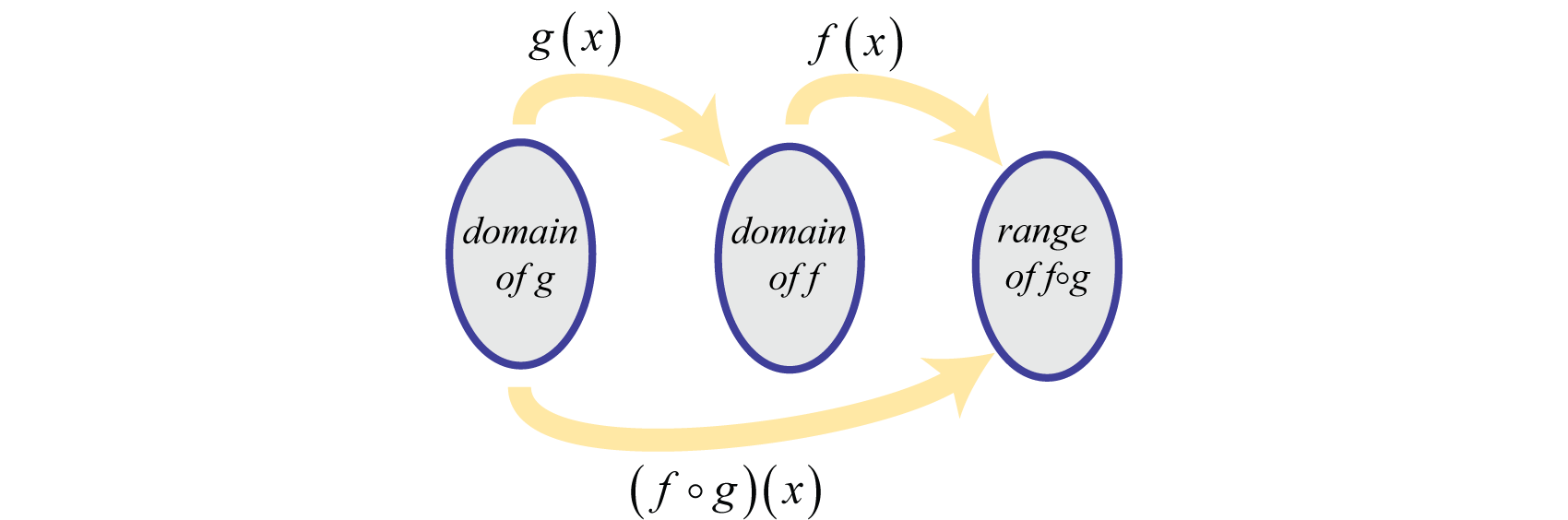### Example 1

Given $f(x)=x2−x+3$ and $g(x)=2x−1$ calculate:

1. $(f○g)(x).$
2. $(g○f)(x).$

Solution:

1. Substitute g into f.

$(f○g)(x)=f(g(x))=f(2x−1)=(2x−1)2−(2x−1)+3=4x2−4x+1−2x+1+3=4x2−6x+5$

2. Substitute f into g.

$(g○f)(x)=g(f(x))=g(x2−x+3)=2(x2−x+3)−1=2x2−2x+6−1=2x2−2x+5$

1. $(f○g)(x)=4x2−6x+5$
2. $(g○f)(x)=2x2−2x+5$

The previous example shows that composition of functions is not necessarily commutative.

### Example 2

Given $f(x)=x3+1$ and $g(x)=3x−13$ find $(f○g)(4).$

Solution:

Begin by finding $(f○g)(x).$

$(f○g)(x)=f(g(x))=f(3x−13)=(3x−13)3+1=3x−1+1=3x$

Next, substitute 4 in for x.

$(f○g)(x)=3x(f○g)(4)=3(4)=12$

Answer: $(f○g)(4)=12$

Functions can be composed with themselves.

### Example 3

Given $f(x)=x2−2$ find $(f○f)(x).$

Solution:

$(f○f)(x)=f(f(x))=f(x2−2)=(x2−2)2−2=x4−4x2+4−2=x4−4x2+2$

Answer: $(f○f)(x)=x4−4x2+2$

Try this! Given $f(x)=2x+3$ and $g(x)=x−1$ find $(f○g)(5).$

## Inverse Functions

Consider the function that converts degrees Fahrenheit to degrees Celsius: $C(x)=59(x−32).$ We can use this function to convert 77°F to degrees Celsius as follows.

$C(77)=59(77−32)=59(45)=25$

Therefore, 77°F is equivalent to 25°C. If we wish to convert 25°C back to degrees Fahrenheit we would use the formula: $F(x)=95x+32.$

$F(25)=95(25)+32=45+32=77$

Notice that the two functions $C$ and $F$ each reverse the effect of the other.This describes an inverse relationship. In general, f and g are inverse functions if,

$(f○g)(x)=f(g(x))=x for all x in the domain of g and(g○f)(x)=g(f(x))=x for all x in the domain of f.$

In this example,

$C(F(25))=C(77)=25F(C(77))=F(25)=77$

### Example 4

Verify algebraically that the functions defined by $f(x)=12x−5$ and $g(x)=2x+10$ are inverses.

Solution:

Compose the functions both ways and verify that the result is x.

 $(f○g)(x)=f(g(x))=f(2x+10)=12(2x+10)−5=x+5−5=x ✓$ $(g○f)(x)=g(f(x))=g(12x−5)=2(12x−5)+10=x−10+10=x ✓$

Answer: Both $(f○g)(x)=(g○f)(x)=x$; therefore, they are inverses.

Next we explore the geometry associated with inverse functions. The graphs of both functions in the previous example are provided on the same set of axes below.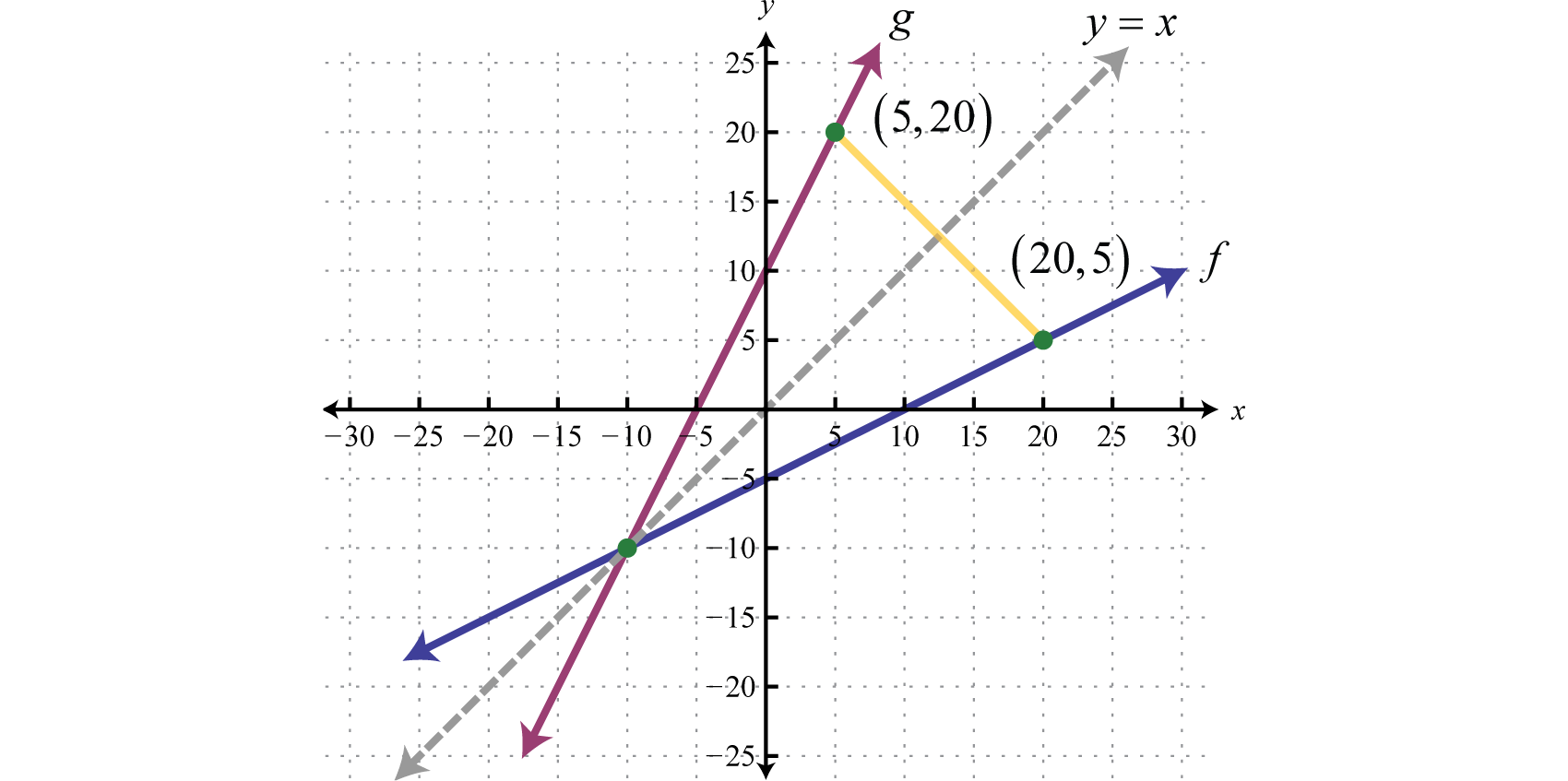Note that there is symmetry about the line $y=x$; the graphs of f and g are mirror images about this line. Also notice that the point (20, 5) is on the graph of f and that (5, 20) is on the graph of g. Both of these observations are true in general and we have the following properties of inverse functions:

1. The graphs of inverse functions are symmetric about the line $y=x.$
2. If $(a,b)$ is on the graph of a function, then $(b,a)$ is on the graph of its inverse.

Furthermore, if g is the inverse of f we use the notation $g=f−1.$ Here $f−1$ is read, “f inverse,” and should not be confused with negative exponents. In other words, $f−1(x)≠1f(x)$ and we have,

$(f○f−1)(x)=f(f−1(x))=x and(f−1○f)(x)=f−1(f(x))=x$

### Example 5

Verify algebraically that the functions defined by $f(x)=1x−2$ and $f−1(x)=1x+2$ are inverses.

Solution:

Compose the functions both ways to verify that the result is x.

 $(f○f−1)(x)=f(f−1(x))=f(1x+2)=1(1x+2)−2=x+21−2=x+2−2=x ✓$ $(f−1○f)(x)=f−1(f(x))=f−1(1x−2)=1(1x−2)+2=1 1x =x ✓$

Answer: Since $(f○f−1)(x)=(f−1○f)(x)=x$ they are inverses.

Recall that a function is a relation where each element in the domain corresponds to exactly one element in the range. We use the vertical line test to determine if a graph represents a function or not. Functions can be further classified using an inverse relationship. One-to-one functionsFunctions where each value in the range corresponds to exactly one value in the domain. are functions where each value in the range corresponds to exactly one element in the domain. The horizontal line testIf a horizontal line intersects the graph of a function more than once, then it is not one-to-one. is used to determine whether or not a graph represents a one-to-one function. If a horizontal line intersects a graph more than once, then it does not represent a one-to-one function.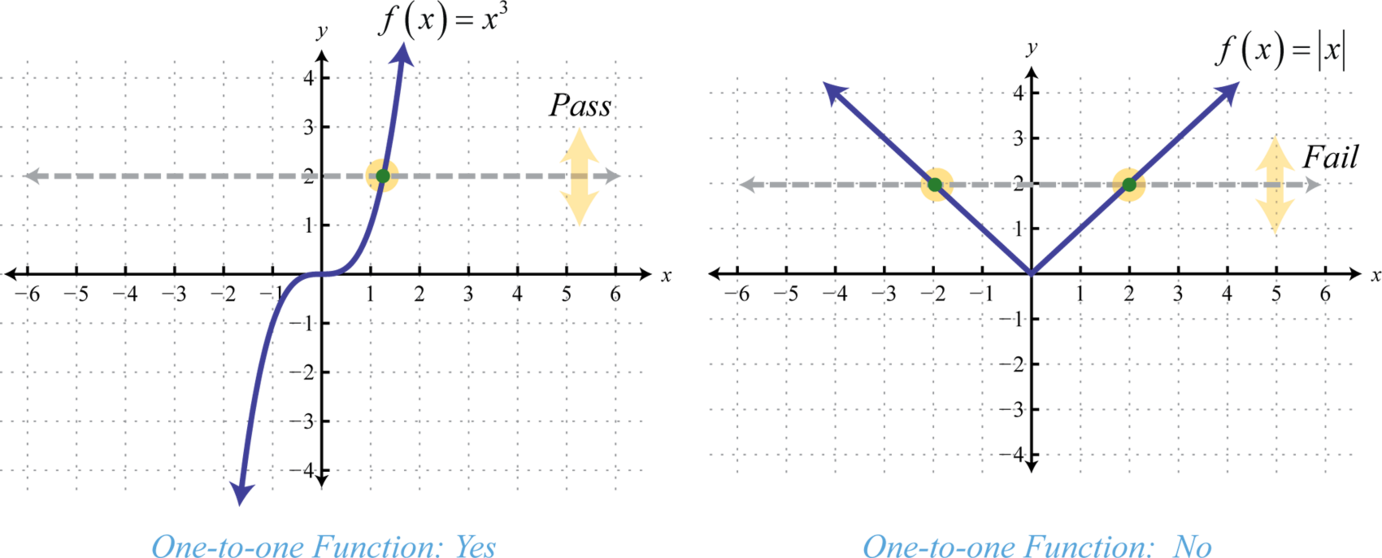The horizontal line represents a value in the range and the number of intersections with the graph represents the number of values it corresponds to in the domain. The function defined by $f(x)=x3$ is one-to-one and the function defined by $f(x)=|x|$ is not. Determining whether or not a function is one-to-one is important because a function has an inverse if and only if it is one-to-one. In other words, a function has an inverse if it passes the horizontal line test.

Note: In this text, when we say “a function has an inverse,” we mean that there is another function, $f−1$, such that $(f○f−1)(x)=(f−1○f)(x)=x.$

### Example 6

Determine whether or not the given function is one-to-one.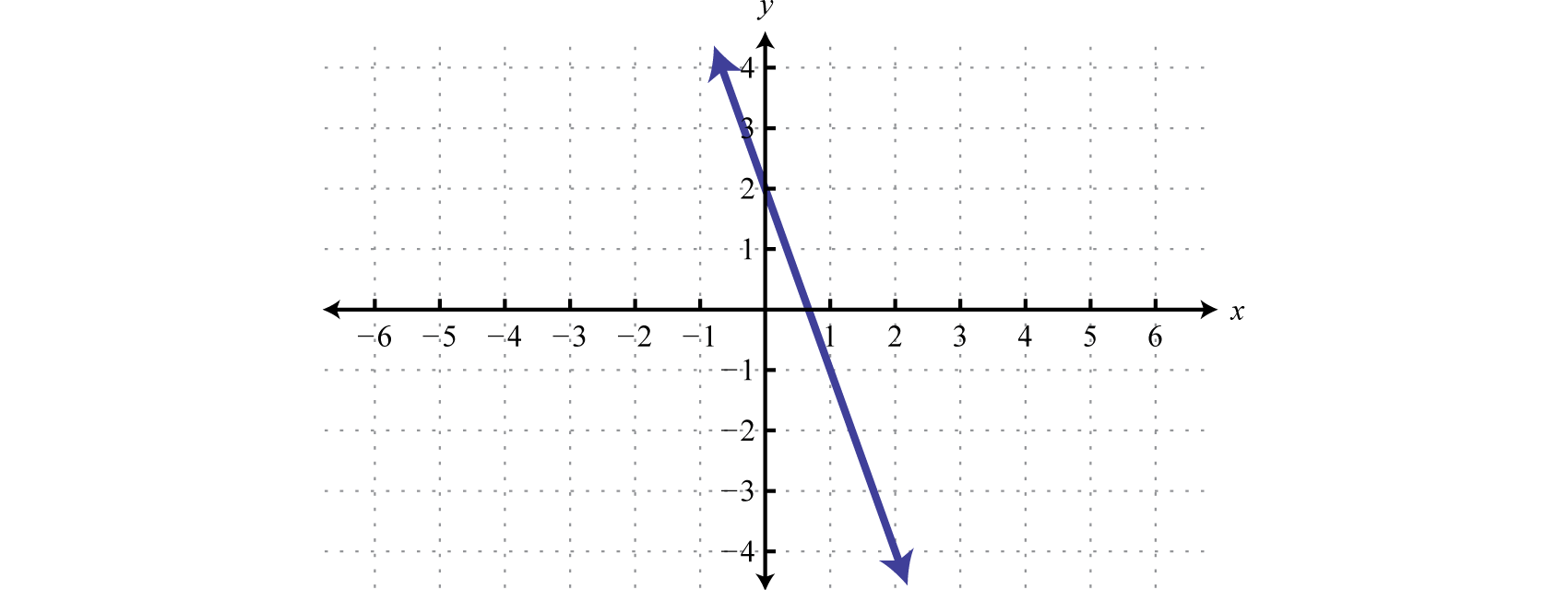Solution: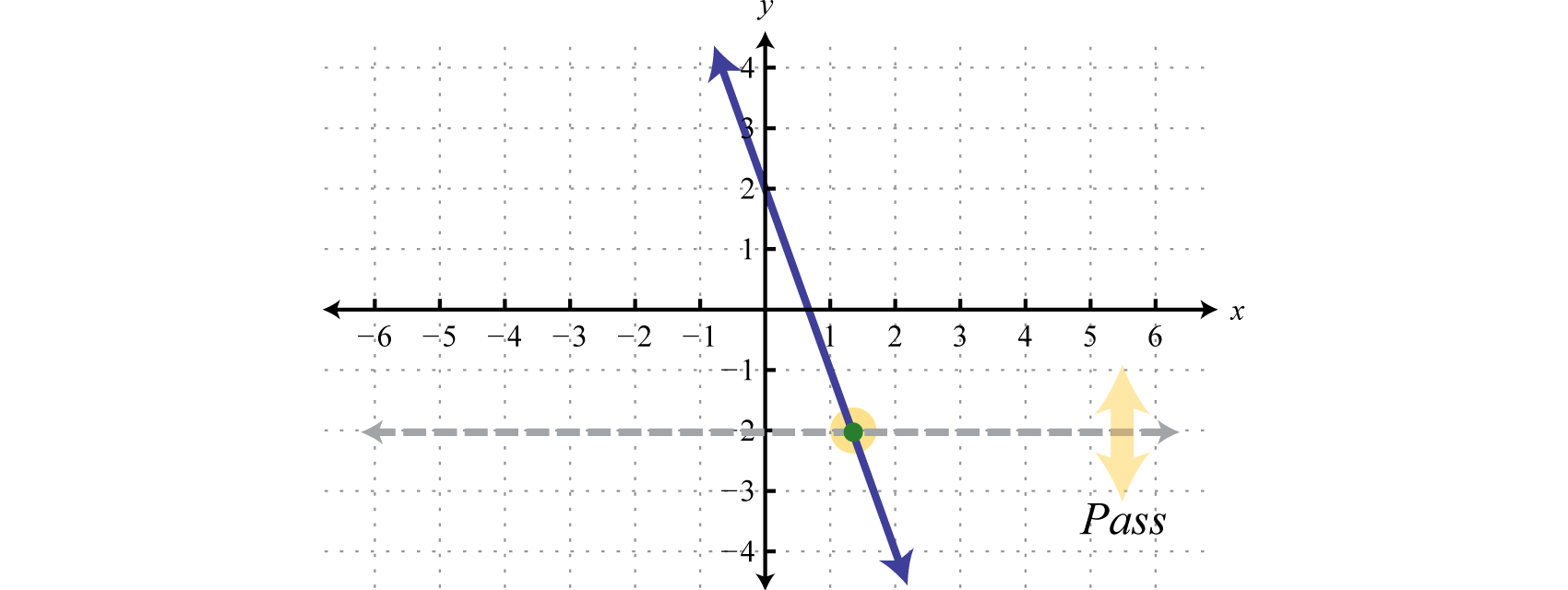Answer: The given function passes the horizontal line test and thus is one-to-one.

In fact, any linear function of the form $f(x)=mx+b$ where $m≠0$, is one-to-one and thus has an inverse. The steps for finding the inverse of a one-to-one function are outlined in the following example.

### Example 7

Find the inverse of the function defined by $f(x)=32x−5.$

Solution:

Before beginning this process, you should verify that the function is one-to-one. In this case, we have a linear function where $m≠0$ and thus it is one-to-one.

• Step 1: Replace the function notation $f(x)$ with y.

$f(x)=32x−5y=32x−5$

• Step 2: Interchange x and y. We use the fact that if $(x,y)$ is a point on the graph of a function, then $(y,x)$ is a point on the graph of its inverse.

$x=32y−5$

• Step 3: Solve for y.

$x=32y−5x+5=32y23⋅(x+5)=23⋅32y23x+103=y$

• Step 4: The resulting function is the inverse of f. Replace y with $f−1(x).$

$f−1(x)=23x+103$

• Step 5: Check.

 $(f○f−1)(x)=f(f−1(x))=f(23x+103)=32(23x+103)−5=x+5−5=x ✓$ $(f−1○f)(x)=f−1(f(x))=f−1(32x−5)=23(32x−5)+103=x−103+103=x ✓$

Answer: $f−1(x)=23x+103$

If a function is not one-to-one, it is often the case that we can restrict the domain in such a way that the resulting graph is one-to-one. For example, consider the squaring function shifted up one unit, $g(x)=x2+1.$ Note that it does not pass the horizontal line test and thus is not one-to-one. However, if we restrict the domain to nonnegative values, $x≥0$, then the graph does pass the horizontal line test.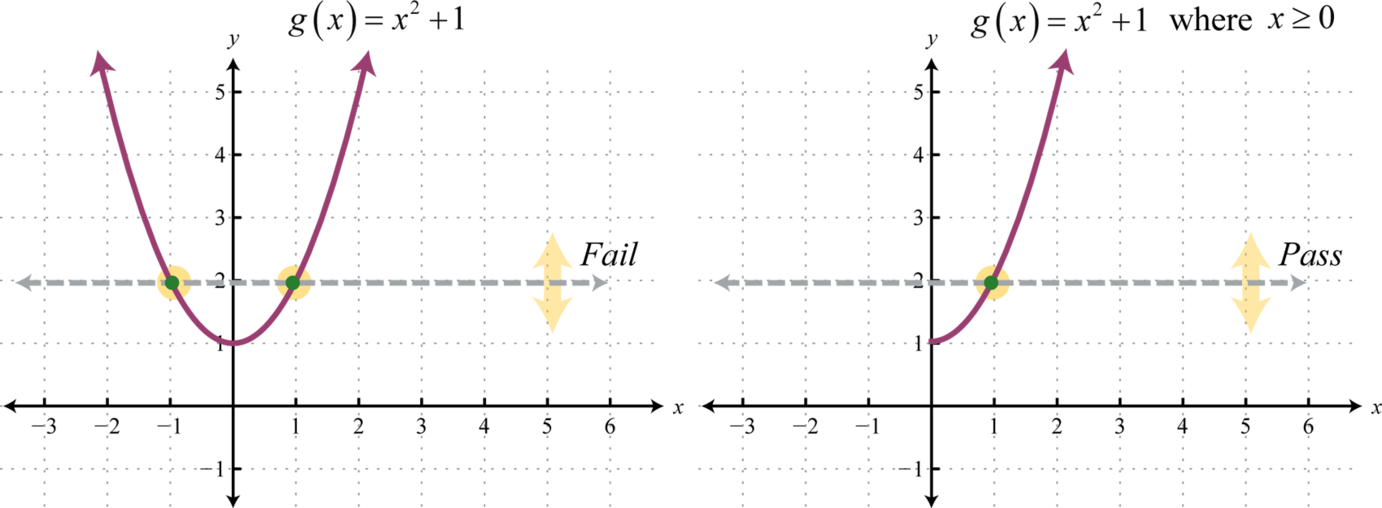On the restricted domain, g is one-to-one and we can find its inverse.

### Example 8

Find the inverse of the function defined by $g(x)=x2+1$ where $x≥0.$

Solution:

Begin by replacing the function notation $g(x)$ with y.

$g(x)=x2+1y=x2+1 where x≥0$

Interchange x and y.

$x=y2+1 where y≥0$

Solve for y.

$x=y2+1x−1=y2±x−1=y$

Since $y≥0$ we only consider the positive result.

$y=x−1g−1(x)=x−1$

Answer: $g−1(x)=x−1.$ The check is left to the reader.

The graphs in the previous example are shown on the same set of axes below. Take note of the symmetry about the line $y=x.$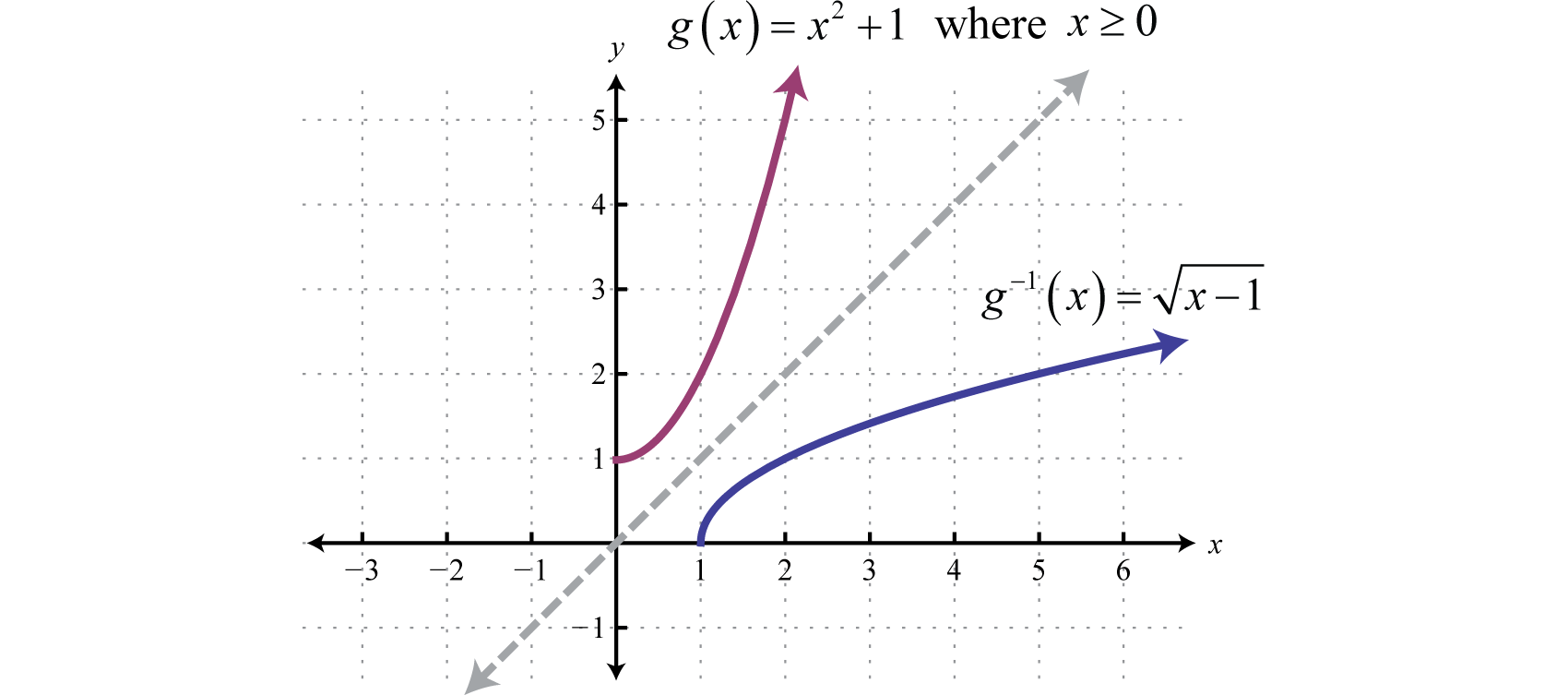### Example 9

Find the inverse of the function defined by $f(x)=2x+1x−3.$

Solution:

Use a graphing utility to verify that this function is one-to-one. Begin by replacing the function notation $f(x)$ with y.

$f(x)=2x+1x−3y=2x+1x−3$

Interchange x and y.

$x=2y+1y−3$

Solve for y.

$x=2y+1y−3x(y−3)=2y+1xy−3x=2y+1$

Obtain all terms with the variable y on one side of the equation and everything else on the other. This will enable us to treat y as a GCF.

$xy−3x=2y+1xy−2y=3x+1y(x−2)=3x+1y=3x+1x−2$

Answer: $f−1(x)=3x+1x−2.$ The check is left to the reader.

Try this! Find the inverse of $f(x)=x+13−3.$

Answer: $f−1(x)=(x+3)3−1$

### Key Takeaways

• The composition operator ($○$) indicates that we should substitute one function into another. In other words, $(f○g)(x)=f(g(x))$ indicates that we substitute $g(x)$ into $f(x).$
• If two functions are inverses, then each will reverse the effect of the other. Using notation, $(f○g)(x)=f(g(x))=x$ and $(g○f)(x)=g(f(x))=x.$
• Inverse functions have special notation. If $g$ is the inverse of $f$, then we can write $g(x)=f−1(x).$ This notation is often confused with negative exponents and does not equal one divided by $f(x).$
• The graphs of inverses are symmetric about the line $y=x.$ If $(a,b)$ is a point on the graph of a function, then $(b,a)$ is a point on the graph of its inverse.
• If each point in the range of a function corresponds to exactly one value in the domain then the function is one-to-one. Use the horizontal line test to determine whether or not a function is one-to-one.
• A one-to-one function has an inverse, which can often be found by interchanging x and y, and solving for y. This new function is the inverse of the original function.

### Part A: Composition of Functions

Given the functions defined by f and g find $(f○g)(x)$ and $(g○f)(x).$

1. $f(x)=4x−1$, $g(x)=3x$

2. $f(x)=−2x+5$, $g(x)=2x$

3. $f(x)=3x−5$, $g(x)=x−4$

4. $f(x)=5x+1$, $g(x)=2x−3$

5. $f(x)=x2−x+1$, $g(x)=2x−1$

6. $f(x)=x2−3x−2$, $g(x)=x−2$

7. $f(x)=x2+3$, $g(x)=x2−5$

8. $f(x)=2x2$, $g(x)=x2−x$

9. $f(x)=8x3+5$, $g(x)=x−53$

10. $f(x)=27x3−1$, $g(x)=x+13$

11. $f(x)=1x+5$, $g(x)=1x$

12. $f(x)=1x−3$, $g(x)=3x+3$

13. $f(x)=5x$, $g(x)=3x−2$

14. $f(x)=2x$, $g(x)=4x+1$

15. $f(x)=12x$, $g(x)=x2+8$

16. $f(x)=2x−1$, $g(x)=1x+1$

17. $f(x)=1−x2x$, $g(x)=12x+1$

18. $f(x)=2xx+1$, $g(x)=x+1x$

Given the functions defined by $f(x)=3x2−2$, $g(x)=5x+1$, and $h(x)=x$, calculate the following.

1. $(f○g)(2)$

2. $(g○f)(−1)$

3. $(g○f)(0)$

4. $(f○g)(0)$

5. $(f○h)(3)$

6. $(g○h)(16)$

7. $(h○g)(35)$

8. $(h○f)(−3)$

Given the functions defined by $f(x)=x+33$, $g(x)=8x3−3$, and $h(x)=2x−1$, calculate the following.

1. $(f○g)(1)$

2. $(g○f)(−2)$

3. $(g○f)(0)$

4. $(f○g)(−2)$

5. $(f○h)(−1)$

6. $(h○g)(−12)$
7. $(h○f)(24)$

8. $(g○h)(0)$

Given the function, determine $(f○f)(x).$

1. $f(x)=3x−1$

2. $f(x)=25x+1$

3. $f(x)=x2+5$

4. $f(x)=x2−x+6$

5. $f(x)=x3+2$

6. $f(x)=x3−x$

7. $f(x)=1x+1$

8. $f(x)=x+12x$

### Part B: Inverse Functions

Are the given functions one-to-one? Explain.

1.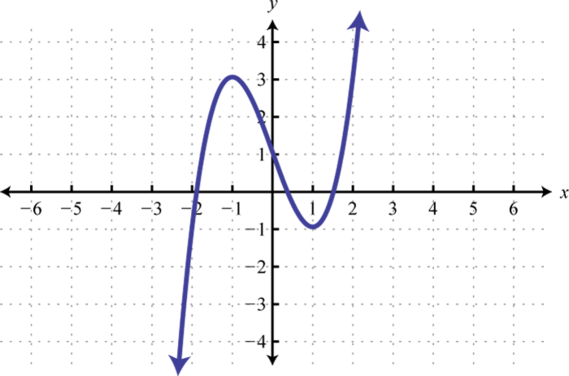2.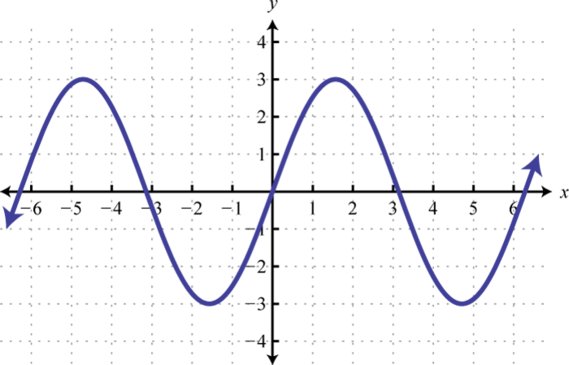3.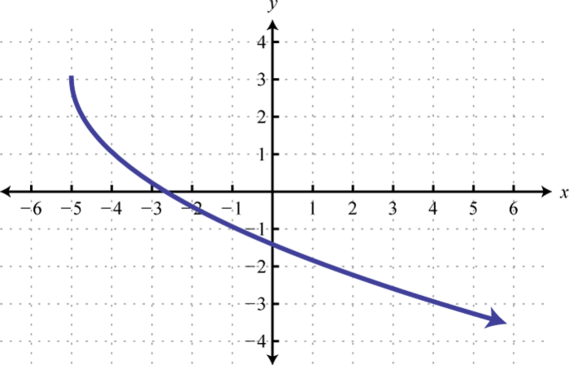4.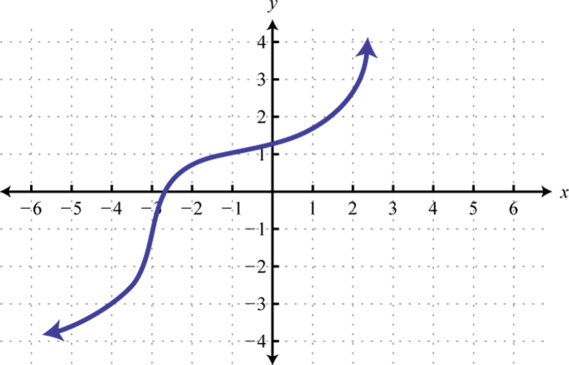5. $f(x)=x+1$

6. $g(x)=x2+1$

7. $h(x)=|x|+1$

8. $r(x)=x3+1$

9. $f(x)=x+1$

10. $g(x)=3$

Given the graph of a one-to-one function, graph its inverse.

1.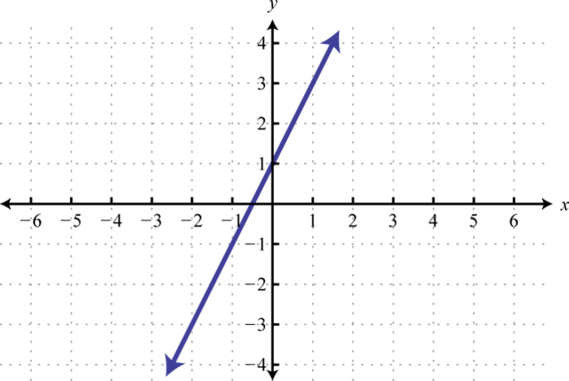2.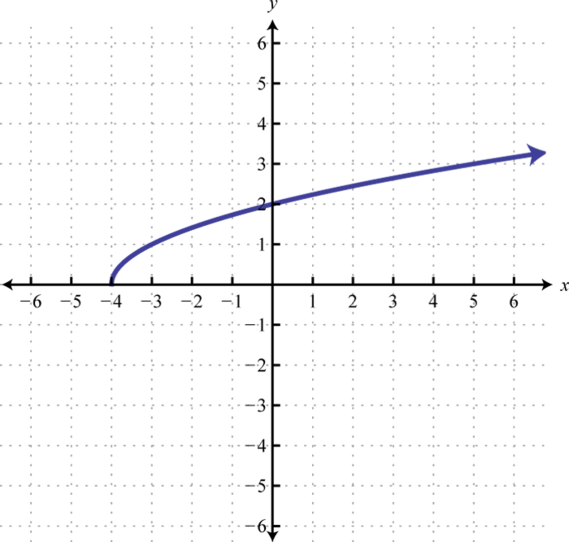3.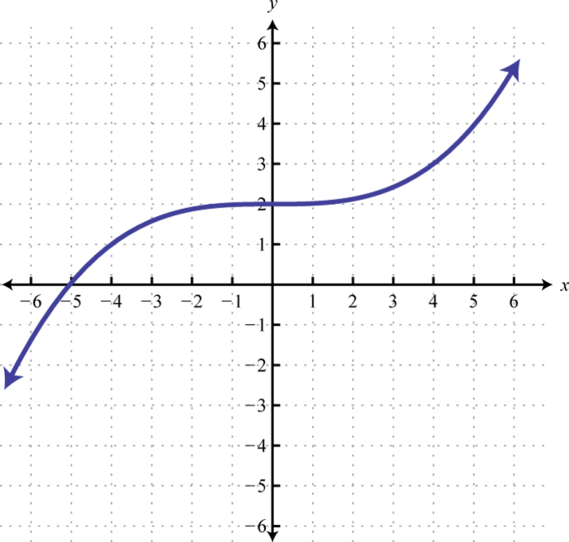4.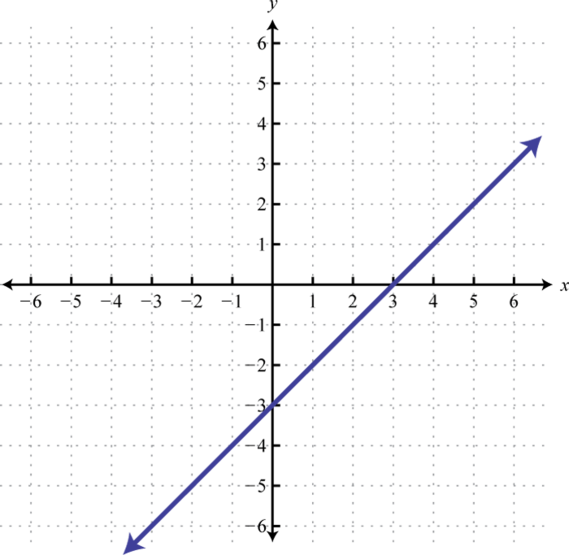5.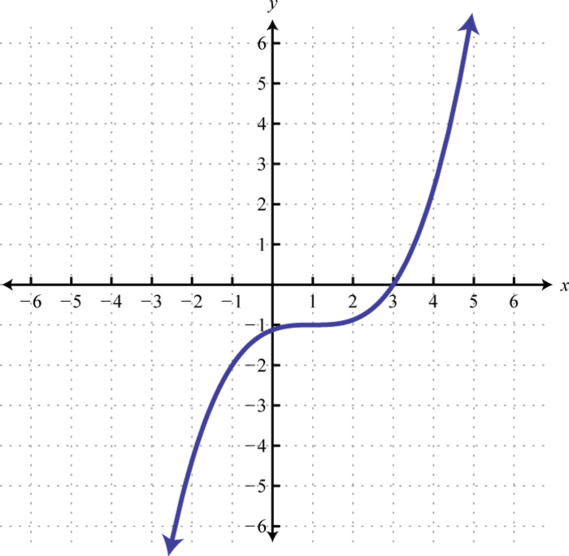6.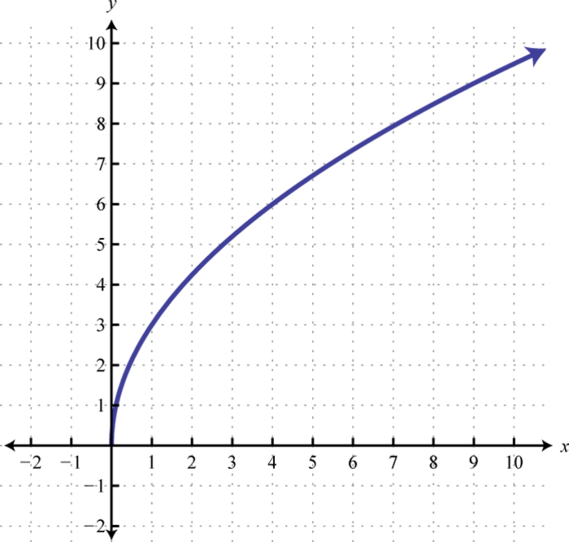Verify algebraically that the two given functions are inverses. In other words, show that $(f○f−1)(x)=x$ and $(f−1○f)(x)=x.$

1. $f(x)=3x−4$, $f−1(x)=x+43$

2. $f(x)=−5x+1$, $f−1(x)=1−x5$

3. $f(x)=−23x+1$, $f−1(x)=−32x+32$

4. $f(x)=4x−13$, $f−1(x)=14x+112$

5. $f(x)=x−8$, $f−1(x)=x2+8$, $x≥0$

6. $f(x)=6x3−3$, $f−1(x)=(x+3)36$

7. $f(x)=xx+1$, $f−1(x)=x1−x$

8. $f(x)=x−33x$, $f−1(x)=31−3x$

9. $f(x)=2(x−1)3+3$, $f−1(x)=1+x−323$

10. $f(x)=5x−13+4$, $f−1(x)=(x−4)3+15$

Find the inverses of the following functions.

1. $f(x)=5x$

2. $f(x)=12x$

3. $f(x)=2x+5$

4. $f(x)=−4x+3$

5. $f(x)=−23x+13$

6. $f(x)=−12x+34$

7. $g(x)=x2+5$, $x≥0$

8. $g(x)=x2−7$, $x≥0$

9. $f(x)=(x−5)2$, $x≥5$

10. $f(x)=(x+1)2$, $x≥−1$

11. $h(x)=3x3+5$

12. $h(x)=2x3−1$

13. $f(x)=(2x−3)3$

14. $f(x)=(x+4)3−1$

15. $g(x)=2x3+1$
16. $g(x)=1x3−2$
17. $f(x)=5x+1$
18. $f(x)=12x−9$
19. $f(x)=x+5x−5$
20. $f(x)=3x−42x−1$
21. $h(x)=x−510x$
22. $h(x)=9x+13x$
23. $g(x)=5x+23$

24. $g(x)=4x−33$

25. $f(x)=x−63−4$

26. $f(x)=2x+23+5$

27. $h(x)=x+15−3$

28. $h(x)=x−85+1$

29. $f(x)=mx+b$, $m≠0$

30. $f(x)=ax2+c$, $x≥0$

31. $f(x)=ax3+d$

32. $f(x)=a(x−h)2+k$, $x≥h$

Graph the function and its inverse on the same set of axes.

1. $f(x)=x+2$

2. $f(x)=23x−4$

3. $f(x)=−2x+2$

4. $f(x)=−13x+4$

5. $g(x)=x2−2$, $x≥0$

6. $g(x)=(x−2)2$, $x≥2$

7. $h(x)=x3+1$

8. $h(x)=(x+2)3−2$

9. $f(x)=2−x$

10. $f(x)=−x+1$

### Part C: Discussion Board

1. Is composition of functions associative? Explain.

2. Explain why $C(x)=59(x−32)$ and $F(x)=95x+32$ define inverse functions. Prove it algebraically.

3. Do the graphs of all straight lines represent one-to-one functions? Explain.

4. If the graphs of inverse functions intersect, then how can we find the point of intersection? Explain.

1. $(f○g)(x)=12x−1$; $(g○f)(x)=12x−3$

2. $(f○g)(x)=3x−17$; $(g○f)(x)=3x−9$

3. $(f○g)(x)=4x2−6x+3$; $(g○f)(x)=2x2−2x+1$

4. $(f○g)(x)=x4−10x2+28$; $(g○f)(x)=x4+6x2+4$

5. $(f○g)(x)=8x−35$; $(g○f)(x)=2x$

6. $(f○g)(x)=x5x+1$; $(g○f)(x)=x+5$

7. $(f○g)(x)=53x−2$; $(g○f)(x)=15x−2$

8. $(f○g)(x)=12x2+16;$ $(g○f)(x)=1+32x24x2$
9. $(f○g)(x)=x$; $(g○f)(x)=x$

10. 361

11. −9

12. 7

13. 2

14. 2

15. 21

16. 0

17. 5

18. $(f○f)(x)=9x−4$

19. $(f○f)(x)=x4+10x2+30$

20. $(f○f)(x)=x9+6x6+12x3+10$

21. $(f○f)(x)=x+1x+2$

1. No, fails the HLT

2. Yes, passes the HLT

3. Yes, its graph passes the HLT.

4. No, its graph fails the HLT.

5. Yes, its graph passes the HLT.

6.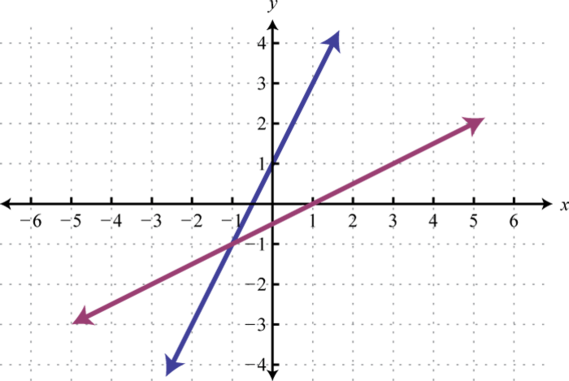7.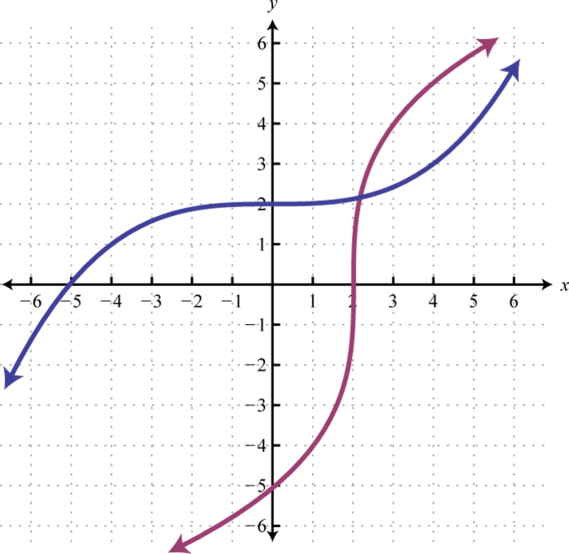8.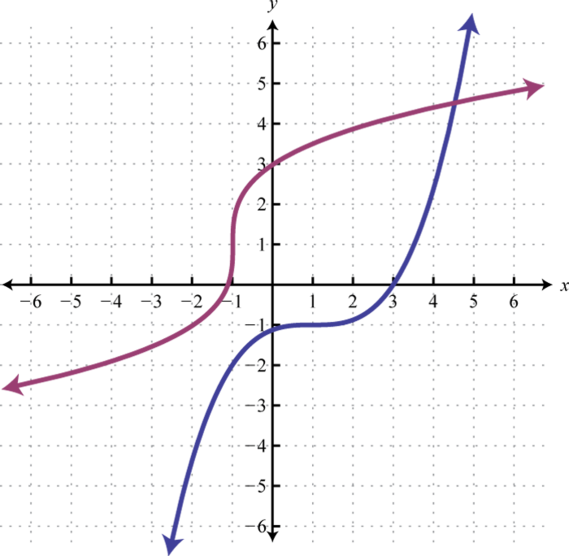9. Proof

10. Proof

11. Proof

12. Proof

13. Proof

14. $f−1(x)=x5$

15. $f−1(x)=12x−52$
16. $f−1(x)=−32x+12$
17. $g−1(x)=x−5$

18. $f−1(x)=x+5$

19. $h−1(x)=x−533$
20. $f−1(x)=x3+32$
21. $g−1(x)=2−xx3$
22. $f−1(x)=5−xx$
23. $f−1(x)=5(x+1)x−1$
24. $h−1(x)=−510x−1$
25. $g−1(x)=x3−25$
26. $f−1(x)=(x+4)3+6$

27. $h−1(x)=(x+3)5−1$

28. $f−1(x)=x−bm$
29. $f−1(x)=x−da3$
30.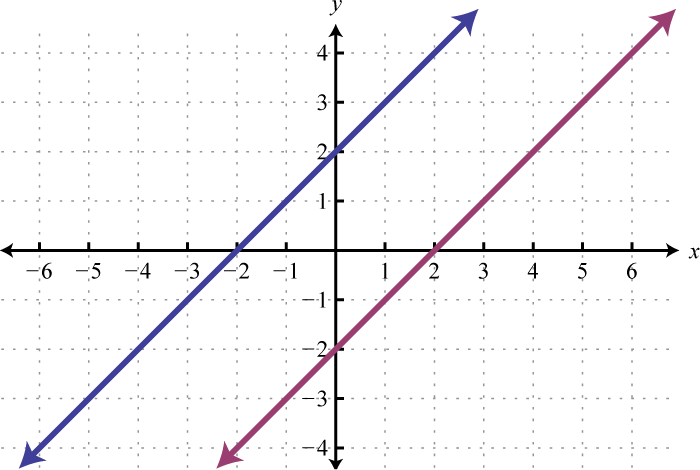31.32.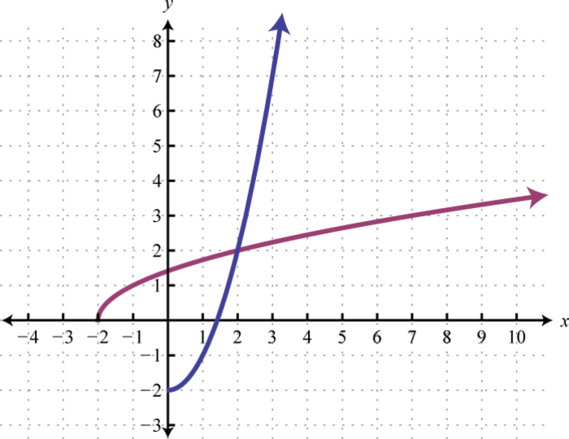33.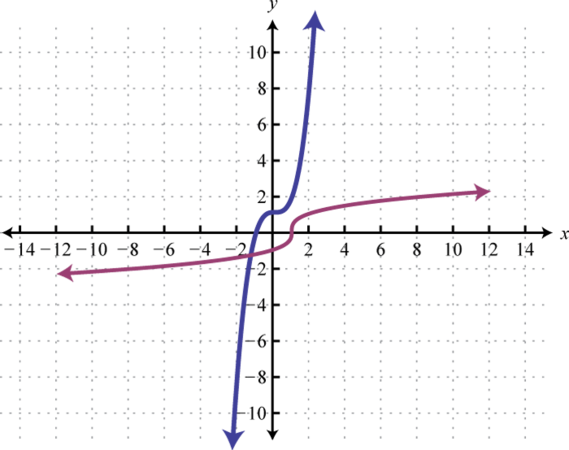34.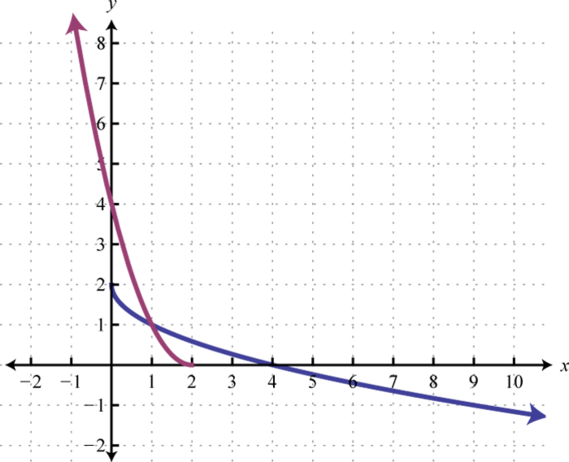## 7.2 Exponential Functions and Their Graphs

### Learning Objectives

1. Identify and evaluate exponential functions.
2. Sketch the graph of exponential functions and determine the domain and range.
3. Identify and graph the natural exponential function.
4. Apply the formulas for compound interest.

## Exponential Functions

At this point in our study of algebra we begin to look at transcendental functions or functions that seem to “transcend” algebra. We have studied functions with variable bases and constant exponents such as $x2$ or $y−3.$ In this section we explore functions with a constant base and variable exponents. Given a real number $b>0$ where $b≠1$ an exponential functionAny function with a definition of the form $f(x)=bx$ where $b>0$ and $b≠1.$ has the form,

$f(x)=bx Exponential Function$

For example, if the base b is equal to 2, then we have the exponential function defined by $f(x)=2x.$ Here we can see the exponent is the variable. Up to this point, rational exponents have been defined but irrational exponents have not. Consider $27$, where the exponent is an irrational number in the range,

$2.64<7<2.65$

We can use these bounds to estimate $27$,

$22.64<27<22.656.23<27<6.28$

Using rational exponents in this manner, an approximation of $27$ can be obtained to any level of accuracy. On a calculator,

$2^7≈6.26$

Therefore the domain of any exponential function consists of all real numbers $(−∞,∞).$ Choose some values for x and then determine the corresponding y-values.

$xyf(x)=2xSolutions−214y=2−2=122=14(−2, 14)−112y=2−1=121=12(−1, 12)01y=20=1(0, 1)12y=21=2(1, 2)24y=22=4(2, 4)76.26y=27≈6.26(2.65, 6.26)$

Because exponents are defined for any real number we can sketch the graph using a continuous curve through these given points: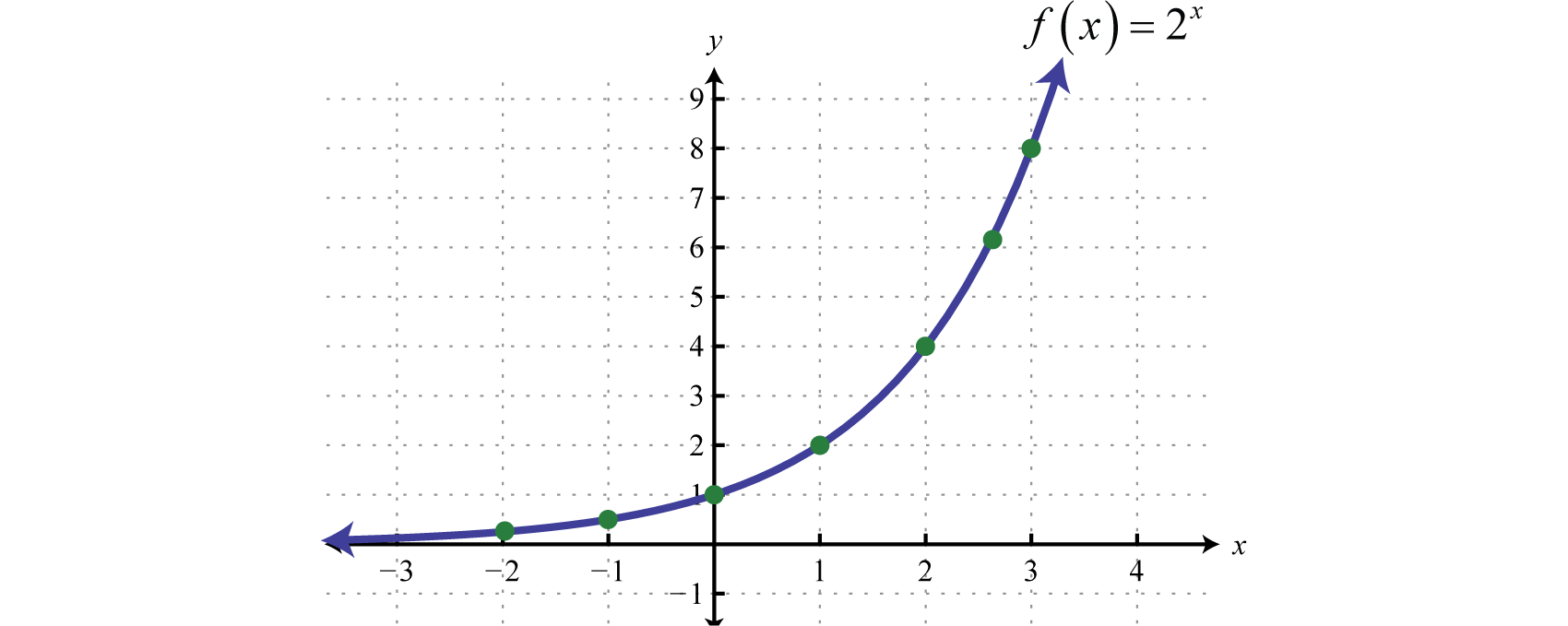It is important to point out that as x approaches negative infinity, the results become very small but never actually attain zero. For example,

$f(−5)=2−5=125≈0.03125f(−10)=2−10=1210≈0.0009766f(−15)=2−15=12−15≈.00003052$

This describes a horizontal asymptote at $y=0$, the x-axis, and defines a lower bound for the range of the function: $(0,∞).$

The base b of an exponential function affects the rate at which it grows. Below we have graphed $y=2x$, $y=3x$, and $y=10x$ on the same set of axes.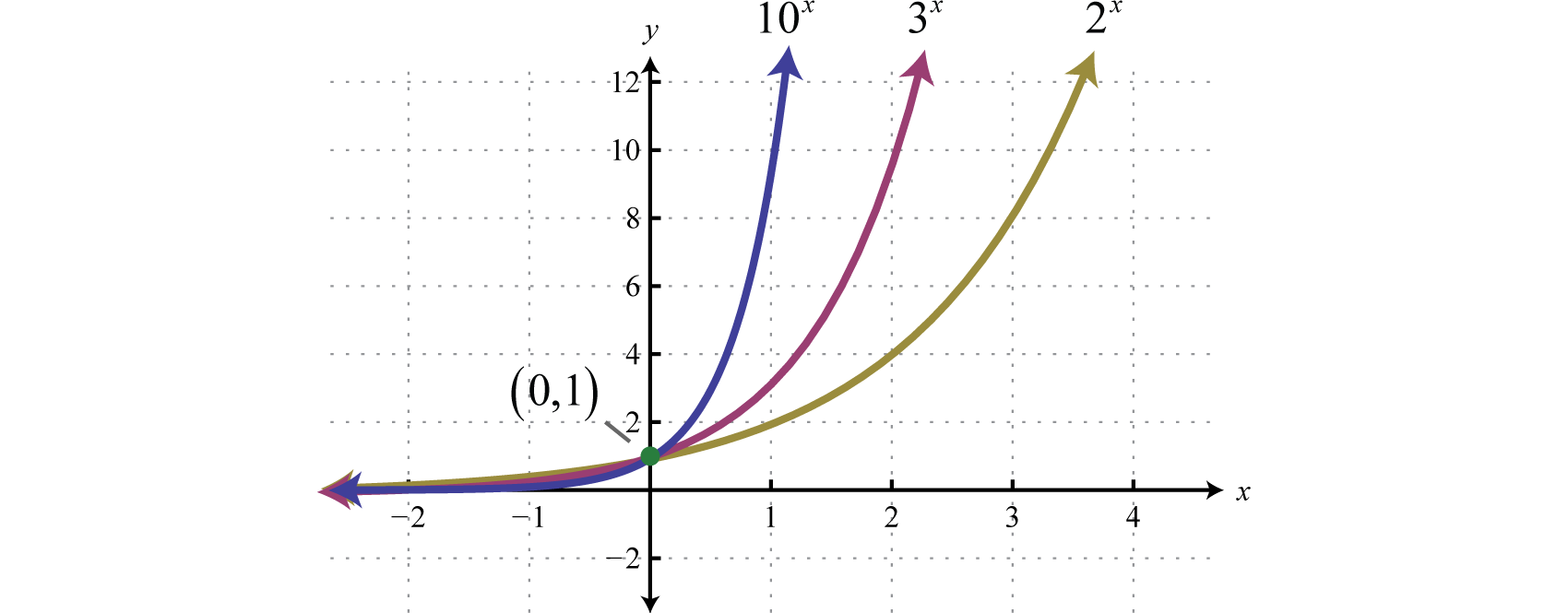Note that all of these exponential functions have the same y-intercept, namely $(0,1).$ This is because $f(0)=b0=1$ for any function defined using the form $f(x)=bx.$ As the functions are read from left to right, they are interpreted as increasing or growing exponentially. Furthermore, any exponential function of this form will have a domain that consists of all real numbers $(−∞,∞)$ and a range that consists of positive values $(0,∞)$ bounded by a horizontal asymptote at $y=0.$

### Example 1

Sketch the graph and determine the domain and range: $f(x)=10x+5.$

Solution:

The base 10 is used often, most notably with scientific notation. Hence, 10 is called the common base. In fact, the exponential function $y=10x$ is so important that you will find a button $10x$ dedicated to it on most modern scientific calculators. In this example, we will sketch the basic graph $y=10x$ and then shift it up 5 units.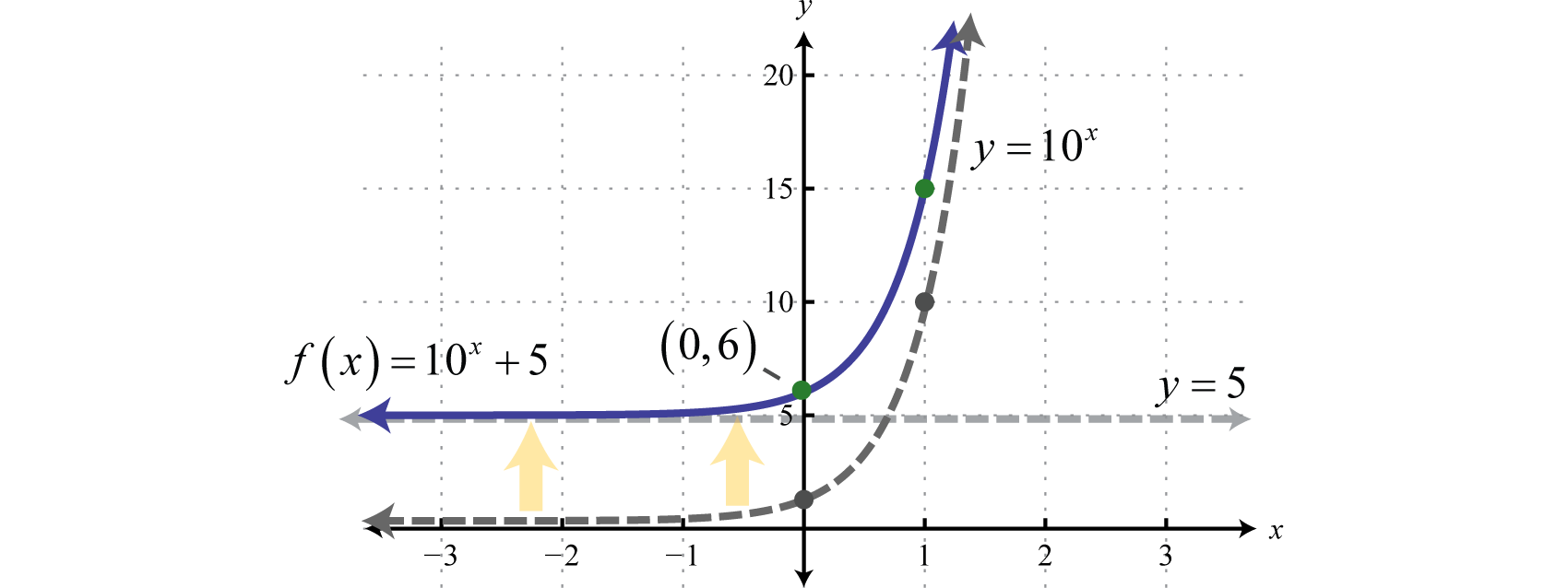Note that the horizontal asymptote of the basic graph $y=10x$ was shifted up 5 units to $y=5$ (shown dashed). Take a minute to evaluate a few values of x with your calculator and convince yourself that the result will never be less than 5.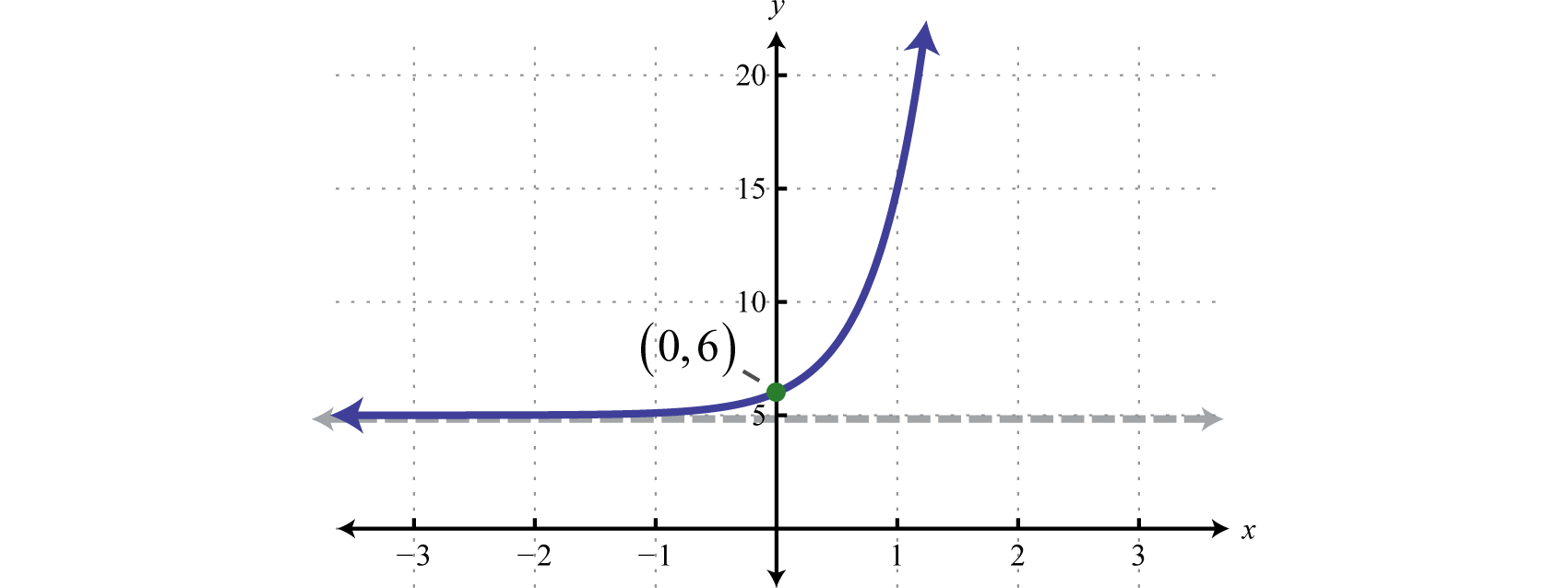Domain: $(−∞,∞)$; Range: $(5,∞)$

Next consider exponential functions with fractional bases $0 For example, $f(x)=(12)x$ is an exponential function with base $b=12.$

$xyf(x)=(12)xSolutions−24f(12)=(12)−2=1−22−2=2212=4(−2, 4)−12f(12)=(12)−1=1−12−1=2111=2(−1, 2)01f(12)=(12)0=1(0, 1)112f(12)=(12)1=12(1, 12)214f(12)=(12)2=14(2, 14)$

Plotting points we have,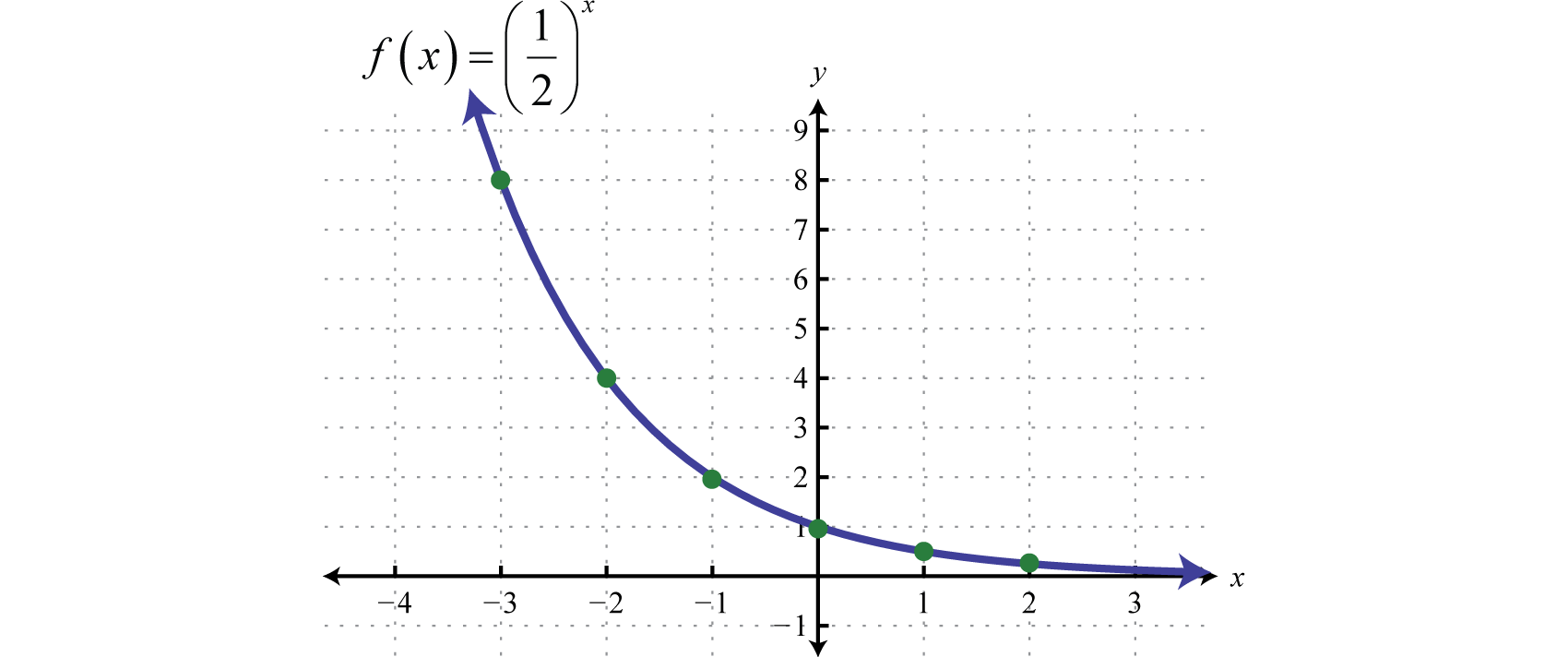Reading the graph from left to right, it is interpreted as decreasing exponentially. The base affects the rate at which the exponential function decreases or decays. Below we have graphed $y=(12)x$, $y=(13)x$, and $y=(110)x$ on the same set of axes.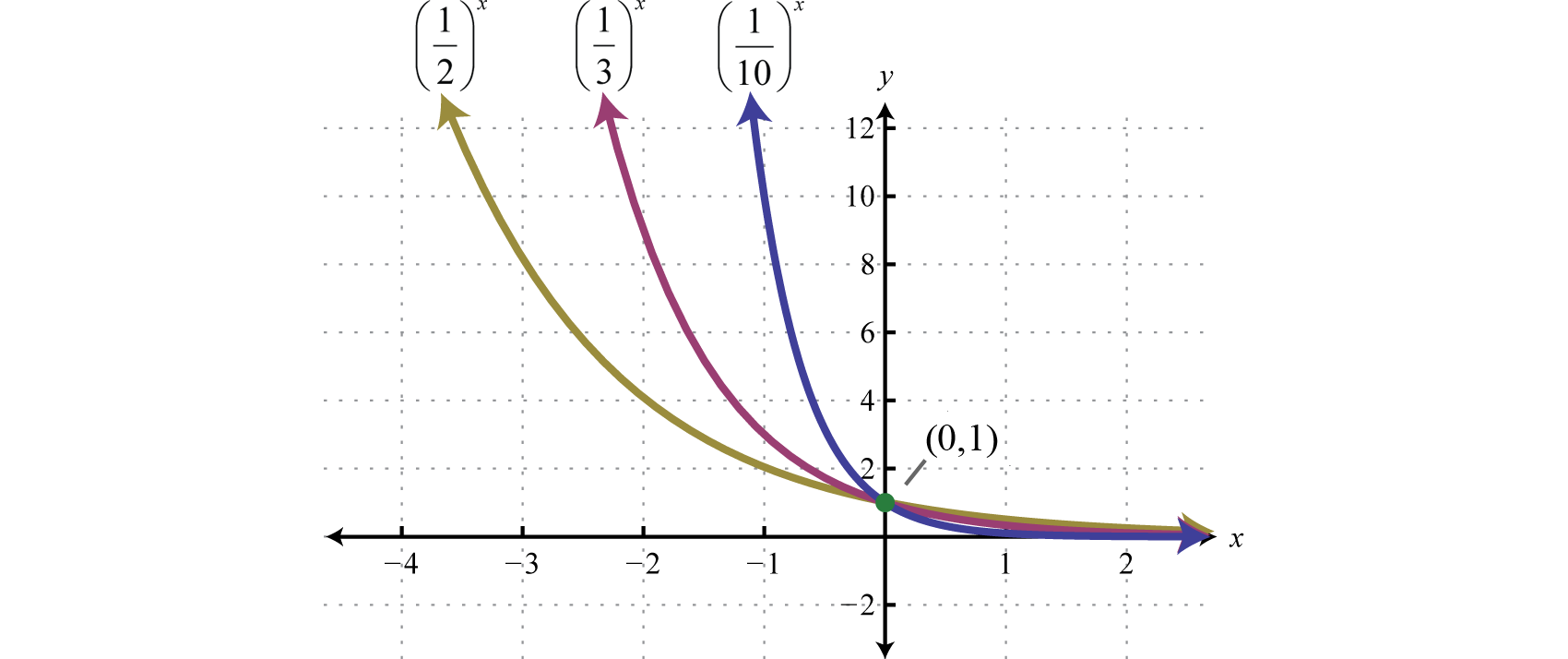Recall that $x−1=1x$ and so we can express exponential functions with fractional bases using negative exponents. For example,

$g(x)=(12)x=1x2x=12x=2−x.$

Furthermore, given that $f(x)=2x$ we can see $g(x)=f(−x)=2−x$ and can consider g to be a reflection of f about the y-axis.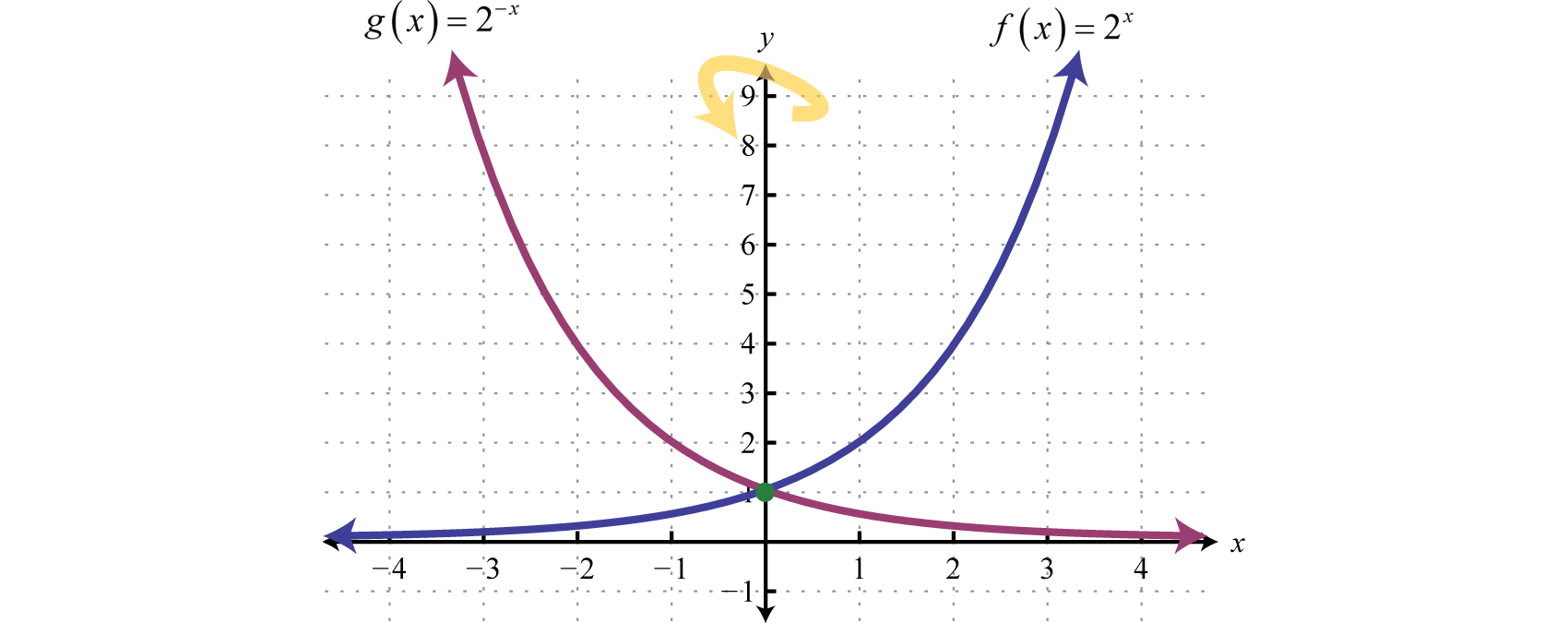In summary, given $b>0$And for both cases,

$Domain:(−∞,∞)Range:​(0,∞)y-intercept:(0,1)Asymptote:​​​y=0$

Furthermore, note that the graphs pass the horizontal line test and thus exponential functions are one-to-one. We use these basic graphs, along with the transformations, to sketch the graphs of exponential functions.

### Example 2

Sketch the graph and determine the domain and range: $f(x)=5−x−10.$

Solution:

Begin with the basic graph $y=5−x$ and shift it down 10 units.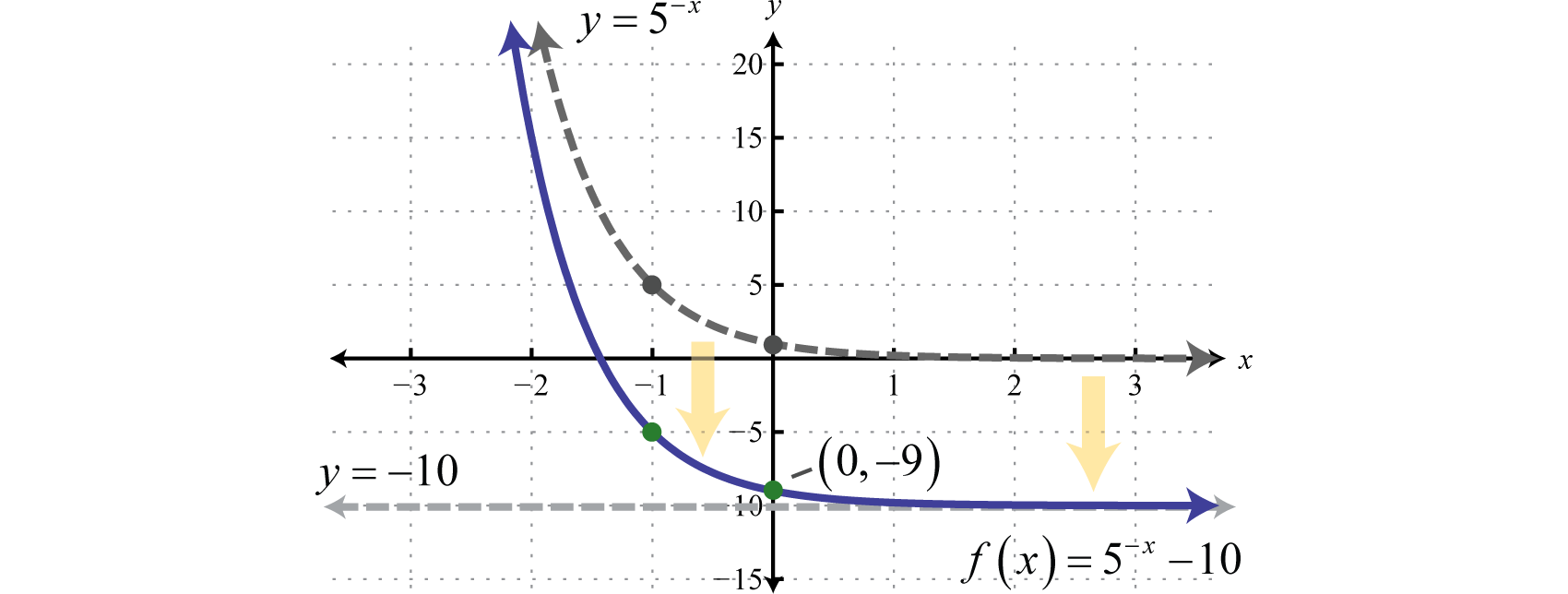The y-intercept is $(0,−9)$ and the horizontal asymptote is $y=−10.$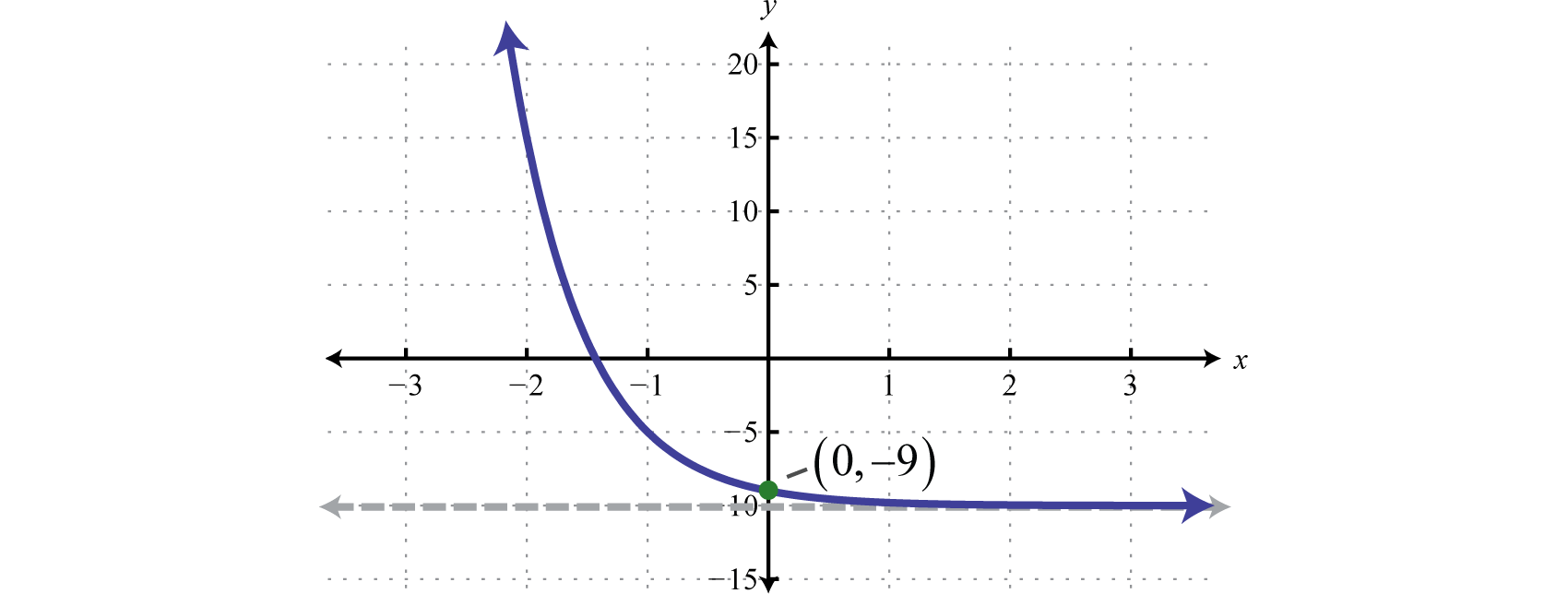Domain: $(−∞,∞)$; Range: $(−10,∞)$

Note: Finding the x-intercept of the graph in the previous example is left for a later section in this chapter. For now, we are more concerned with the general shape of exponential functions.

### Example 3

Sketch the graph and determine the domain and range: $g(x)=−2x−3.$

Solution:

Begin with the basic graph $y=2x$ and identify the transformations.

$y=2x Basic graphy=−2x Reflection about the x-axisy=−2x−3 Shift right 3 units$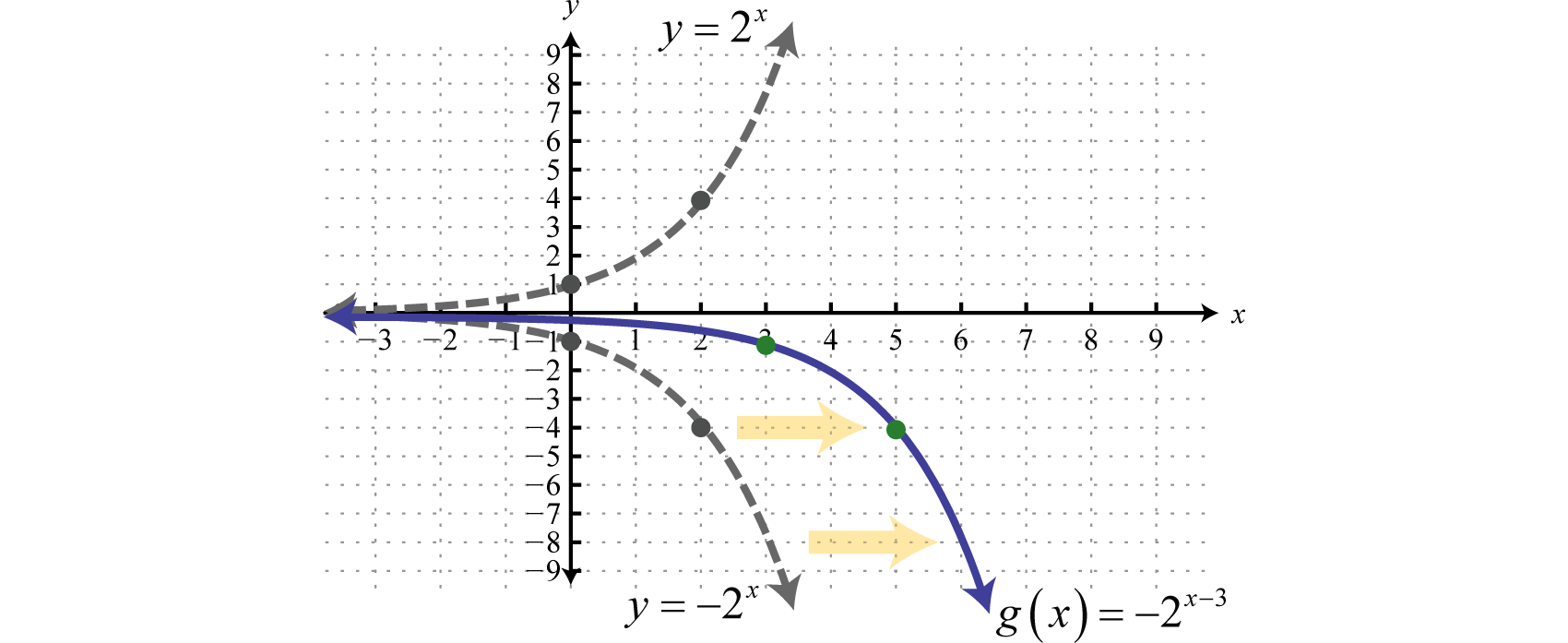Note that the horizontal asymptote remains the same for all of the transformations. To finish we usually want to include the y-intercept. Remember that to find the y-intercept we set $x=0.$

$g(0)=−20−3=−2−3=−123=−18$

Therefore the y-intercept is $(0, −18)$.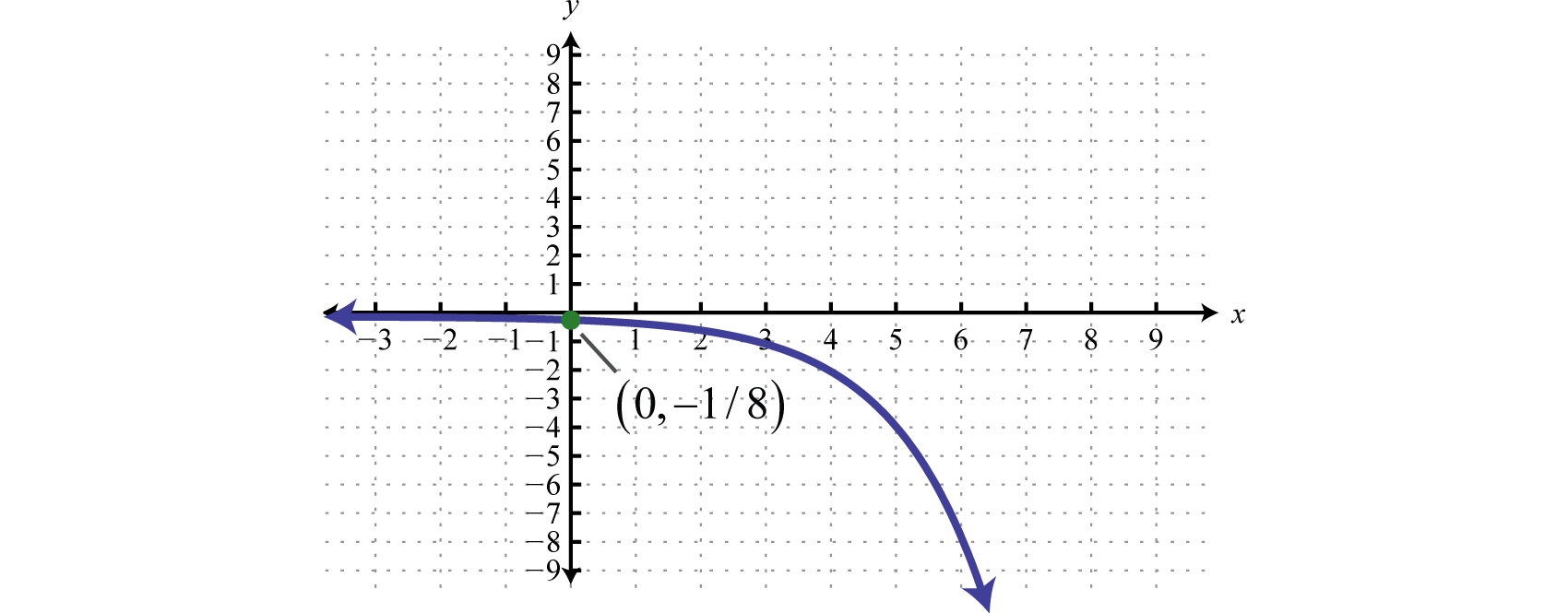Domain: $(−∞,∞)$; Range: $(−∞,0)$

Try this! Sketch the graph and determine the domain and range: $f(x)=2x−1+3.$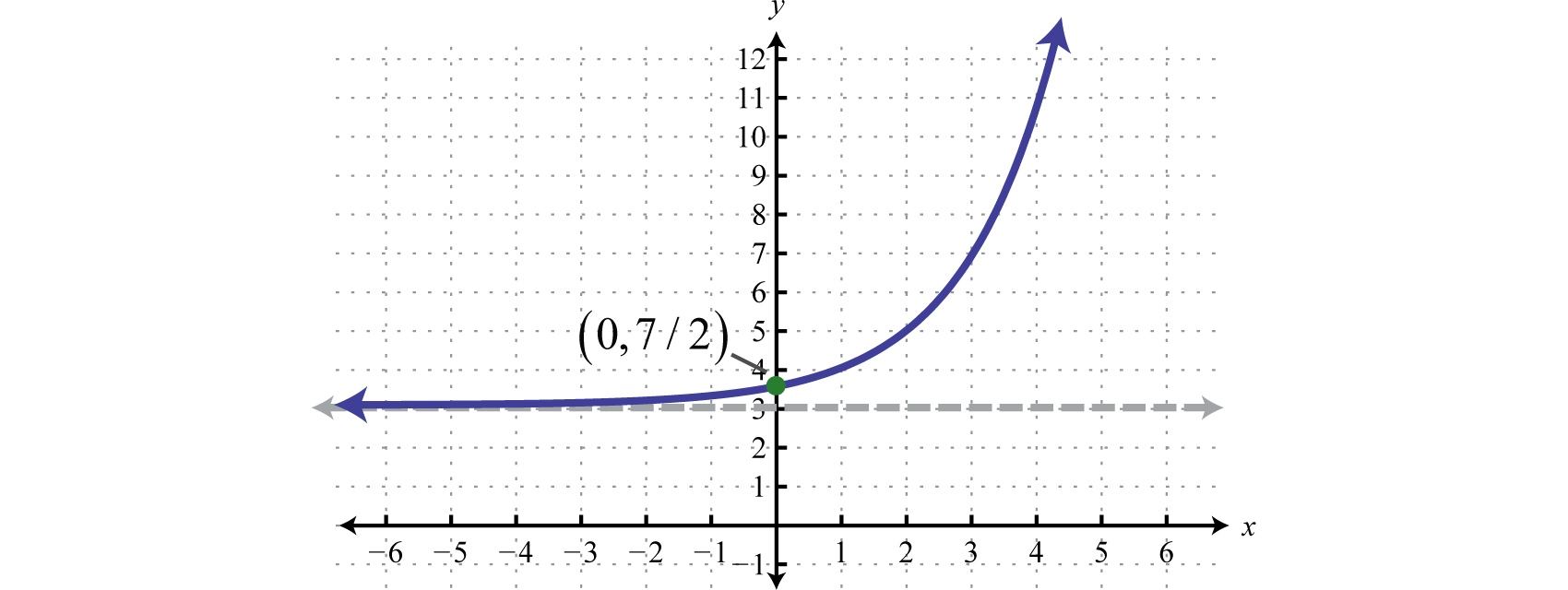Domain: $(−∞,∞)$; Range: $(3,∞)$

## Natural Base e

Some numbers occur often in common applications. One such familiar number is pi (π), which we know occurs when working with circles. This irrational number has a dedicated button on most calculators $π$ and approximated to five decimal places, $π≈3.14159.$ Another important number e occurs when working with exponential growth and decay models. It is an irrational number and approximated to five decimal places, $e≈2.71828.$ This constant occurs naturally in many real-world applications and thus is called the natural base. Sometimes e is called Euler’s constant in honor of Leonhard Euler (pronounced “Oiler”).

Figure 7.1Leonhard Euler (1707–1783)

In fact, the natural exponential function, $f(x)=ex$ is so important that you will find a button $ex$ dedicated to it on any modern scientific calculator. In this section, we are interested in evaluating the natural exponential function for given real numbers and sketching its graph. To evaluate the natural exponential function, defined by $f(x)=ex$ where $x=−2$ using a calculator, you may need to apply the shift button. On many scientific calculators the caret will display as follows,

$f(−2)=e^(−2)≈0.13534$

After learning how to use your particular calculator, you can now sketch the graph by plotting points. (Round off to the nearest hundredth.)

$xyf(x)=exSolutions−20.14f(−2)=e−2=0.14(−2, 0.14)−10.37f(−1)=e−1=0.37(−1, 0.37)01f(0)=e0=1(0, 1)12.72f(1)=e1=2.72(1, 2.72)27.39f(2)=e2=7.39(2, 7.39)$

Plot the points and sketch the graph.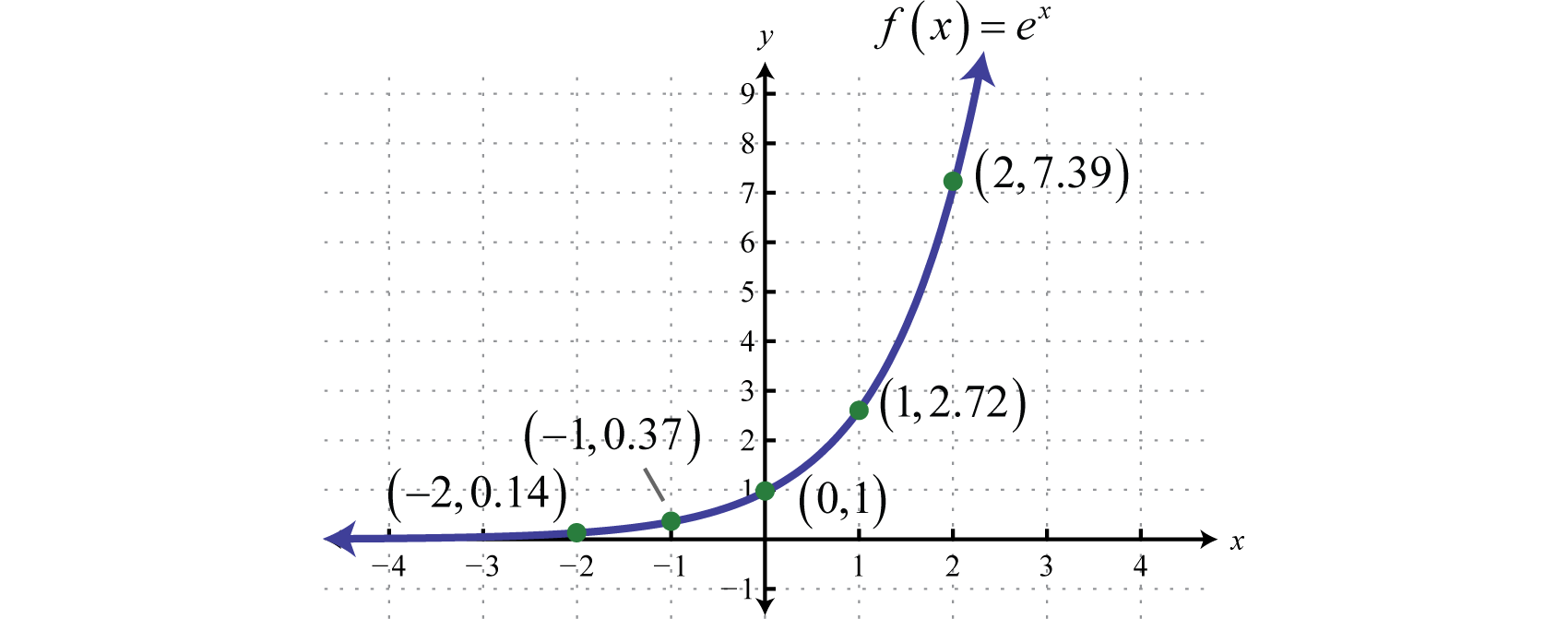Note that the function is similar to the graph of $y=3x.$ The domain consists of all real numbers and the range consists of all positive real numbers. There is an asymptote at $y=0$ and a y-intercept at $(0,1).$ We can use the transformations to sketch the graph of more complicated exponential functions.

### Example 4

Sketch the graph and determine the domain and range: $g(x)=ex+2−3.$

Solution:

Identify the basic transformations.

$y=ex Basic graphy=ex+2 Shift left 2 unitsy=ex+2−3 Shift down 3 units$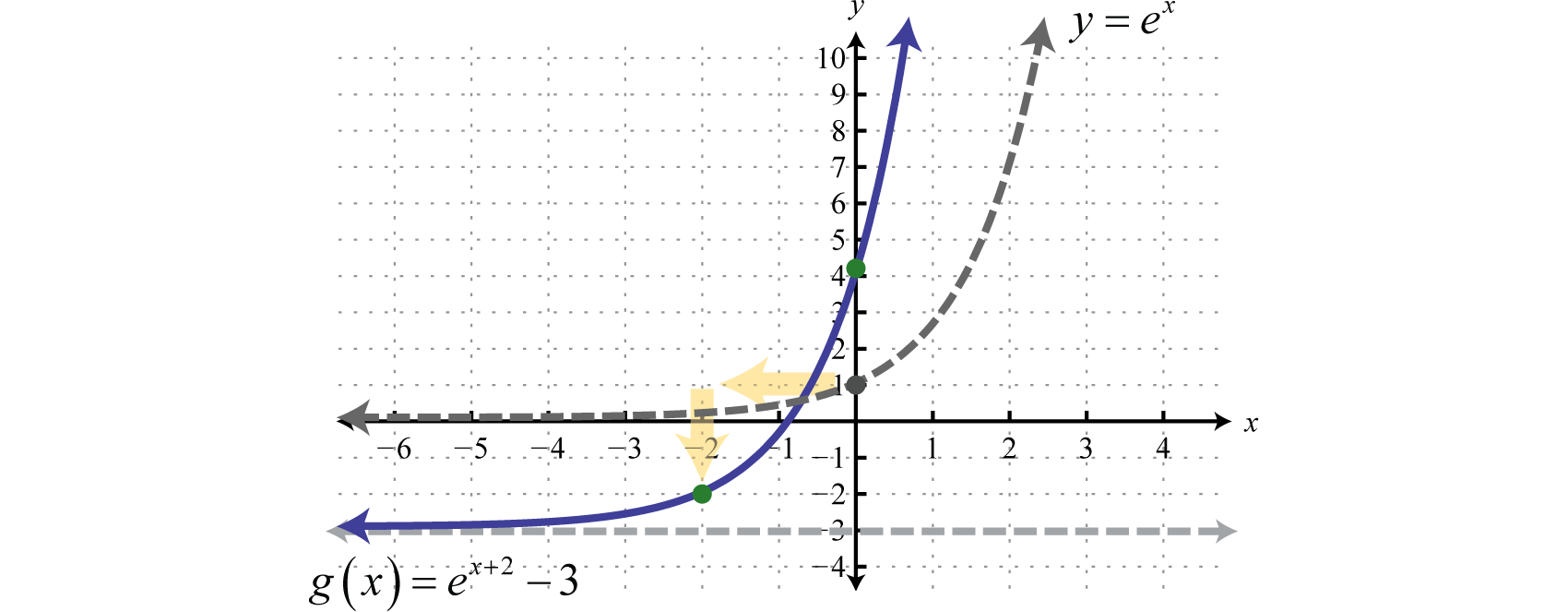To determine the y-intercept set $x=0.$

$g(0)=e0+2−3=e2−3≈4.39$

Therefore the y-intercept is $(0,e2−3).$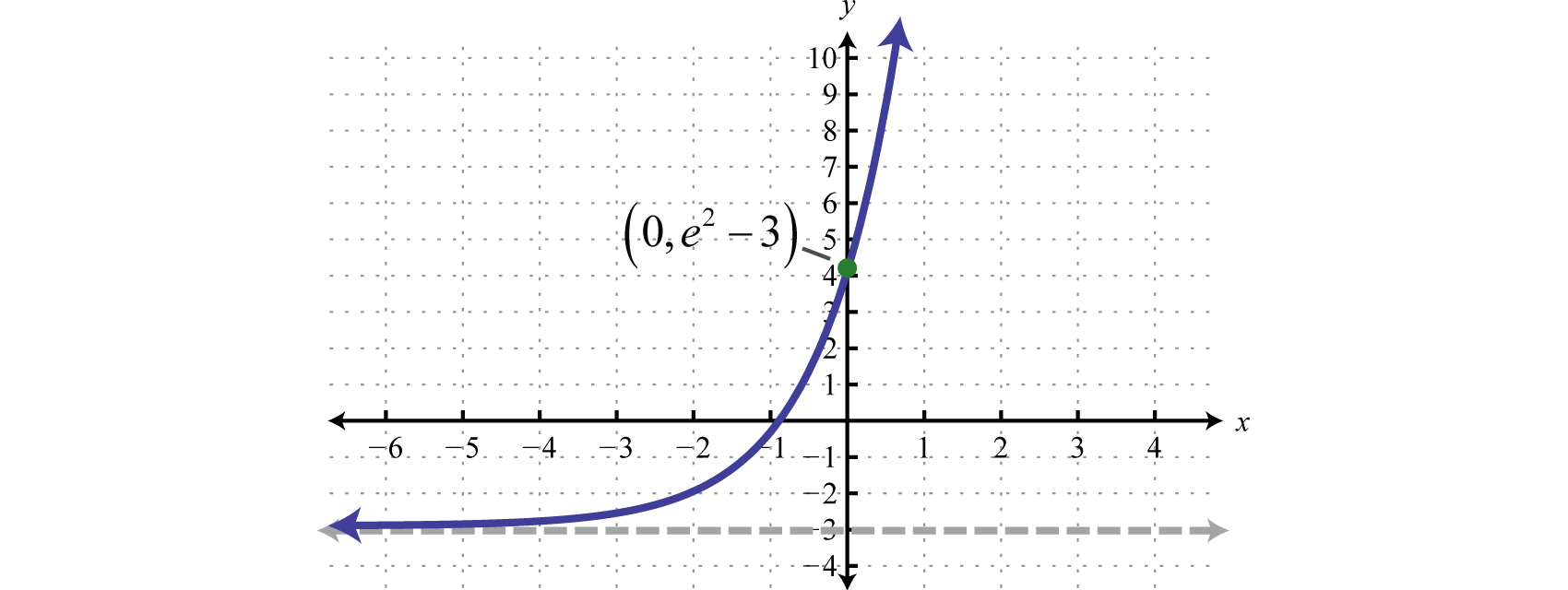Domain: $(−∞,∞)$; Range: $(−3,∞)$

Try this! Sketch the graph and determine the domain and range: $f(x)=e−x+2.$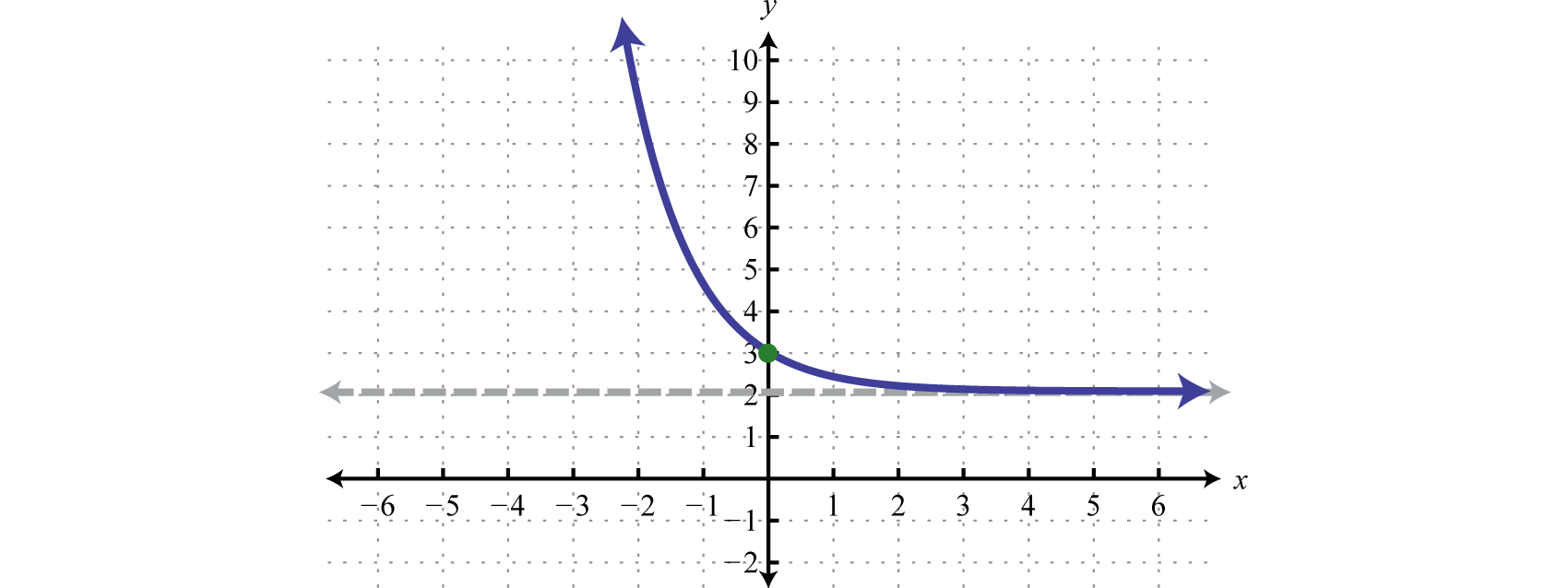Domain: $(−∞,∞)$; Range: $(2,∞)$

## Compound Interest Formulas

Exponential functions appear in formulas used to calculate interest earned in most regular savings accounts. Compound interest occurs when interest accumulated for one period is added to the principal investment before calculating interest for the next period. The amount accrued in this manner over time is modeled by the compound interest formulaA formula that gives the amount accumulated by earning interest on principal and interest over time: $A(t)=P(1+rn)nt.$:

$A(t)=P(1+rn)nt$

Here the amount A depends on the time t in years the principal P is accumulating compound interest at an annual interest rate r. The value n represents the number of times the interest is compounded in a year.

### Example 5

An investment of \$500 is made in a 6-year CD that earns $412$% annual interest that is compounded monthly. How much will the CD be worth at the end of the 6-year term?

Solution:

Here the principal $P=500$, the interest rate $r=412%=0.045$, and because the interest is compounded monthly, $n=12.$ The investment is modeled by the following,

$A(t)=500(1+0.04512)12t$

To determine the amount in the account after 6 years evaluate $A(6)$ and round off to the nearest cent.

$A(6)=500(1+0.04512)12(6)=500(1.00375)72=654.65$

Answer: The CD will be worth \$654.65 at the end of the 6-year term.

Next we explore the effects of increasing n in the formula. For the sake of clarity we let P and r equal 1 and calculate accordingly.

Annual compounding

$(1+1n)n$

Yearly ($n=1$)

$(1+11)1=2$

Semi-annually ($n=2$)

$(1+12)2=2.25$

Quarterly ($n=4$)

$(1+14)4≈2.44140$

Monthly ($n=12$)

$(1+112)12≈2.61304$

Weekly ($n=52$)

$(1+152)52≈2.69260$

Daily ($n=365$)

$(1+1365)365≈2.71457$

Hourly ($n=8760$)

$(1+18760)8760≈2.71813$

Continuing this pattern, as n increases to say compounding every minute or even every second, we can see that the result tends toward the natural base $e≈2.71828.$ Compounding interest every instant leads to the continuously compounding interest formulaA formula that gives the amount accumulated by earning continuously compounded interest: $A(t)=Pert.$,

$A(t)=Pert$

Here P represents the initial principal amount invested, r represents the annual interest rate, and t represents the time in years the investment is allowed to accrue continuously compounded interest.

### Example 6

An investment of \$500 is made in a 6-year CD that earns $412$% annual interest that is compounded continuously. How much will the CD be worth at the end of the 6-year term?

Solution:

Here the principal $P=500$, and the interest rate $r=412%=0.045.$ Since the interest is compounded continuously we will use the formula $A(t)=Pert.$ The investment is modeled by the following,

$A(t)=500e0.045t$

To determine the amount in the account after 6 years, evaluate $A(6)$ and round off to the nearest cent.

$A(6)=500e0.045(6)=500e0.27=654.98$

Answer: The CD will be worth \$654.98 at the end of the 6-year term.

Compare the previous two examples and note that compounding continuously may not be as beneficial as it sounds. While it is better to compound interest more often, the difference is not that profound. Certainly, the interest rate is a much greater factor in the end result.

Try this! How much will a \$1,200 CD, earning 5.2% annual interest compounded continuously, be worth at the end of a 10-year term?

### Key Takeaways

• Exponential functions have definitions of the form $f(x)=bx$ where $b>0$ and $b≠1.$ The domain consists of all real numbers $(−∞,∞)$ and the range consists of positive numbers $(0,∞).$ Also, all exponential functions of this form have a y-intercept of $(0,1)$ and are asymptotic to the x-axis.
• If the base of an exponential function is greater than 1 ($b>1$), then its graph increases or grows as it is read from left to right.
• If the base of an exponential function is a proper fraction ($0), then its graph decreases or decays as it is read from left to right.
• The number 10 is called the common base and the number e is called the natural base.
• The natural exponential function defined by $f(x)=ex$ has a graph that is very similar to the graph of $g(x)=3x.$
• Exponential functions are one-to-one.

### Part A: Exponential Functions

Evaluate.

1. $f(x)=3x$ where $f(−2)$, $f(0)$, and $f(2).$

2. $f(x)=10x$ where $f(−1)$, $f(0)$, and $f(1).$

3. $g(x)=(13)x$ where $g(−1)$, $g(0)$, and $g(3).$

4. $g(x)=(34)x$ where $g(−2)$, $g(−1)$, and $g(0).$

5. $h(x)=9−x$ where $h(−1)$, $h(0)$, and $h(12).$

6. $h(x)=4−x$ where $h(−1)$, $h(−12)$, and $h(0).$

7. $f(x)=−2x+1$ where $f(−1)$, $f(0)$, and $f(3).$

8. $f(x)=2−3x$ where $f(−1)$, $f(0)$, and $f(2).$

9. $g(x)=10−x+20$ where $g(−2)$, $g(−1)$, and $g(0).$

10. $g(x)=1−2−x$ where $g(−1)$, $g(0)$, and $g(1).$

Use a calculator to approximate the following to the nearest hundredth.

1. $f(x)=2x+5$ where $f(2.5).$

2. $f(x)=3x−10$ where $f(3.2).$

3. $g(x)=4x$ where $g(2).$

4. $g(x)=5x−1$ where $g(3).$

5. $h(x)=10x$ where $h(π).$

6. $h(x)=10x+1$ where $h(π3)$

7. $f(x)=10−x−2$ where $f(1.5).$

8. $f(x)=5−x+3$ where $f(1.3).$

9. $f(x)=(23)x+1$ where $f(−2.7).$

10. $f(x)=(35)−x−1$ where $f(1.4).$

Sketch the function and determine the domain and range. Draw the horizontal asymptote with a dashed line.

1. $f(x)=4x$

2. $g(x)=3x$

3. $f(x)=4x+2$

4. $f(x)=3x−6$

5. $f(x)=2x−2$

6. $f(x)=4x+2$

7. $f(x)=3x+1−4$

8. $f(x)=10x−4+2$

9. $h(x)=2x−3−2$

10. $h(x)=3x+2+4$

11. $f(x)=(14)x$
12. $h(x)=(13)x$
13. $f(x)=(14)x−2$
14. $h(x)=(13)x+2$
15. $g(x)=2−x−3$

16. $g(x)=3−x+1$

17. $f(x)=6−10−x$

18. $g(x)=5−4−x$

19. $f(x)=5−2x$

20. $f(x)=3−3x$

### Part B: Natural Base e

Find $f(−1)$, $f(0)$, and $f(32)$ for the given function. Use a calculator where appropriate to approximate to the nearest hundredth.

1. $f(x)=ex+2$

2. $f(x)=ex−4$

3. $f(x)=5−3ex$

4. $f(x)=e−x+3$

5. $f(x)=1+e−x$

6. $f(x)=3−2e−x$

7. $f(x)=e−2x+2$

8. $f(x)=e−x2−1$

Sketch the function and determine the domain and range. Draw the horizontal asymptote with a dashed line.

1. $f(x)=ex−3$

2. $f(x)=ex+2$

3. $f(x)=ex+1$

4. $f(x)=ex−3$

5. $f(x)=ex−2+1$

6. $f(x)=ex+2−1$

7. $g(x)=−ex$

8. $g(x)=e−x$

9. $h(x)=−ex+1$

10. $h(x)=−ex+3$

### Part C: Compound Interest Formulas

1. Jim invested \$750 in a 3-year CD that earns 4.2% annual interest that is compounded monthly. How much will the CD be worth at the end of the 3-year term?

2. Jose invested \$2,450 in a 4-year CD that earns 3.6% annual interest that is compounded semi-annually. How much will the CD be worth at the end of the 4-year term?

3. Jane has her \$5,350 savings in an account earning $358$% annual interest that is compounded quarterly. How much will be in the account at the end of 5 years?

4. Bill has \$12,400 in a regular savings account earning $423$% annual interest that is compounded monthly. How much will be in the account at the end of 3 years?

5. If \$85,200 is invested in an account earning 5.8% annual interest compounded quarterly, then how much interest is accrued in the first 3 years?

6. If \$124,000 is invested in an account earning 4.6% annual interest compounded monthly, then how much interest is accrued in the first 2 years?

7. Bill invested \$1,400 in a 3-year CD that earns 4.2% annual interest that is compounded continuously. How much will the CD be worth at the end of the 3-year term?

8. Brooklyn invested \$2,850 in a 5-year CD that earns 5.3% annual interest that is compounded continuously. How much will the CD be worth at the end of the 5-year term?

9. Omar has his \$4,200 savings in an account earning $438$% annual interest that is compounded continuously. How much will be in the account at the end of $212$ years?

10. Nancy has her \$8,325 savings in an account earning $578$% annual interest that is compounded continuously. How much will be in the account at the end of $512$ years?

11. If \$12,500 is invested in an account earning 3.8% annual interest compounded continuously, then how much interest is accrued in the first 10 years?

12. If \$220,000 is invested in an account earning 4.5% annual interest compounded continuously, then how much interest is accrued in the first 2 years?

13. The population of a certain small town is growing according to the function $P(t)=12,500(1.02)t$ where t represents time in years since the last census. Use the function to determine the population on the day of the census (when t = 0) and estimate the population in 6 years from that time.

14. The population of a certain small town is decreasing according to the function $P(t)=22,300(0.982)t$ where t represents time in years since the last census. Use the function to determine the population on the day of the census (when t = 0) and estimate the population in 6 years from that time.

15. The decreasing value, in dollars, of a new car is modeled by the formula $V(t)=28,000(0.84)t$ where t represents the number of years after the car was purchased. Use the formula to determine the value of the car when it was new (t = 0) and the value after 4 years.

16. The number of unique visitors to the college website can be approximated by the formula $N(t)=410(1.32)t$ where t represents the number of years after 1997 when the website was created. Approximate the number of unique visitors to the college website in the year 2020.

17. If left unchecked, a new strain of flu virus can spread from a single person to others very quickly. The number of people affected can be modeled using the formula $P(t)=e0.22t$ where t represents the number of days the virus is allowed to spread unchecked. Estimate the number of people infected with the virus after 30 days and after 60 days.

18. If left unchecked, a population of 24 wild English rabbits can grow according to the formula $P(t)=24e0.19t$ where the time t is measured in months. How many rabbits would be present $312$ years later?

19. The population of a certain city in 1975 was 65,000 people and was growing exponentially at an annual rate of 1.7%. At the time, the population growth was modeled by the formula $P(t)=65,000e0.017t$ where t represented the number of years since 1975. In the year 2000, the census determined that the actual population was 104,250 people. What population did the model predict for the year 2000 and what was the actual error?

20. Because of radioactive decay, the amount of a 10 milligram sample of Iodine-131 decreases according to the formula $A(t)=10e−0.087t$ where t represents time measured in days. How much of the sample remains after 10 days?

21. The number of cells in a bacteria sample is approximated by the logistic growth model $N(t)=1.2×1051+9e−0.32t$ where t represents time in hours. Determine the initial number of cells and then determine the number of cells 6 hours later.

22. The market share of a product, as a percentage, is approximated by the formula $P(t)=1002+e−0.44t$ where t represents the number of months after an aggressive advertising campaign is launched. By how much can we expect the market share to increase after the first three months of advertising?

### Part D: Discussion Board

1. Why is b = 1 excluded as a base in the definition of exponential functions? Explain.

2. Explain why an exponential function of the form $y=bx$ can never be negative.

3. Research and discuss the derivation of the compound interest formula.

4. Research and discuss the logistic growth model. Provide a link to more information on this topic.

5. Research and discuss the life and contributions of Leonhard Euler.

1. $f(−2)=19$, $f(0)=1$, $f(2)=9$

2. $g(−1)=3$, $g(0)=1$, $g(3)=127$

3. $h(−1)=9$, $h(0)=1$, $h(12)=13$

4. $f(−1)=12$, $f(0)=0$, $f(3)=−7$

5. $g(−2)=120$, $g(−1)=30$, $g(0)=21$

6. 10.66

7. 7.10

8. 1385.46

9. −1.97

10. 3.99

11.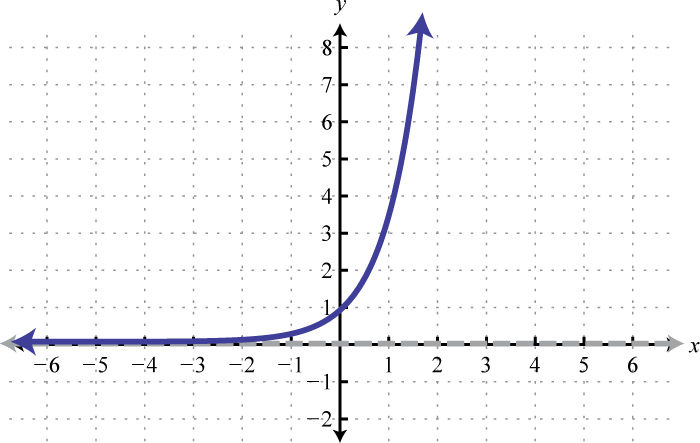Domain: $(−∞,∞)$; Range: $(0,∞)$

12.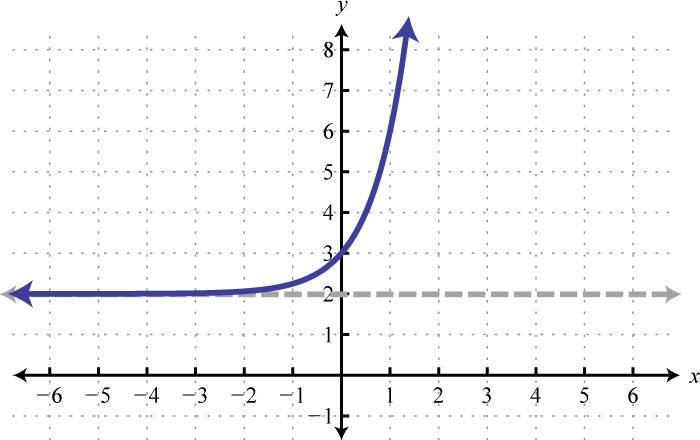Domain: $(−∞,∞)$; Range: $(2,∞)$

13.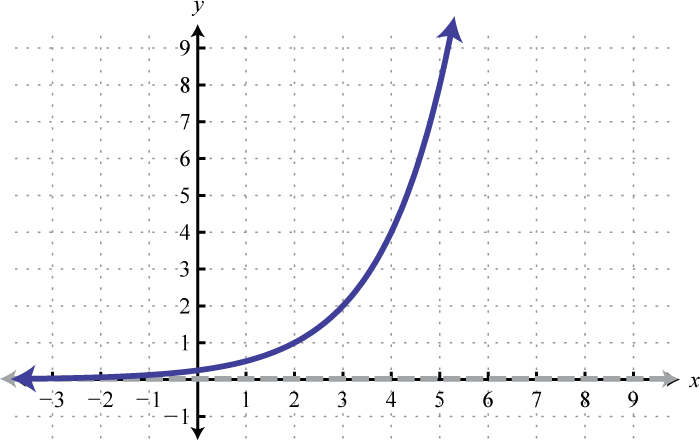Domain: $(−∞,∞)$; Range: $(0,∞)$

14.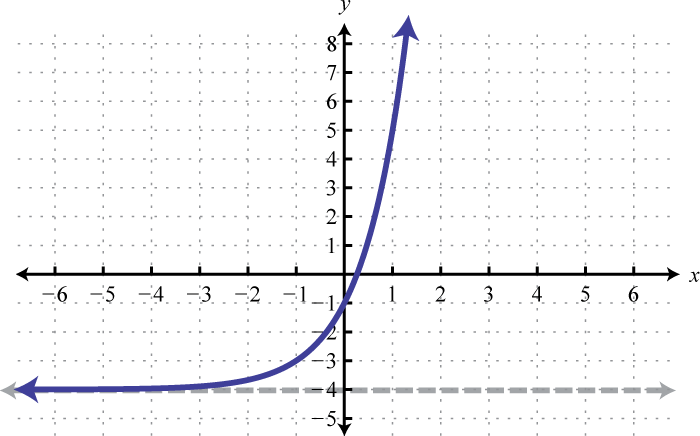Domain: $(−∞,∞)$; Range: $(−4,∞)$

15.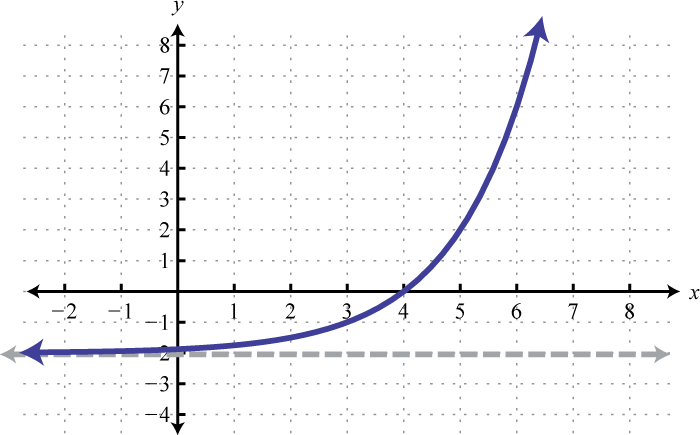Domain: $(−∞,∞)$; Range: $(−2,∞)$

16.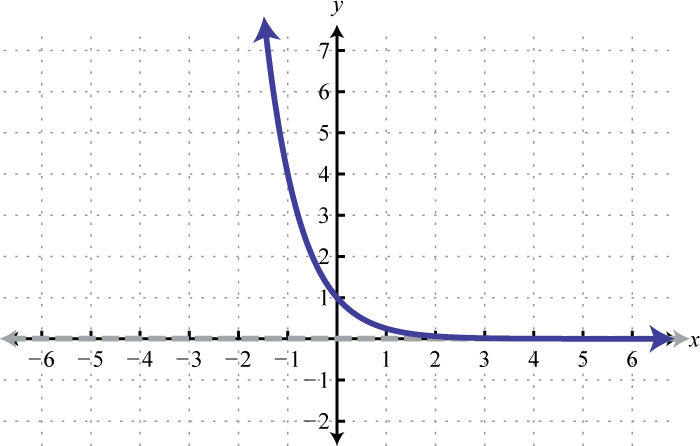Domain: $(−∞,∞)$; Range: $(0,∞)$

17.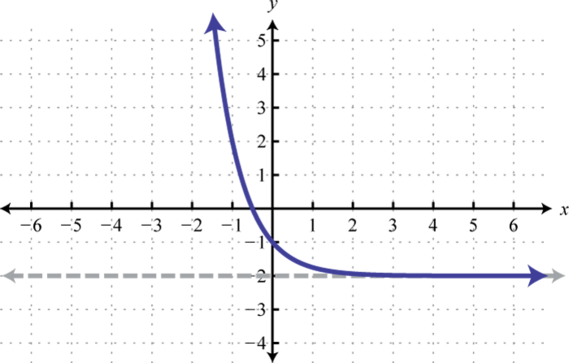Domain: $(−∞,∞)$; Range: $(−2,∞)$

18.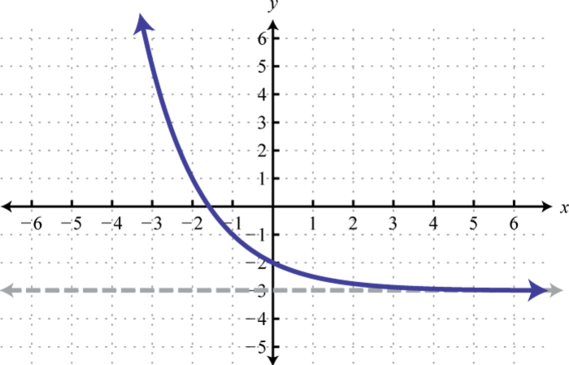Domain: $(−∞,∞)$; Range: $(−3,∞)$

19.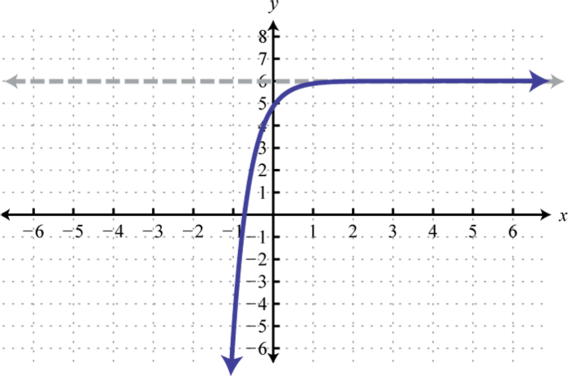Domain: $(−∞,∞)$; Range: $(−∞,6)$

20.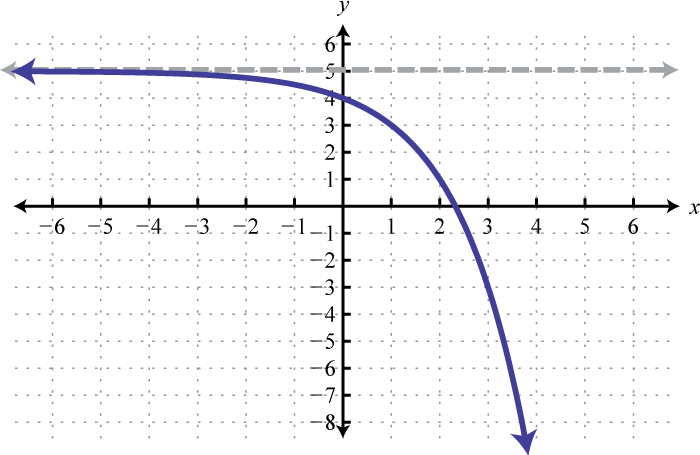Domain: $(−∞,∞)$; Range: $(−∞,5)$

1. $f(−1)≈2.37$, $f(0)=3$, $f(32)≈6.48$

2. $f(−1)≈3.90$, $f(0)=2$, $f(32)≈−8.45$

3. $f(−1)≈3.72$, $f(0)=2$, $f(32)≈1.22$

4. $f(−1)≈9.39$, $f(0)=3$, $f(32)≈2.05$

5.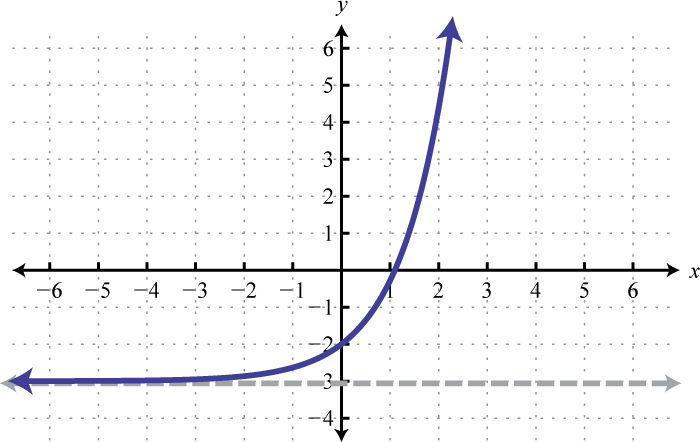Domain: $(−∞,∞)$; Range: $(−3,∞)$

6.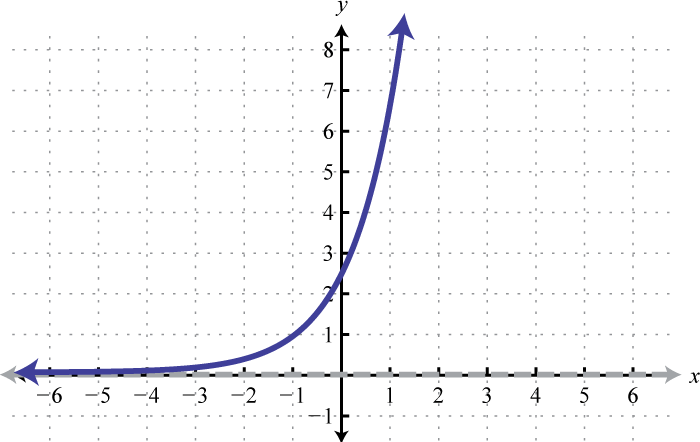Domain: $(−∞,∞)$; Range: $(0,∞)$

7.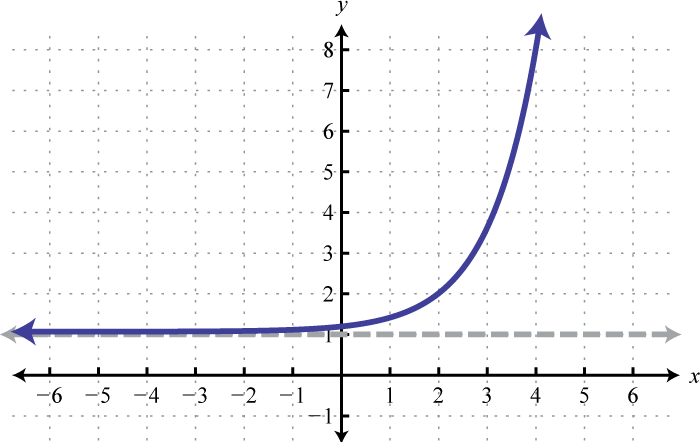Domain: $(−∞,∞)$; Range: $(1,∞)$

8.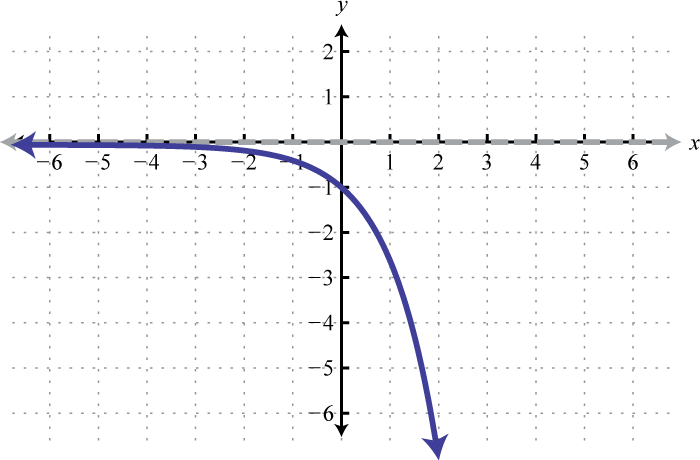Domain: $(−∞,∞)$; Range: $(−∞,0)$

9.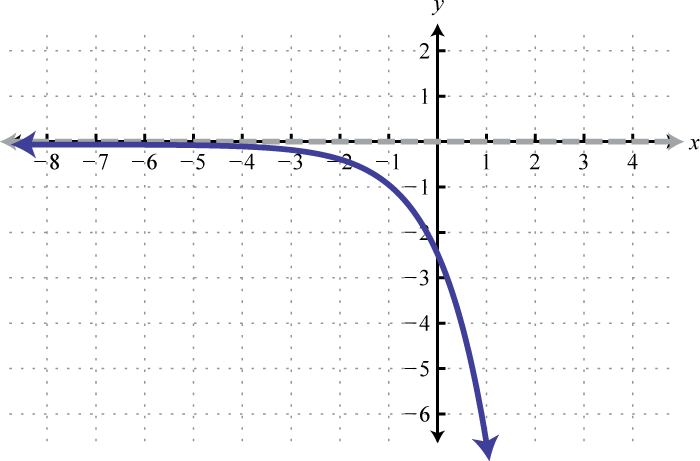Domain: $(−∞,∞)$; Range: $(−∞,0)$

1. \$850.52

2. \$6,407.89

3. \$16,066.13

4. \$1,588.00

5. \$4,685.44

6. \$5,778.56

7. Initial population: 12,500; Population 6 years later: 14,077

8. New: \$28,000; In 4 years: \$13,940.40

9. After 30 days: 735 people; After 60 days: 540,365 people

10. Model: 99,423 people; error: 4,827 people

11. Initially there are 12,000 cells and 6 hours later there are 51,736 cells.

## 7.3 Logarithmic Functions and Their Graphs

### Learning Objectives

1. Define and evaluate logarithms.
2. Identify the common and natural logarithm.
3. Sketch the graph of logarithmic functions.

## Definition of the Logarithm

We begin with the exponential function defined by $f(x)=2x$ and note that it passes the horizontal line test.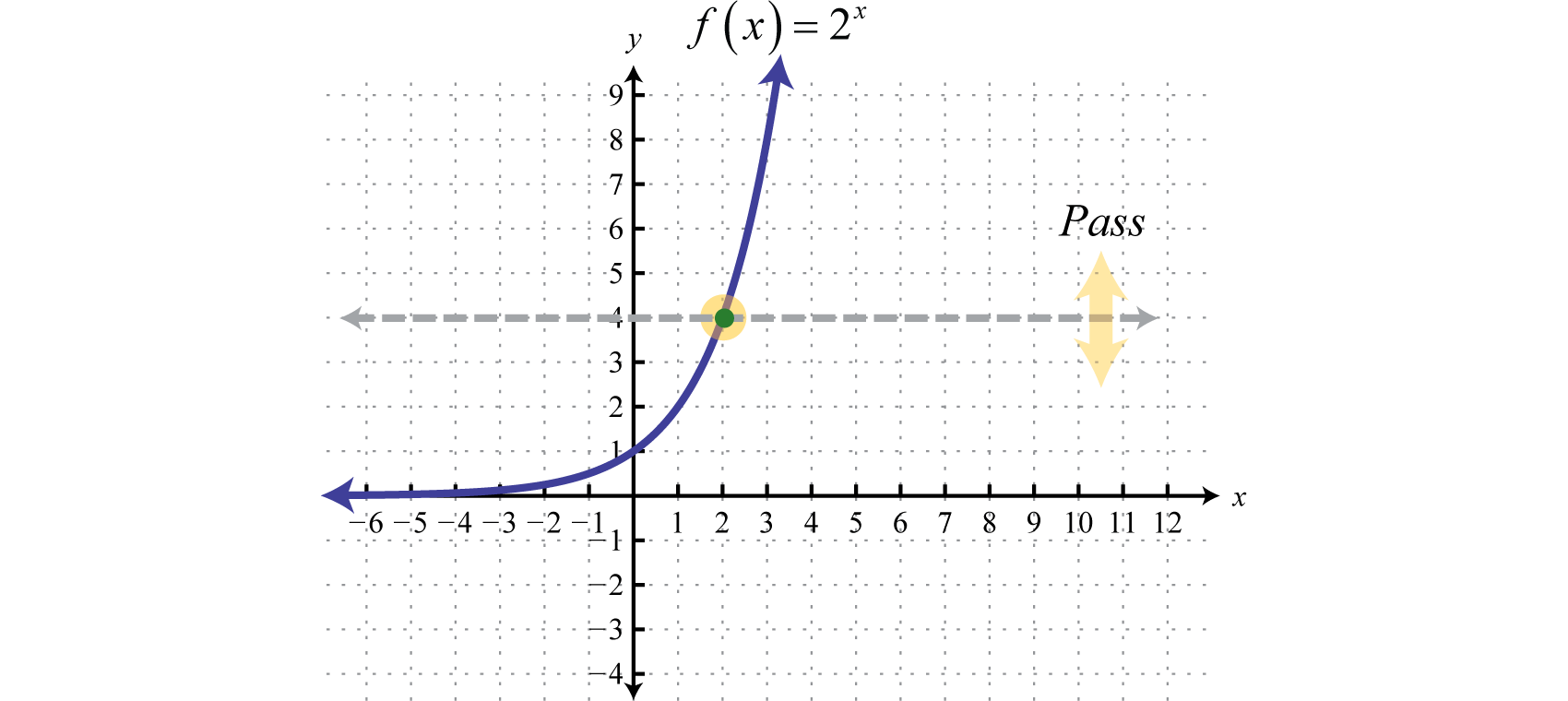Therefore it is one-to-one and has an inverse. Reflecting $y=2x$ about the line $y=x$ we can sketch the graph of its inverse. Recall that if $(x,y)$ is a point on the graph of a function, then $(y,x)$ will be a point on the graph of its inverse.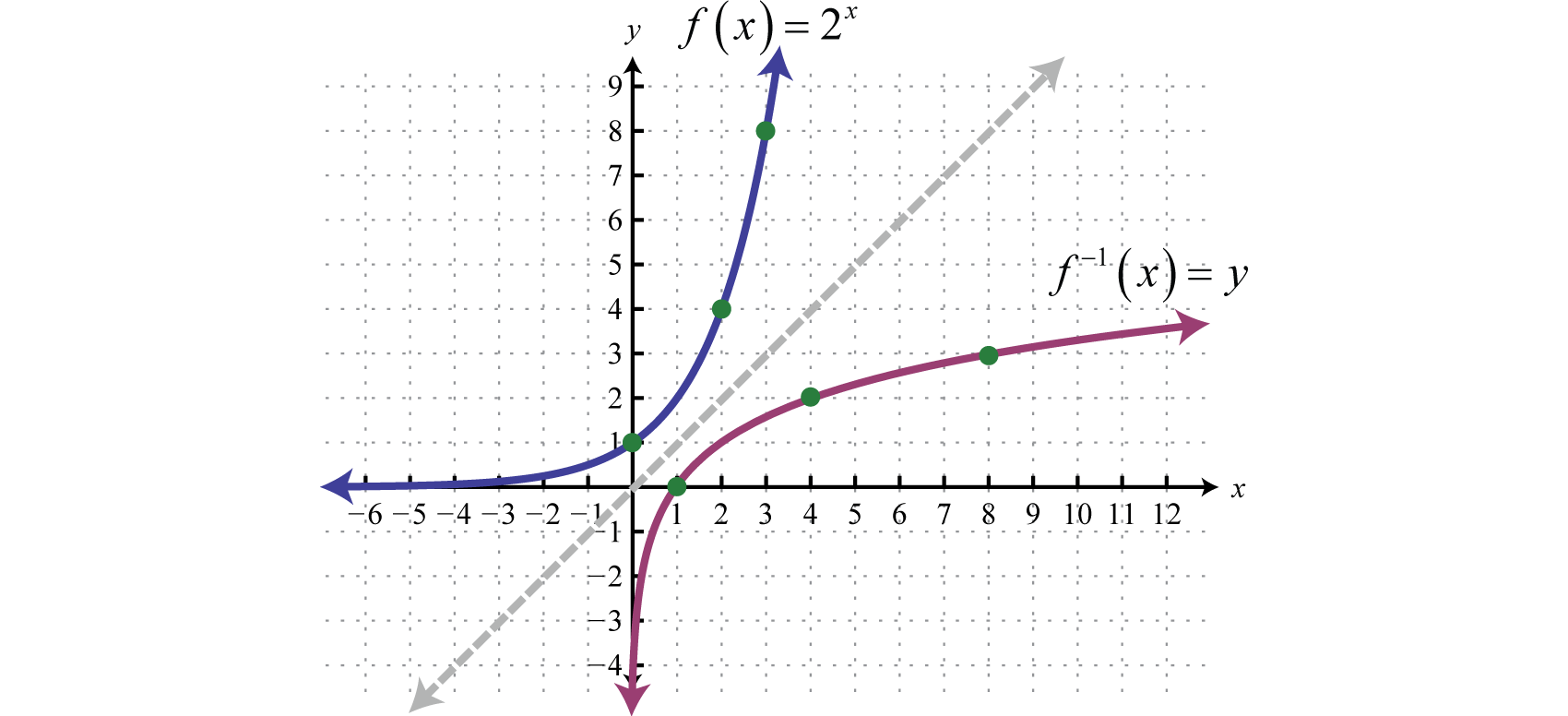To find the inverse algebraically, begin by interchanging x and y and then try to solve for y.

$f(x)=2xy=2x ⇒ x=2y$

We quickly realize that there is no method for solving for y. This function seems to “transcend” algebra. Therefore, we define the inverse to be the base-2 logarithm, denoted $log2 x.$ The following are equivalent:

$y=log2 x ⇔ x=2y$

This gives us another transcendental function defined by $f−1(x)=log2 x$, which is the inverse of the exponential function defined by $f(x)=2x.$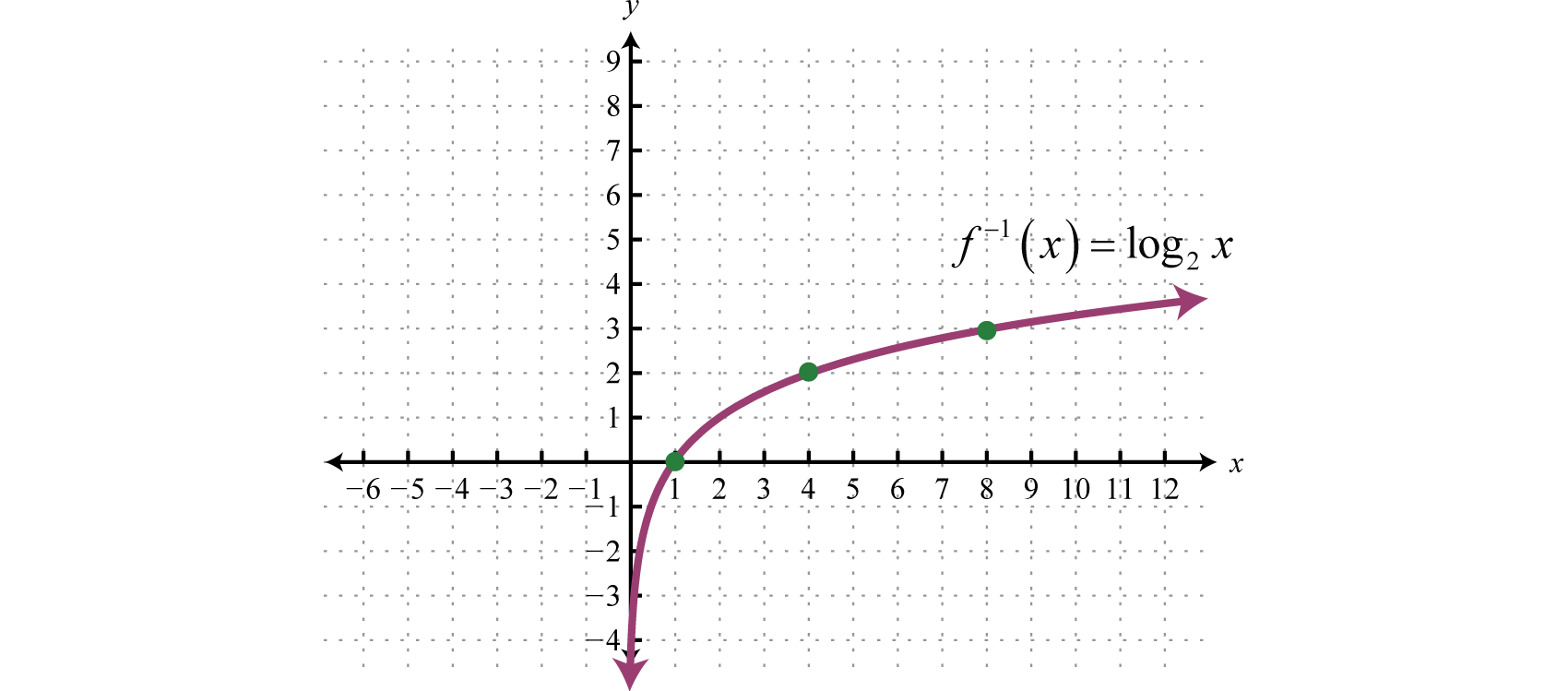The domain consists of all positive real numbers $(0,∞)$ and the range consists of all real numbers $(−∞,∞).$ John Napier is widely credited for inventing the term logarithm.

Figure 7.2John Napier (1550–1617)

In general, given base $b>0$ where $b≠1$, the logarithm base bThe exponent to which the base b is raised in order to obtain a specific value. In other words, $y=logb x$ is equivalent to $by=x.$ is defined as follows:

$y=logb x if and only if x=by$

Use this definition to convert logarithms to exponential form and back.

Logarithmic Form

Exponential Form

$log2 16=4$

$24=16$

$log5 25=2$

$52=25$

$log6 1=0$

$60=1$

$log3 3=12$

$31/2=3$

$log7 (149)=−2$

$7−2=149$

It is useful to note that the logarithm is actually the exponent y to which the base b is raised to obtain the argument x.### Example 1

Evaluate:

1. $log5 125$
2. $log2 (18)$
3. $log4 2$
4. $log11 1$

Solution:

1. $log5 125=3$ because $53=125.$
2. $log2 (18)=−3$ because $2−3=123=18.$
3. $log4 2=12$ because $41/2=4=2.$
4. $log11 1=0$ because $110=1.$

Note that the result of a logarithm can be negative or even zero. However, the argument of a logarithm is not defined for negative numbers or zero:

$log2 (−4)=? ⇒ 2?=−4log2 (0)=? ⇒ 2?=0$

There is no power of two that results in −4 or 0. Negative numbers and zero are not in the domain of the logarithm. At this point it may be useful to go back and review all of the rules of exponents.

### Example 2

Find x:

1. $log7 x=2$
2. $log16 x=12$
3. $log1/2 x=−5$

Solution:

Convert each to exponential form and then simplify using the rules of exponents.

1. $log7 x=2$ is equivalent to $72=x$ and thus $x=49.$
2. $log16 x=12$ is equivalent to $161/2=x$ or $16=x$ and thus $x=4.$
3. $log1/2 x=−5$ is equivalent to $(12)−5=x$ or $25=x$ and thus $x=32$

Try this! Evaluate: $log5 (153)$.

Answer: $−13$

## The Common and Natural Logarithm

A logarithm can have any positive real number, other than 1, as its base. If the base is 10, the logarithm is called the common logarithmThe logarithm base 10, denoted $log x.$.

$f(x)=log10 x=log x Common logarithm$

When a logarithm is written without a base it is assumed to be the common logarithm. (Note: This convention varies with respect to the subject in which it appears. For example, computer scientists often let $log x$ represent the logarithm base 2.)

### Example 3

Evaluate:

1. $log 105$
2. $log 10$
3. $log 0.01$

Solution:

1. $log 105=5$ because $105=105.$
2. $log 10=12$ because $101/2=10.$
3. $log 0.01=log (1100)=−2$ because $10−2=1102=1100=0.01.$

The result of a logarithm is not always apparent. For example, consider $log 75.$

$log 10=1log 75=?log 100=2$

We can see that the result of $log 75$ is somewhere between 1 and 2. On most scientific calculators there is a common logarithm button $LOG.$ Use it to find the $log 75$ as follows:

$LOG75=1.87506$

Therefore, rounded off to the nearest thousandth, $log 75≈1.875.$ As a check, we can use a calculator to verify that $10^1.875≈75.$

If the base of a logarithm is $e$, the logarithm is called the natural logarithmThe logarithm base e, denoted $ln x.$.

$f(x)=loge x=ln x Natural logarithm$

The natural logarithm is widely used and is often abbreviated $ln x.$

### Example 4

Evaluate:

1. $ln e$
2. $ln e23$
3. $ln (1e4)$

Solution:

1. $ln e=1$ because $ln e=loge e=1$ and $e1=e.$
2. $ln (e23)=23$ because $e2/3=e23.$
3. $ln (1e4)=−4$ because $e−4=1e4$

On a calculator you will find a button for the natural logarithm $LN.$

$LN 75 = 4.317488$

Therefore, rounded off to the nearest thousandth, $ln (75)≈4.317.$ As a check, we can use a calculator to verify that $e^4.317≈75.$

### Example 5

Find x. Round answers to the nearest thousandth.

1. $log x=3.2$
2. $ln x=−4$
3. $log x=−23$

Solution:

Convert each to exponential form and then use a calculator to approximate the answer.

1. $log x=3.2$ is equivalent to $103.2=x$ and thus $x≈1584.893.$
2. $ln x=−4$ is equivalent to $e−4=x$ and thus $x≈0.018.$
3. $log x=−23$ is equivalent to $10−2/3=x$ and thus $x≈0.215$

Try this! Evaluate: $ln (1e)$.

Answer: $−12$

## Graphing Logarithmic Functions

We can use the translations to graph logarithmic functions. When the base $b>1$, the graph of $f(x)=logb x$ has the following general shape: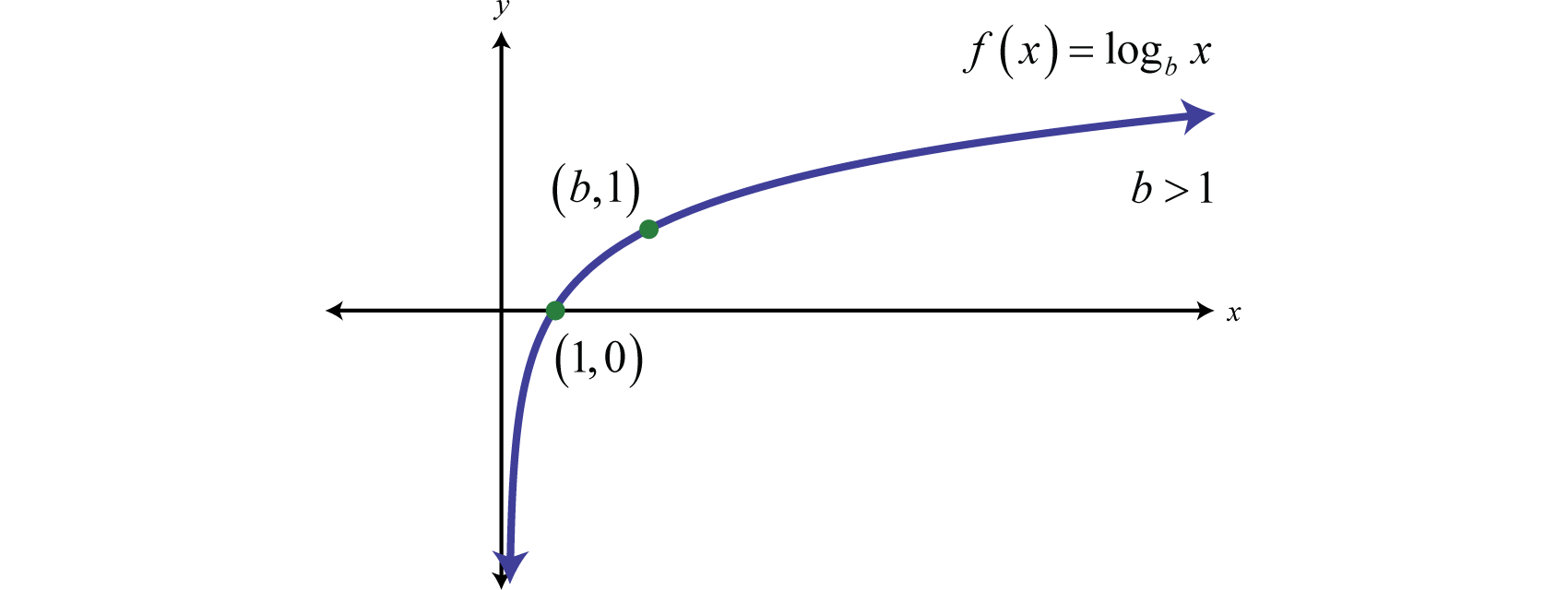The domain consists of positive real numbers, $(0,∞)$ and the range consists of all real numbers, $(−∞,∞).$ The y-axis, or $x=0$, is a vertical asymptote and the x-intercept is $(1,0).$ In addition, $f(b)=logb b=1$ and so $(b,1)$ is a point on the graph no matter what the base is.

### Example 6

Sketch the graph and determine the domain and range: $f(x)=log3 (x+4)−1.$

Solution:

Begin by identifying the basic graph and the transformations.

$y=log3 x Basic graphy=log3 (x+4) Shift left 4 units.y=log3 (x+4)−1 Shift down 1 unit.$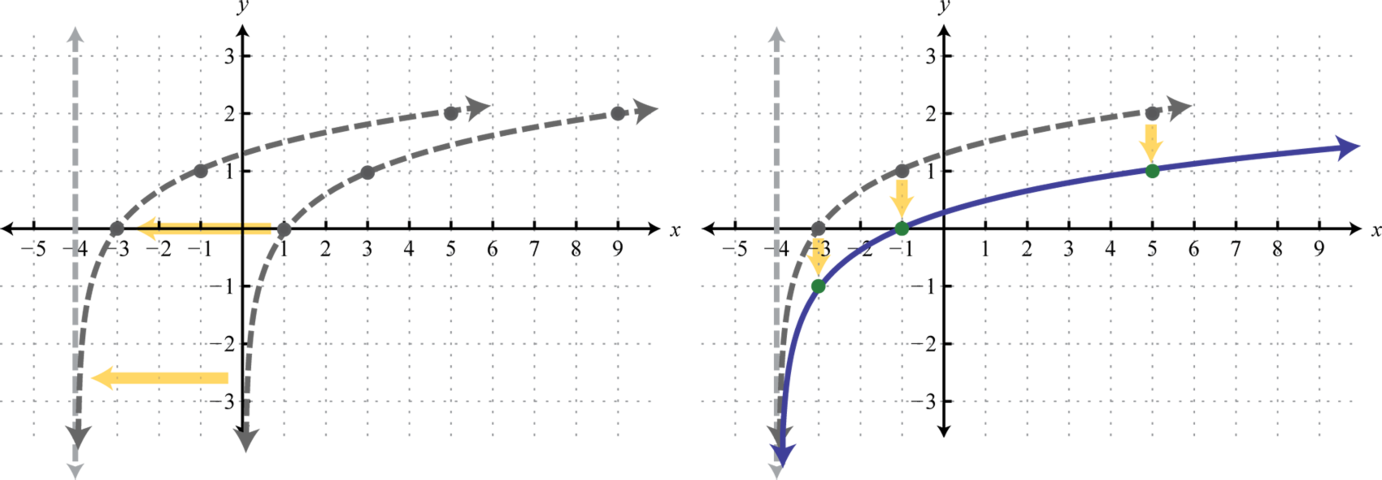Notice that the asymptote was shifted 4 units to the left as well. This defines the lower bound of the domain. The final graph is presented without the intermediate steps.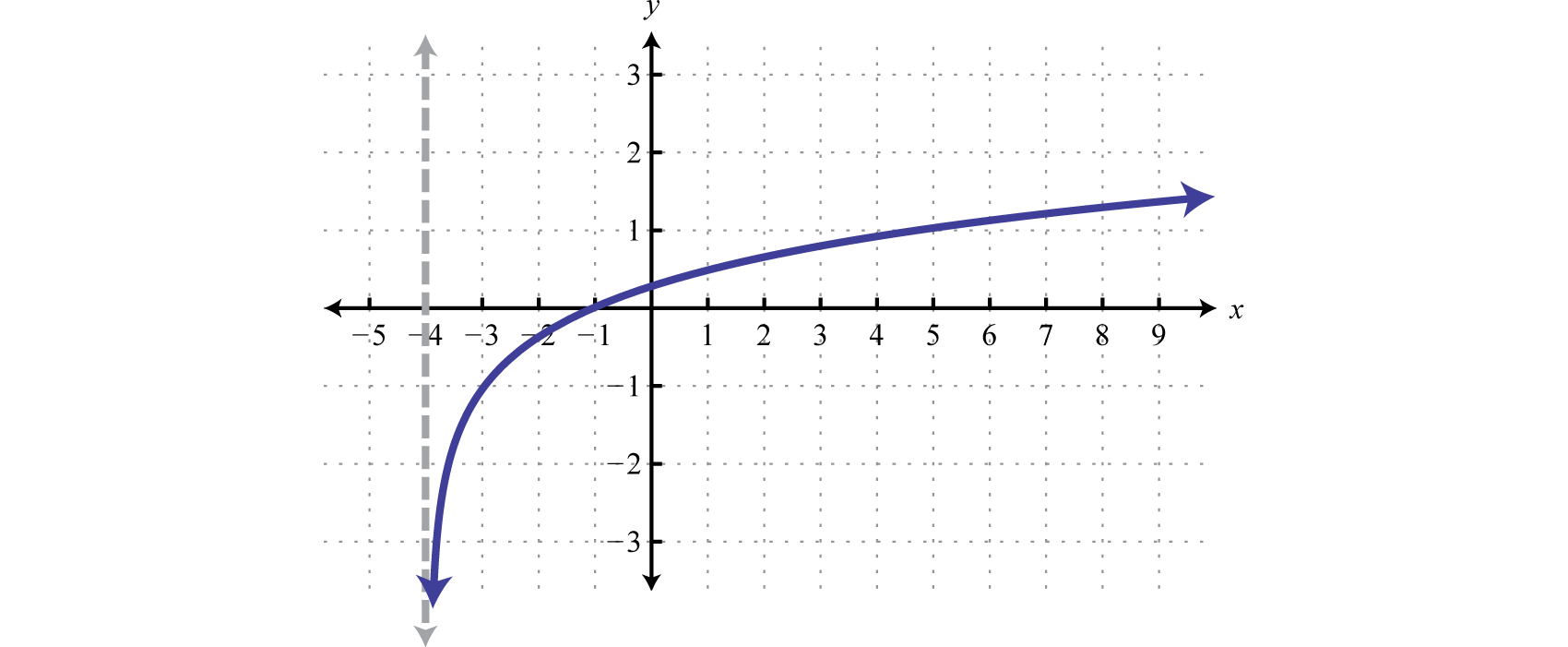Domain: $(−4,∞)$; Range: $(−∞,∞)$

Note: Finding the intercepts of the graph in the previous example is left for a later section in this chapter. For now, we are more concerned with the general shape of logarithmic functions.

### Example 7

Sketch the graph and determine the domain and range: $f(x)=−log (x−2).$

Solution:

Begin by identifying the basic graph and the transformations.

$y=log x Basic graphy=−log x Reflection about the x-axisy=−log (x−2) Shift right 2 units.$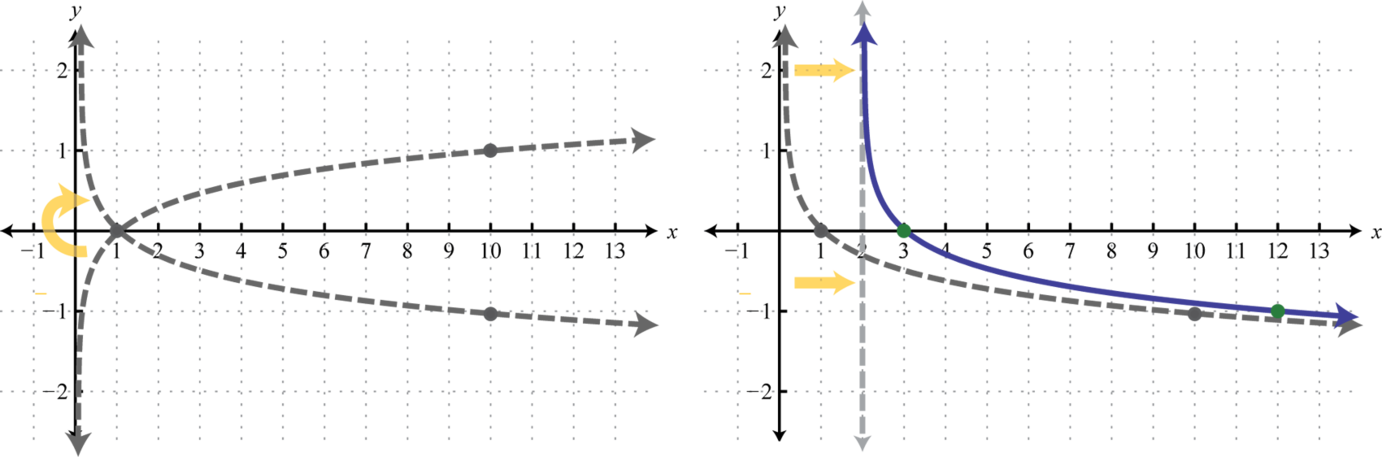Here the vertical asymptote was shifted two units to the right. This defines the lower bound of the domain.Domain: $(2,∞)$; Range: $(−∞,∞)$

Try this! Sketch the graph and determine the domain and range: $g(x)=ln (−x)+2.$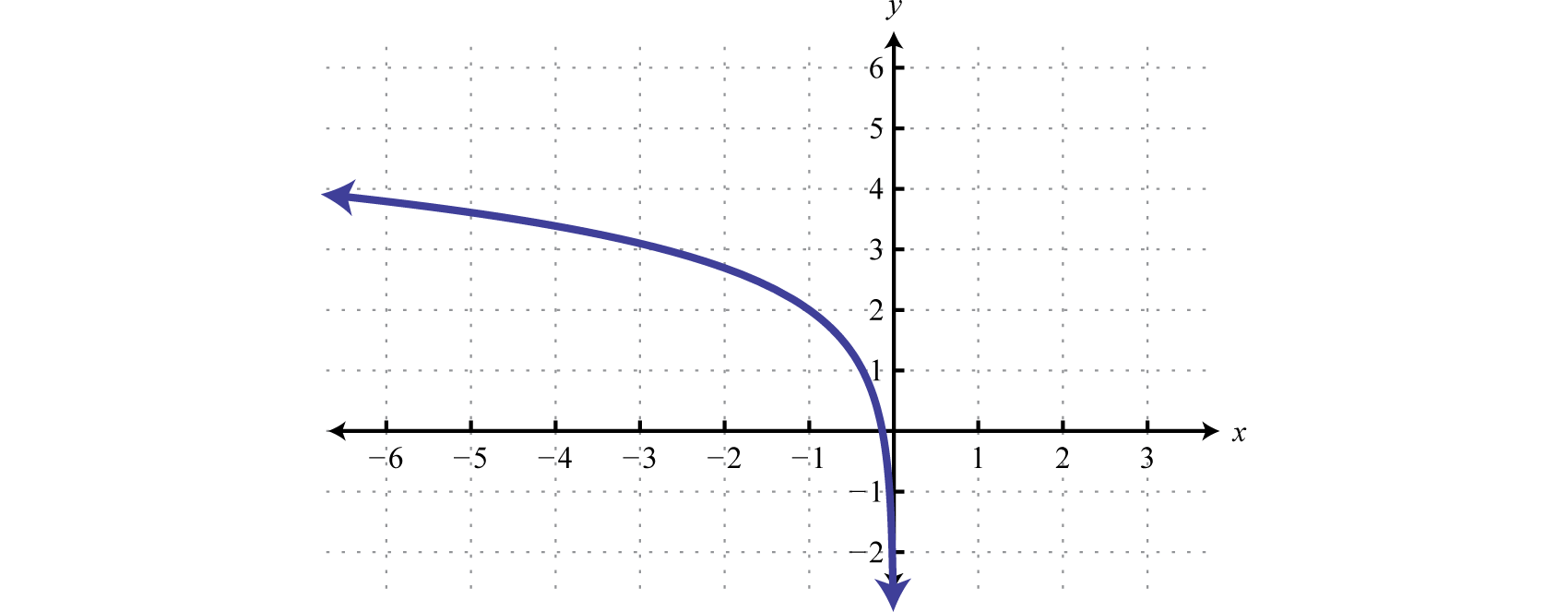Domain: (−∞, 0); Range: (−∞, ∞)

Next, consider exponential functions with fractional bases, such as the function defined by $f(x)=(12)x.$ The domain consists of all real numbers. Choose some values for x and then find the corresponding y-values.

$xySolutions−24f(−2)=(12)−2=22=4(−2, 4)−12f(−1)=(12)−1=21=2(−1, 2)01f(0)=(12)0=1(0, 1)112f(1)=(12)1=12(1, 12)214f(2)=(12)2=14(2, 14)$

Use these points to sketch the graph and note that it passes the horizontal line test.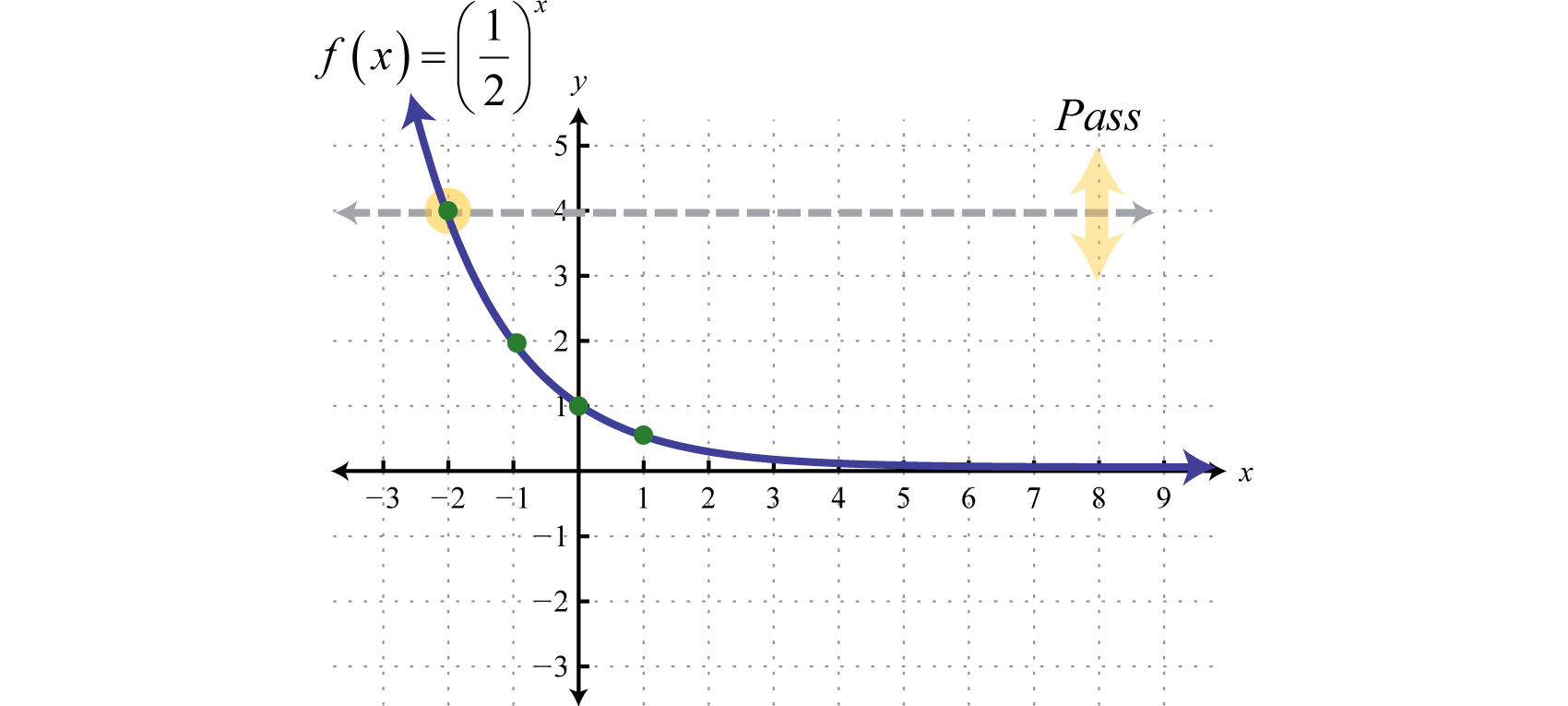Therefore this function is one-to-one and has an inverse. Reflecting the graph about the line $y=x$ we have: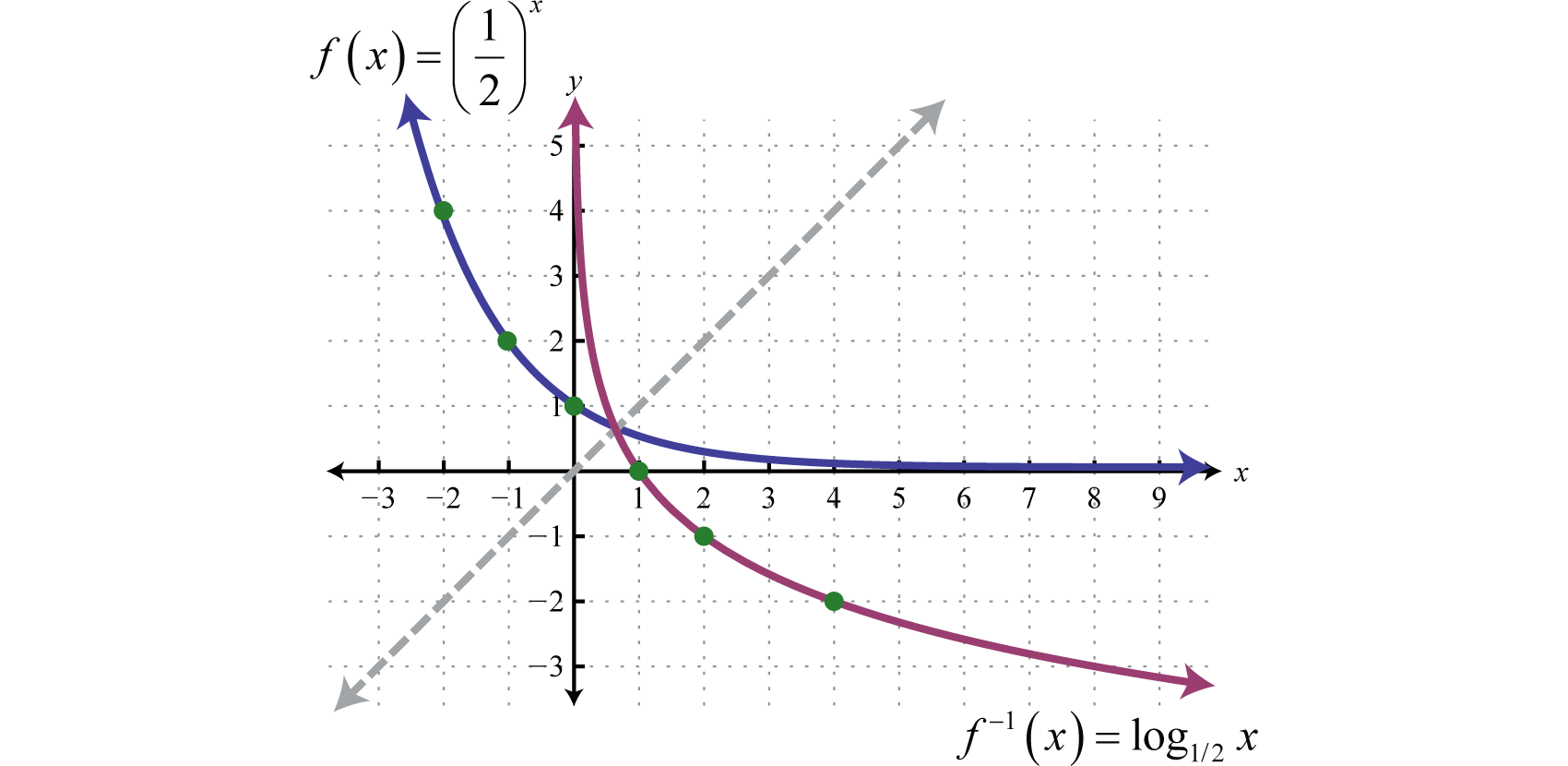which gives us a picture of the graph of $f−1(x)=log1/2 x.$ In general, when the base $b>1$, the graph of the function defined by $g(x)=log1/b x$ has the following shape.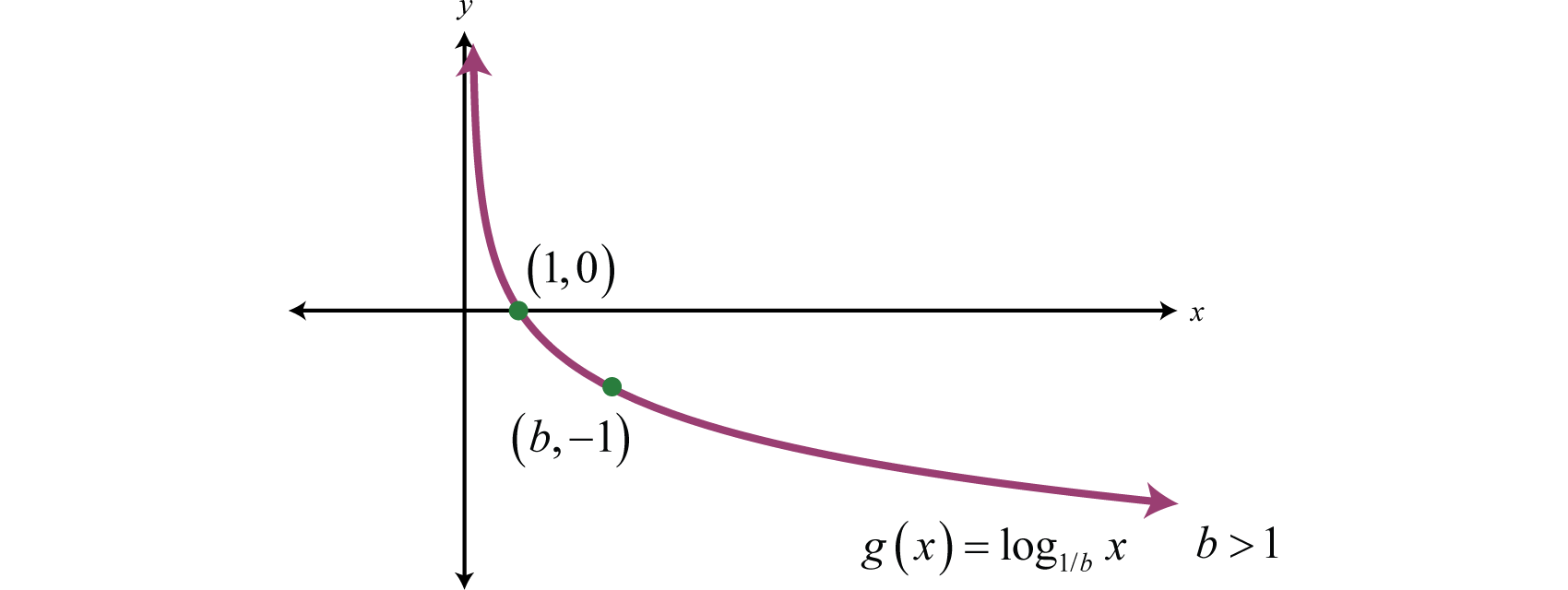The domain consists of positive real numbers, $(0,∞)$ and the range consists of all real numbers, $(−∞,∞).$ The y-axis, or $x=0$, is a vertical asymptote and the x-intercept is $(1,0).$ In addition, $f(b)=log1/b b=−1$ and so $(b,−1)$ is a point on the graph.

### Example 8

Sketch the graph and determine the domain and range: $f(x)=log1/3 (x+3)+2.$

Solution:

Begin by identifying the basic graph and the transformations.

$y=log1/3 x Basic graphy=log1/3 (x+3) Shift left 3 units.y=log1/3 (x+3)+2 Shift up 2 units.$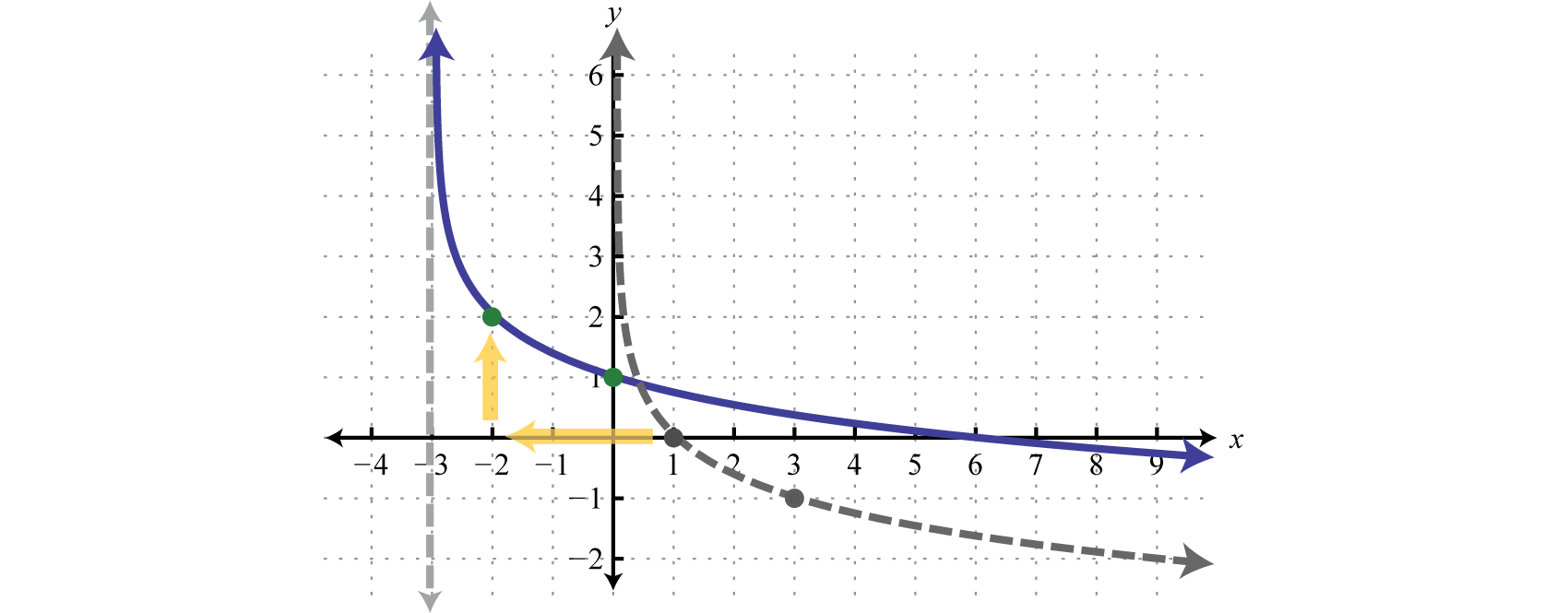In this case the shift left 3 units moved the vertical asymptote to $x=−3$ which defines the lower bound of the domain.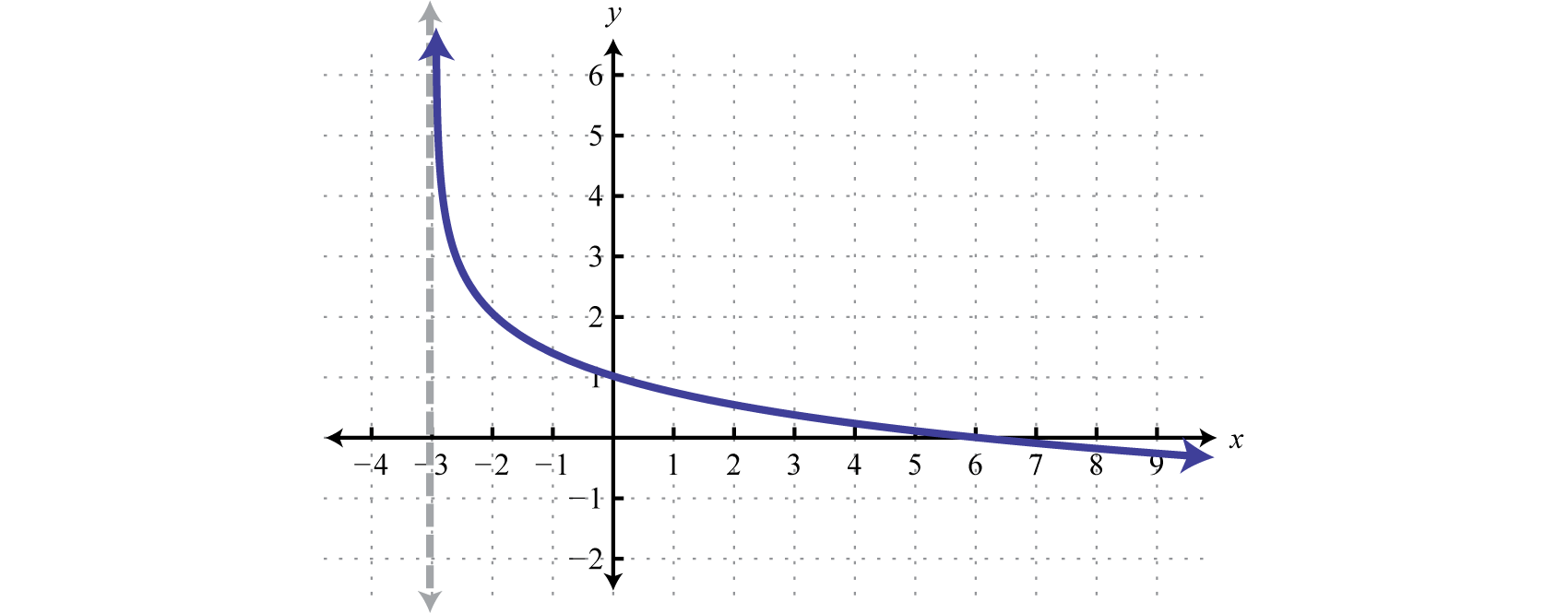Domain: $(−3,∞)$; Range: $(−∞,∞)$

In summary, if $b>1$And for both cases,

$Domain:(0,∞)Range:(−∞,∞)x-intercept:(1,0)Asymptote:x=0$

Try this! Sketch the graph and determine the domain and range: $f(x)=log1/3 (x−1).$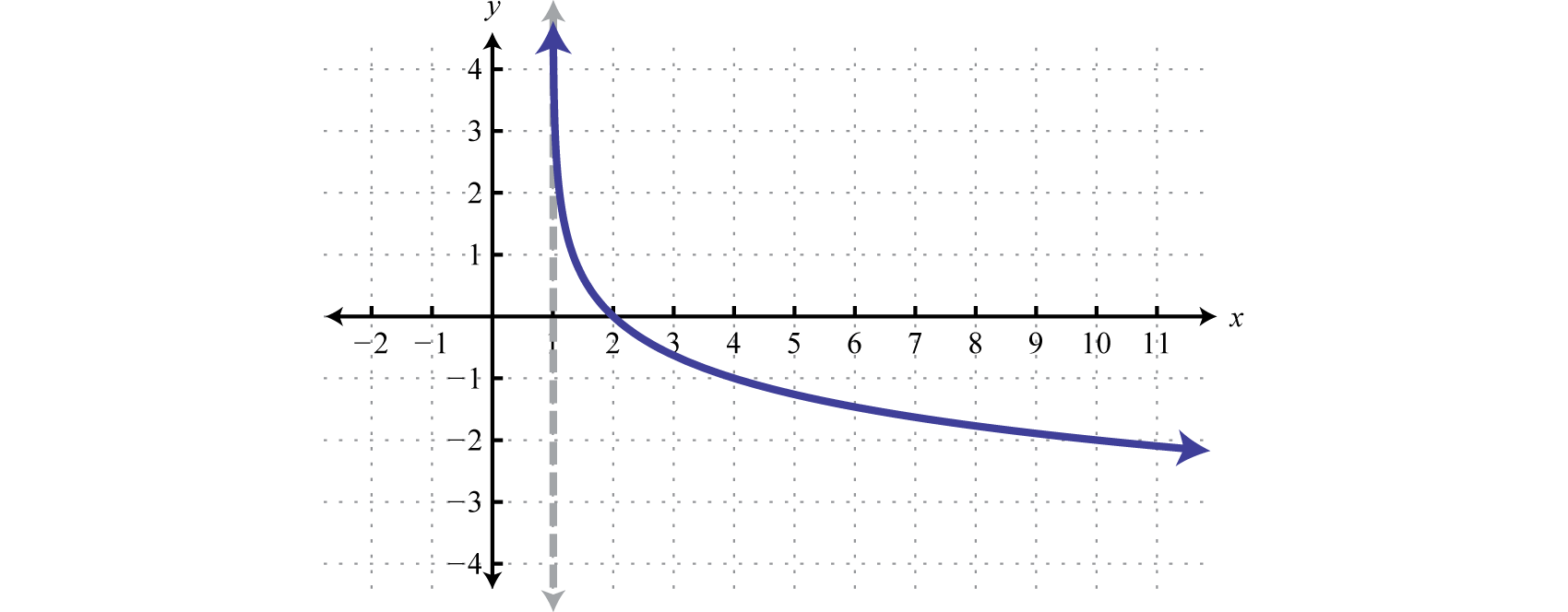### Key Takeaways

• The base-b logarithmic function is defined to be the inverse of the base-b exponential function. In other words, $y=logb x$ if and only if $by=x$ where $b>0$ and $b≠1.$
• The logarithm is actually the exponent to which the base is raised to obtain its argument.
• The logarithm base 10 is called the common logarithm and is denoted $log x.$
• The logarithm base e is called the natural logarithm and is denoted $ln x.$
• Logarithmic functions with definitions of the form $f(x)=logb x$ have a domain consisting of positive real numbers $(0,∞)$ and a range consisting of all real numbers $(−∞,∞).$ The y-axis, or $x=0$, is a vertical asymptote and the x-intercept is $(1,0).$
• To graph logarithmic functions we can plot points or identify the basic function and use the transformations. Be sure to indicate that there is a vertical asymptote by using a dashed line. This asymptote defines the boundary of the domain.

### Part A: Definition of the Logarithm

Evaluate.

1. $log3 9$

2. $log7 49$

3. $log4 4$

4. $log5 1$

5. $log5 625$

6. $log3 243$

7. $log2 (116)$
8. $log3 (19)$
9. $log5 (1125)$
10. $log2 (164)$
11. $log4 410$

12. $log9 95$

13. $log5 53$

14. $log2 2$

15. $log7 (17)$
16. $log9 (193)$
17. $log1/2 4$

18. $log1/3 27$

19. $log2/3 (23)$
20. $log3/4 (916)$
21. $log25 5$

22. $log8 2$

23. $log4 (12)$
24. $log27 (13)$
25. $log1/9 1$
26. $log3/5 (53)$

Find x.

1. $log3 x=4$

2. $log2 x=5$

3. $log5 x=−3$

4. $log6 x=−2$

5. $log12 x=0$

6. $log7 x=−1$

7. $log1/4 x=−2$

8. $log2/5 x=2$

9. $log1/9 x=12$

10. $log1/4 x=32$

11. $log1/3 x=−1$

12. $log1/5 x=0$

### Part B: The Common and Natural Logarithm

Evaluate. Round off to the nearest hundredth where appropriate.

1. $log 1000$

2. $log 100$

3. $log 0.1$

4. $log 0.0001$

5. $log 162$

6. $log 23$

7. $log 0.025$

8. $log 0.235$

9. $ln e4$

10. $ln 1$

11. $ln (1e)$
12. $ln (1e5)$
13. $ln (25)$

14. $ln (100)$

15. $ln (0.125)$

16. $ln (0.001)$

Find x. Round off to the nearest hundredth.

1. $log x=2.5$

2. $log x=1.8$

3. $log x=−1.22$

4. $log x=−0.8$

5. $ln x=3.1$

6. $ln x=1.01$

7. $ln x=−0.69$

8. $ln x=−1$

Find a without using a calculator.

1. $log3 (127)=a$

2. $ln e=a$

3. $log2 a=8$

4. $log2 25=a$

5. $log1012 =a$

6. $ln a=9$

7. $log1/8 (164)=a$

8. $log6 a=−3$

9. $ln a=15$
10. $log4/9 (23)=a$

In 1935 Charles Richter developed a scale used to measure earthquakes on a seismograph. The magnitude M of an earthquake is given by the formula,

$M=log (II0)$

Here I represents the intensity of the earthquake as measured on the seismograph 100 km from the epicenter and $I0$ is the minimum intensity used for comparison. For example, if an earthquake intensity is measured to be 100 times that of the minimum, then $I=100I0$ and

$M=log (100I0I0)=log (100)=2$

The earthquake would be said to have a magnitude 2 on the Richter scale. Determine the magnitudes of the following intensities on the Richter scale. Round off to the nearest tenth.

1. I is 3 million times that of the minimum intensity.

2. I is 6 million times that of the minimum intensity.

3. I is the same as the minimum intensity.

4. I is 30 million times that of the minimum intensity.

In chemistry, pH is a measure of acidity and is given by the formula,

$pH=−log (H+)$

Here $H+$ represents the hydrogen ion concentration (measured in moles of hydrogen per liter of solution.) Determine the pH given the following hydrogen ion concentrations.

1. Pure water: $H+=0.0000001$

2. Blueberry: $H+=0.0003162$

3. Lemon Juice: $H+=0.01$

4. Battery Acid: $H+=0.1$

### Part C: Graphing Logarithmic Functions

Sketch the function and determine the domain and range. Draw the vertical asymptote with a dashed line.

1. $f(x)=log2 (x+1)$

2. $f(x)=log3 (x−2)$

3. $f(x)=log2 x−2$

4. $f(x)=log3 x+3$

5. $f(x)=log2 (x−2)+4$

6. $f(x)=log3 (x+1)−2$

7. $f(x)=−log2 x+1$

8. $f(x)=−log3 (x+3)$

9. $f(x)=log2 (−x)+1$

10. $f(x)=2−log3 (−x)$

11. $f(x)=log x+5$

12. $f(x)=log x−1$

13. $f(x)=log (x+4)−8$

14. $f(x)=log (x−5)+10$

15. $f(x)=−log (x+2)$

16. $f(x)=−log (x−1)+2$

17. $f(x)=ln (x−3)$

18. $f(x)=ln x+3$

19. $f(x)=ln (x−2)+4$

20. $f(x)=ln (x+5)$

21. $f(x)=2−ln x$

22. $f(x)=−ln (x−1)$

23. $f(x)=log1/2 x$

24. $f(x)=log1/3 x+2$

25. $f(x)=log1/2 (x−2)$

26. $f(x)=log1/3 (x+1)−1$

27. $f(x)=2−log1/4 x$

28. $f(x)=1+log1/4 (−x)$

29. $f(x)=1−log1/3 (x−2)$

30. $f(x)=1+log1/2 (−x)$

### Part D: Discussion Board

1. Research and discuss the origins and history of the logarithm. How did students work with them before the common availability of calculators?

2. Research and discuss the history and use of the Richter scale. What does each unit on the Richter scale represent?

3. Research and discuss the life and contributions of John Napier.

1. 2

2. 1

3. 4

4. −4

5. −3

6. 10

7. $13$

8. $−12$

9. −2

10. 1

11. $12$

12. $−12$

13. 0

14. 81

15. $1125$

16. 1

17. 16

18. $13$

19. 3

1. 3

2. −1

3. 2.21

4. −1.60

5. 4

6. −1

7. 3.22

8. −2.08

9. 316.23

10. 0.06

11. 22.20

12. 0.50

13. −3

14. 256

15. 12

16. 2

17. $e5$

18. 6.5

19. 0

20. 7

21. 2

1.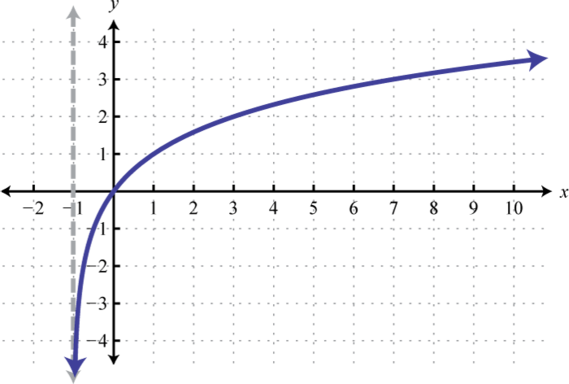Domain: $(−1,∞)$; Range: $(−∞,∞)$

2.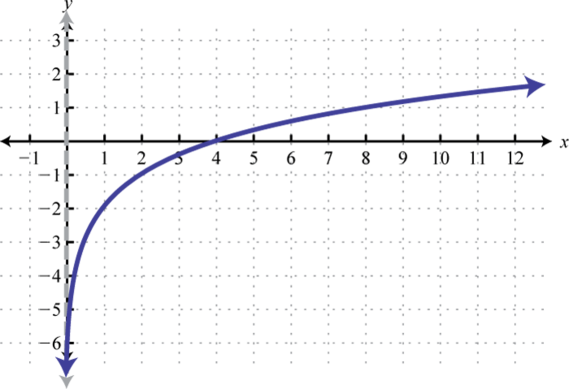Domain: $(0,∞)$; Range: $(−∞,∞)$

3.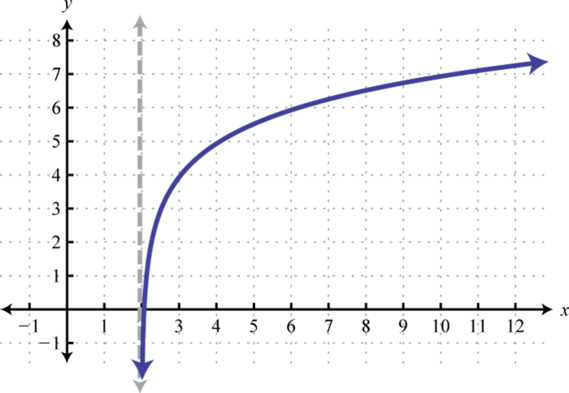Domain: $(2,∞)$; Range: $(−∞,∞)$

4.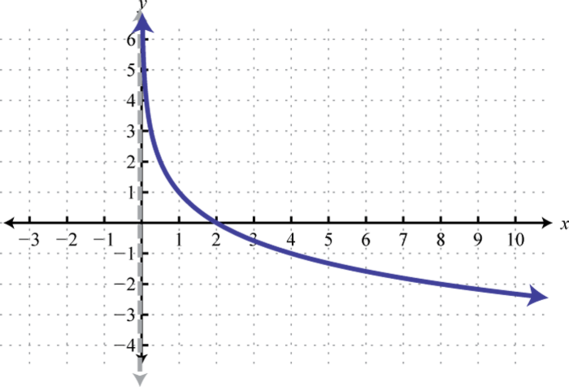Domain: $(0,∞)$; Range: $(−∞,∞)$

5.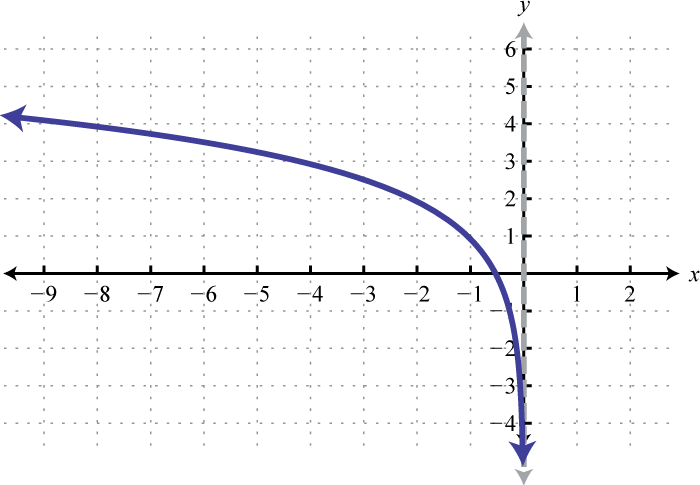Domain: $(−∞,0)$; Range: $(−∞,∞)$

6.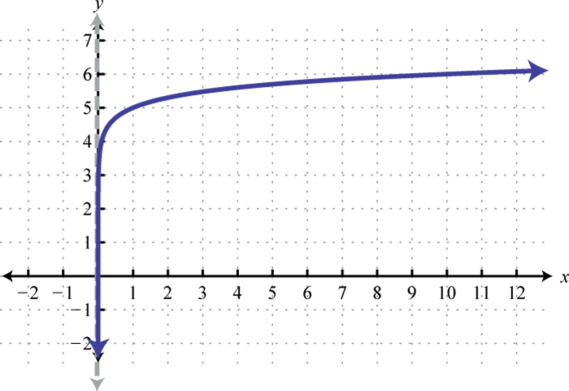Domain: $(0,∞)$; Range: $(−∞,∞)$

7.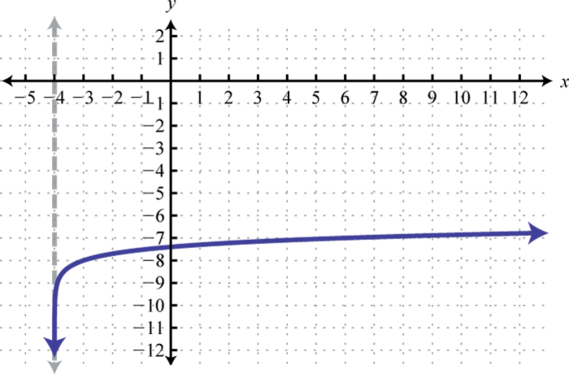Domain: $(−4,∞)$; Range: $(−∞,∞)$

8.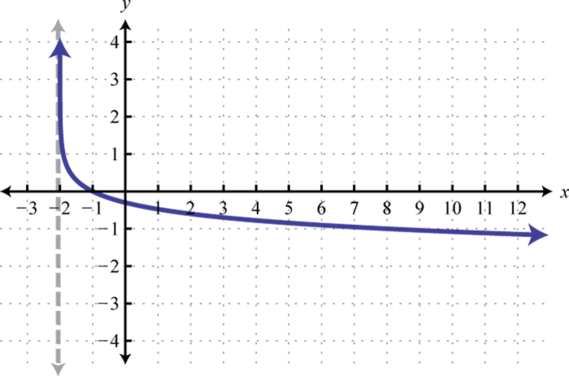Domain: $(−2,∞)$; Range: $(−∞,∞)$

9.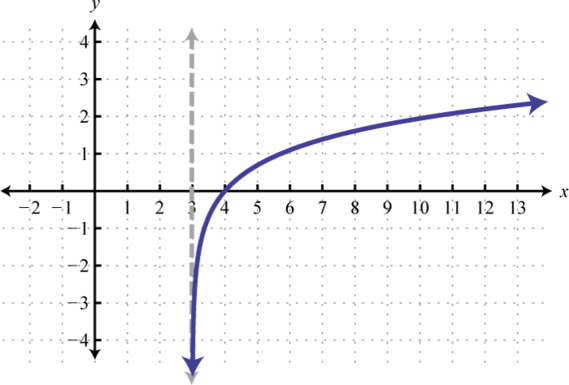Domain: $(3,∞)$; Range: $(−∞,∞)$

10.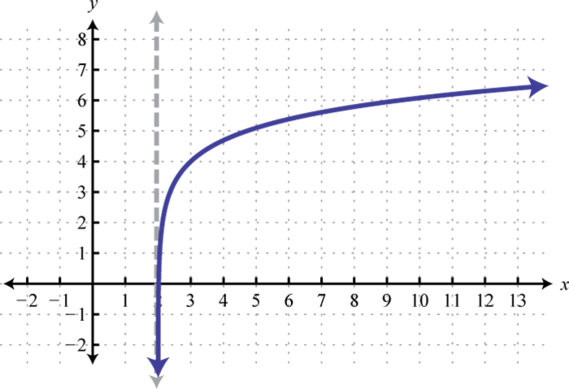Domain: $(2,∞)$; Range: $(−∞,∞)$

11.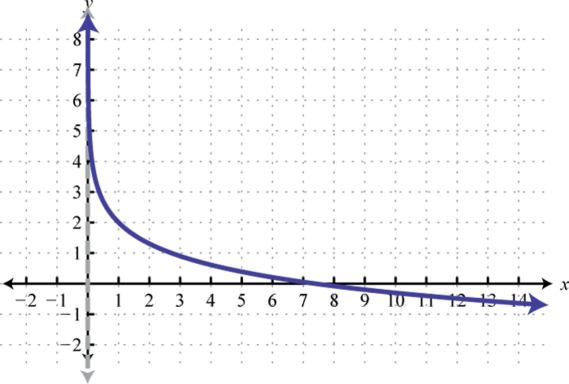Domain: $(0,∞)$; Range: $(−∞,∞)$

12.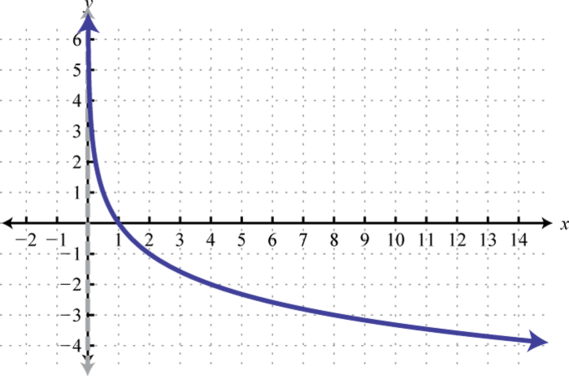Domain: $(0,∞)$; Range: $(−∞,∞)$

13.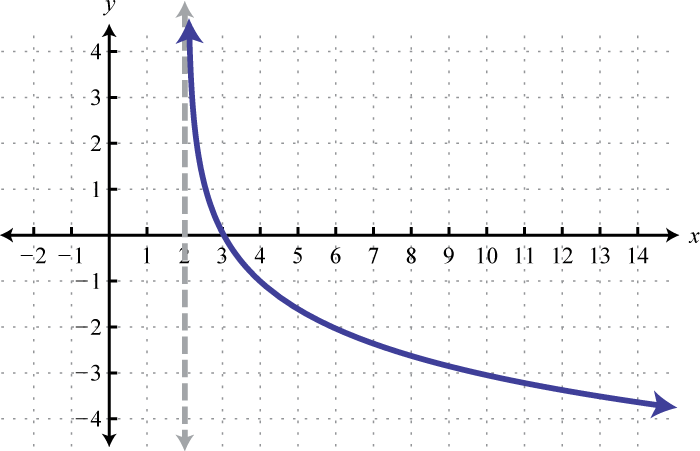Domain: $(2,∞)$; Range: $(−∞,∞)$

14.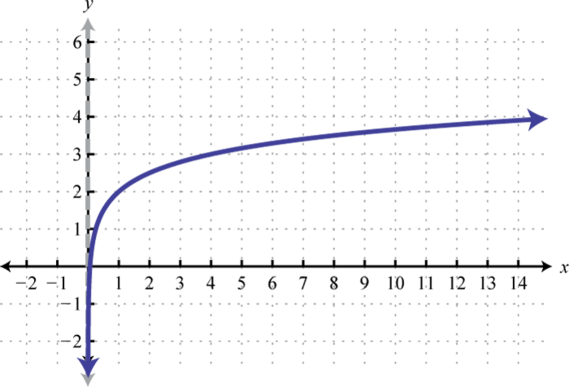Domain: $(0,∞)$; Range: $(−∞,∞)$

15.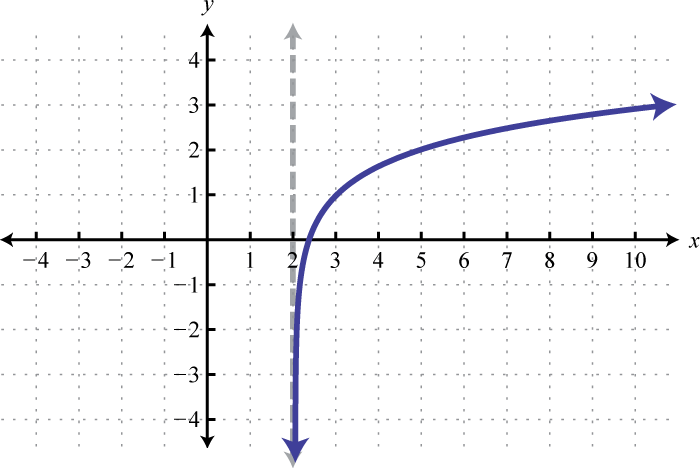Domain: $(2, ∞)$; Range: $(−∞, ∞)$

## 7.4 Properties of the Logarithm

### Learning Objectives

1. Apply the inverse properties of the logarithm.
2. Expand logarithms using the product, quotient, and power rule for logarithms.
3. Combine logarithms into a single logarithm with coefficient 1.

## Logarithms and Their Inverse Properties

Recall the definition of the base-b logarithm: given $b>0$ where $b≠1$,

$y=logb x if and only if x=by$

Use this definition to convert logarithms to exponential form. Doing this, we can derive a few properties:

$logb 1=0 because b0=1logb b=1 because b1=blogb (1b)=−1 because b−1=1b$

### Example 1

Evaluate:

1. $log 1$
2. $ln e$
3. $log5 (15)$

Solution:

1. When the base is not written, it is assumed to be 10. This is the common logarithm,

$log 1=log10 1=0$

2. The natural logarithm, by definition, has base e,

$ln e=loge e=1$

3. Because $5−1=15$ we have,

$log5 (15)=−1$

Furthermore, consider fractional bases of the form $1/b$ where $b>1.$

$log1/b b=−1 because (1b)−1=1−1b−1=b1=b$

### Example 2

Evaluate:

1. $log1/4 4$
2. $log2/3 (32)$

Solution:

1. $log1/4 4=−1$ because $(14)−1=4$
2. $log2/3 (32)=−1$ because $(23)−1=32$

Given an exponential function defined by $f(x)=bx$, where $b>0$ and $b≠1$, its inverse is the base-b logarithm, $f−1(x)=logb x.$ And because $f(f−1(x))=x$ and $f−1(f(x))=x$, we have the following inverse properties of the logarithmGiven $b>0$ we have $logb bx=x$ and $blogb x=x$ when $x>0.$:

$f−1(f(x))=logb bx=xandf(f−1(x))=blogb x=x ,x>0$

Since $f−1(x)=logb x$ has a domain consisting of positive values $(0,∞)$, the property $blogb x=x$ is restricted to values where $x>0.$

### Example 3

Evaluate:

1. $log5 625$
2. $5log5 3$
3. $eln 5$

Solution:

Apply the inverse properties of the logarithm.

1. $log5 625=log5 54=4$
2. $5log5 3=3$
3. $eln 5=5$

In summary, when $b>0$ and $b≠1$, we have the following properties:

 $logb 1=0$ $logb b=1$ $log1/b b=−1$ $logb (1b)=−1$ $logb bx=x$ $blogb x=x$, $x>0$

Try this! Evaluate: $log 0.00001$

## Product, Quotient, and Power Properties of Logarithms

In this section, three very important properties of the logarithm are developed. These properties will allow us to expand our ability to solve many more equations. We begin by assigning u and v to the following logarithms and then write them in exponential form:

$logb x=u ⇒ bu=xlogb y=v ⇒ bv=y$

Substitute $x=bu$ and $y=bv$ into the logarithm of a product $logb (xy)$ and the logarithm of a quotient $logb (xy).$ Then simplify using the rules of exponents and the inverse properties of the logarithm.

Logarithm of a Product

Logarithm of a Quotient

$logb (xy)=logb (bubv)=logb bu+v=u+v=logb x+logb y$

$logb (xy)=logb (bubv)=logb bu−v=u−v=logb x−logb y$

This gives us two essential properties: the product property of logarithms$logb (xy)=logb x+logb y;$ the logarithm of a product is equal to the sum of the logarithm of the factors.,

$logb (xy)=logb x+logb y$

and the quotient property of logarithms$logb (xy)=logb x−logb y;$ the logarithm of a quotient is equal to the difference of the logarithm of the numerator and the logarithm of the denominator.,

$logb (xy)=logb x−logb y$

In words, the logarithm of a product is equal to the sum of the logarithm of the factors. Similarly, the logarithm of a quotient is equal to the difference of the logarithm of the numerator and the logarithm of the denominator.

### Example 4

Write as a sum: $log2 (8x).$

Solution:

Apply the product property of logarithms and then simplify.

$log2 (8x)=log2 8+log2 x=log2 23+log2 x=3+log2 x$

Answer: $3+log2 x$

### Example 5

Write as a difference: $log (x10)$.

Solution:

Apply the quotient property of logarithms and then simplify.

$log (x10)=log x−log 10=log x−1$

Answer: $log x−1$

Next we begin with $logb x=u$ and rewrite it in exponential form. After raising both sides to the nth power, convert back to logarithmic form, and then back substitute.

$logb x=u ⇒ bu=x(bu)n=(x )nlogb xn=nu ⇐ bnu=xnlogb xn=nlogb x$

This leads us to the power property of logarithms$logb xn=nlogb x$; the logarithm of a quantity raised to a power is equal to that power times the logarithm of the quantity.,

$logb xn=nlogb x$

In words, the logarithm of a quantity raised to a power is equal to that power times the logarithm of the quantity.

### Example 6

Write as a product:

1. $log2 x4$
2. $log5 (x).$

Solution:

1. Apply the power property of logarithms.

$log2 x4=4log2 x$

2. Recall that a square root can be expressed using rational exponents, $x=x1/2.$ Make this replacement and then apply the power property of logarithms.

$log5 (x)=log5 x1/2=12log5 x$

In summary,

 Product property of logarithms $logb (xy)=logb x+logb y$ Quotient property of logarithms $logb (xy)=logb x−logb y$ Power property of logarithms $logb xn=nlogb x$

We can use these properties to expand logarithms involving products, quotients, and powers using sums, differences and coefficients. A logarithmic expression is completely expanded when the properties of the logarithm can no further be applied.

$Caution:$ It is important to point out the following:

$log (xy)≠log x⋅log y and log (xy)≠log xlog y$

### Example 7

Expand completely: $ln (2x3).$

Solution:

Recall that the natural logarithm is a logarithm base e, $ln x=loge x.$ Therefore, all of the properties of the logarithm apply.

$ln (2x3)=ln 2+ln x3 Product rule for logarithms=ln 2+3ln x Power rule for logarithms$

Answer: $ln 2+3ln x$

### Example 8

Expand completely: $log 10xy23.$

Solution:

Begin by rewriting the cube root using the rational exponent $13$ and then apply the properties of the logarithm.

$log 10xy23=log (10xy2)1/3=13log (10xy2)=13(log 10+log x+log y2)=13(1+log x+2log y)=13+13log x+23log y$

Answer: $13+13log x+23log y$

### Example 9

Expand completely: $log2 ( (x+1)25y)$.

Solution:

When applying the product property to the denominator, take care to distribute the negative obtained from applying the quotient property.

$log2 ( (x+1)25y)=log2 (x+1)2−log2 (5y)=log2 (x+1)2−(log2 5+log2 y) Distribute.=log2 (x+1)2−log2 5−log2 y=2log2 (x+1)−log2 5−log2 y$

Answer: $2log2 (x+1)−log2 5−log2 y$

Caution: There is no rule that allows us to expand the logarithm of a sum or difference. In other words,

$log (x±y)≠log x±log y$

Try this! Expand completely: $ln (5y4x)$.

Answer: $ln 5+4ln y−12ln x$

### Example 10

Given that $log2 x=a$, $log2 y=b$, and that $log2 z=c$, write the following in terms of a, b and c:

a. $log2 (8x2y)$

b. $log2 (2x4z)$

Solution:

1. Begin by expanding using sums and coefficients and then replace a and b with the appropriate logarithm.

$log2 (8x2y)=log2 8+log2 x2+log2 y=log2 8+2log2 x+log2 y=3+2a+b$

2. Expand and then replace a, b, and c where appropriate.

$log2 (2x4z)=log2 (2x4)−log2 z1/2=log2 2+log2 x4−log2 z1/2=log2 2+4log2 x−12log2 z=1+4a−12b$

Next we will condense logarithmic expressions. As we will see, it is important to be able to combine an expression involving logarithms into a single logarithm with coefficient 1. This will be one of the first steps when solving logarithmic equations.

### Example 11

Write as a single logarithm with coefficient $1: 3log3 x−log3 y+2log3 5.$

Solution:

Begin by rewriting all of the logarithmic terms with coefficient 1. Use the power rule to do this. Then use the product and quotient rules to simplify further.

$3log3x−log3y+2log35={log3x3−log3y}+log352 quotient property={log3(x3y)+log325} product property=log3(x3y⋅25)=log3(25x3y)$

Answer: $log3 (25x3y)$

### Example 12

Write as a single logarithm with coefficient $1: 12ln x−3ln y−ln z.$

Solution:

Begin by writing the coefficients of the logarithms as powers of their argument, after which we will apply the quotient rule twice working from left to right.

$12ln x−3ln y−ln z=ln x1/2−ln y3−ln z=ln (x1/2y3)−ln z=ln (x1/2y3÷z)=ln (x1/2y3⋅1z)=ln (x1/2y3z) or =ln (xy3z)$

Answer: $ln (xy3z)$

Try this! Write as a single logarithm with coefficient $1: 3log (x+y)−6log z+2log 5.$

Answer: $log (25(x+y)3z6)$

### Key Takeaways

• Given any base $b>0$ and $b≠1$, we can say that $logb 1=0$, $logb b=1$, $log1/b b=−1$ and that $logb (1b)=−1.$
• The inverse properties of the logarithm are $logb bx=x$ and $blogb x=x$ where $x>0.$
• The product property of the logarithm allows us to write a product as a sum: $logb (xy)=logb x+logb y.$
• The quotient property of the logarithm allows us to write a quotient as a difference: $logb (xy)=logb x−logb y.$
• The power property of the logarithm allows us to write exponents as coefficients: $logb xn=nlogb x.$
• Since the natural logarithm is a base-e logarithm, $ln x=loge x$, all of the properties of the logarithm apply to it.
• We can use the properties of the logarithm to expand logarithmic expressions using sums, differences, and coefficients. A logarithmic expression is completely expanded when the properties of the logarithm can no further be applied.
• We can use the properties of the logarithm to combine expressions involving logarithms into a single logarithm with coefficient 1. This is an essential skill to be learned in this chapter.

### Part A: Logarithms and Their Inverse Properties

Evaluate:

1. $log7 1$

2. $log1/2 2$

3. $log 1014$

4. $log 10−23$

5. $log3 310$

6. $log6 6$

7. $ln e7$

8. $ln (1e)$
9. $log1/2 (12)$
10. $log1/5 5$

11. $log3/4 (43)$

12. $log2/3 1$

13. $2log2 100$

14. $3log3 1$

15. $10log 18$

16. $eln 23$

17. $eln x2$

18. $eln ex$

Find a:

1. $ln a=1$

2. $log a=−1$

3. $log9 a=−1$

4. $log12 a=1$

5. $log2 a=5$

6. $log a=13$

7. $2a=7$

8. $ea=23$

9. $loga 45=5$

10. $loga 10=1$

### Part B: Product, Quotient, and Power Properties of Logarithms

Expand completely.

1. $log4 (xy)$

2. $log (6x)$

3. $log3 (9x2)$

4. $log2 (32x7)$

5. $ln (3y2)$

6. $log (100x2)$

7. $log2 (xy2)$
8. $log5 (25x)$
9. $log (10x2y3)$

10. $log2 (2x4y5)$

11. $log3 (x3yz2)$
12. $log (xy3z2)$
13. $log5 (1x2yz)$
14. $log4 (116x2z3)$
15. $log6 [36(x+y)4]$

16. $ln [e4(x−y)3]$

17. $log7 (2xy)$

18. $ln (2xy)$

19. $log3 (x2y3z)$
20. $log (2(x+y)3z2)$
21. $log (100x3(y+10)3)$
22. $log7 (x(y+z)35)$
23. $log5 (x3yz23)$
24. $log (x2y3z25)$

Given $log3 x=a$, $log3 y=b$, and $log3 z=c$, write the following logarithms in terms of a, b, and c.

1. $log3 (27x2y3z)$
2. $log3 (xy3z)$
3. $log3 (9x2yz3)$
4. $log3 (x3yz2)$

Given $logb 2=0.43$, $logb 3=0.68$, and $logb 7=1.21$, calculate the following. (Hint: Expand using sums, differences, and quotients of the factors 2, 3, and 7.)

1. $logb 42$

2. $logb (36)$

3. $logb (289)$

4. $logb 21$

Expand using the properties of the logarithm and then approximate using a calculator to the nearest tenth.

1. $log (3.10×1025)$

2. $log (1.40×10−33)$

3. $ln (6.2e−15)$

4. $ln (1.4e22)$

Write as a single logarithm with coefficient 1.

1. $log x+log y$

2. $log3 x−log3 y$

3. $log2 5+2log2 x+log2 y$

4. $log3 4+3log3 x+12log3 y$

5. $3log2 x−2log2 y+12log2 z$

6. $4log x−log y−log 2$

7. $log 5+3log (x+y)$

8. $4log5 (x+5)+log5 y$

9. $ln x−6ln y+ln z$

10. $log3 x−2log3 y+5log3 z$

11. $7log x−log y−2log z$

12. $2ln x−3ln y−ln z$

13. $23log3 x−12(log3 y+log3 z)$

14. $15(log7 x+2log7 y)−2log7 (z+1)$

15. $1+log2 x−12log2 y$

16. $2−3log3 x+13log3 y$

17. $13log2 x+23log2 y$

18. $−2log5 x+35log5 y$

19. $−ln 2+2ln (x+y)−ln z$

20. $−3ln (x−y)−ln z+ln 5$

21. $13(ln x+2ln y)−(3ln 2+ln z)$

22. $4log 2+23log x−4log (y+z)$

23. $log2 3−2log2 x+12log2 y−4log2 z$

24. $2log5 4−log5 x−3log5 y+23log5 z$

Express as a single logarithm and simplify.

1. $log (x+1)+log (x−1)$

2. $log2 (x+2)+log2 (x+1)$

3. $ln (x2+2x+1)−ln (x+1)$

4. $ln (x2−9)−ln (x+3)$

5. $log5 (x3−8)−log5 (x−2)$

6. $log3 (x3+1)−log3 (x+1)$

7. $log x+log (x+5)−log (x2−25)$

8. $log (2x+1)+log (x−3)−log (2x2−5x−3)$

1. 0

2. 14

3. 10

4. 7

5. 1

6. −1

7. 100

8. 18

9. $x2$

10. $e$

11. $19$

12. $25=32$

13. $log2 7$

14. 4

1. $log4 x+log4 y$

2. $2+2log3 x$

3. $ln 3+2ln y$

4. $log2 x−2log2 y$

5. $1+2log x+3log y$

6. $3log3 x−log3 y−2log3 z$

7. $−2log5 x−log5 y−log5 z$

8. $2+4log6 (x+y)$

9. $log7 2+12log7 x+12log7 y$

10. $2log3 x+13log3 y−log3 z$

11. $2+3log x−3log (y+10)$

12. $3log5 x−13log5 y−23log5 z$

13. $3+2a+3b+c$

14. $2+2a+b−3c$

15. 2.32

16. 0.71

17. $log (3.1)+25≈25.5$

18. $ln (6.2)−15≈−13.2$

19. $log (xy)$

20. $log2 (5x2y)$

21. $log2 (x3zy2)$
22. $log [5(x+y)3]$

23. $ln (xzy6)$
24. $log (x7yz2)$
25. $log3 (x23yz)$
26. $log2 (2xy)$
27. $log2 (xy23)$
28. $ln ((x+y)22z)$
29. $ln (xy238z)$
30. $log2 (3yx2z4)$
31. $log (x2−1)$

32. $ln (x+1)$

33. $log5 (x2+2x+4)$

34. $log (xx−5)$

## 7.5 Solving Exponential and Logarithmic Equations

### Learning Objectives

1. Solve exponential equations.
2. Use the change of base formula to approximate logarithms.
3. Solve logarithmic equations.

## Solving Exponential Equations

An exponential equationAn equation which includes a variable as an exponent. is an equation that includes a variable as one of its exponents. In this section we describe two methods for solving exponential equations. First, recall that exponential functions defined by $f(x)=bx$ where $b>0$ and $b≠1$, are one-to-one; each value in the range corresponds to exactly one element in the domain. Therefore, $f(x)=f(y)$ implies $x=y.$ The converse is true because f is a function. This leads to the very important one-to-one property of exponential functionsGiven $b>0$ and $b≠1$ we have $bx=by$ if and only if $x=y.$:

$bx=by if and only if x=y$

Use this property to solve special exponential equations where each side can be written in terms of the same base.

### Example 1

Solve: $32x−1=27.$

Solution:

Begin by writing 27 as a power of 3.

$32x−1=2732x−1=33$

Next apply the one-to-one property of exponential functions. In other words, set the exponents equal to each other and then simplify.

$2x−1=32x=4x=2$

### Example 2

Solve: $161−3x=2.$

Solution:

Begin by writing 16 as a power of 2 and then apply the power rule for exponents.

$161−3x=2(24)1−3x=224(1−3x)=21$

Now that the bases are the same we can set the exponents equal to each other and simplify.

$4(1−3x)=14−12x=1−12x=−3x=−3−12=14$

Answer: $14$

Try this! Solve: $252x+3=125.$

Answer: $−34$

In many cases we will not be able to equate the bases. For this reason we develop a second method for solving exponential equations. Consider the following equations:

$32=93?=1233=27$

We can see that the solution to $3x=12$ should be somewhere between 2 and 3. A graphical interpretation follows.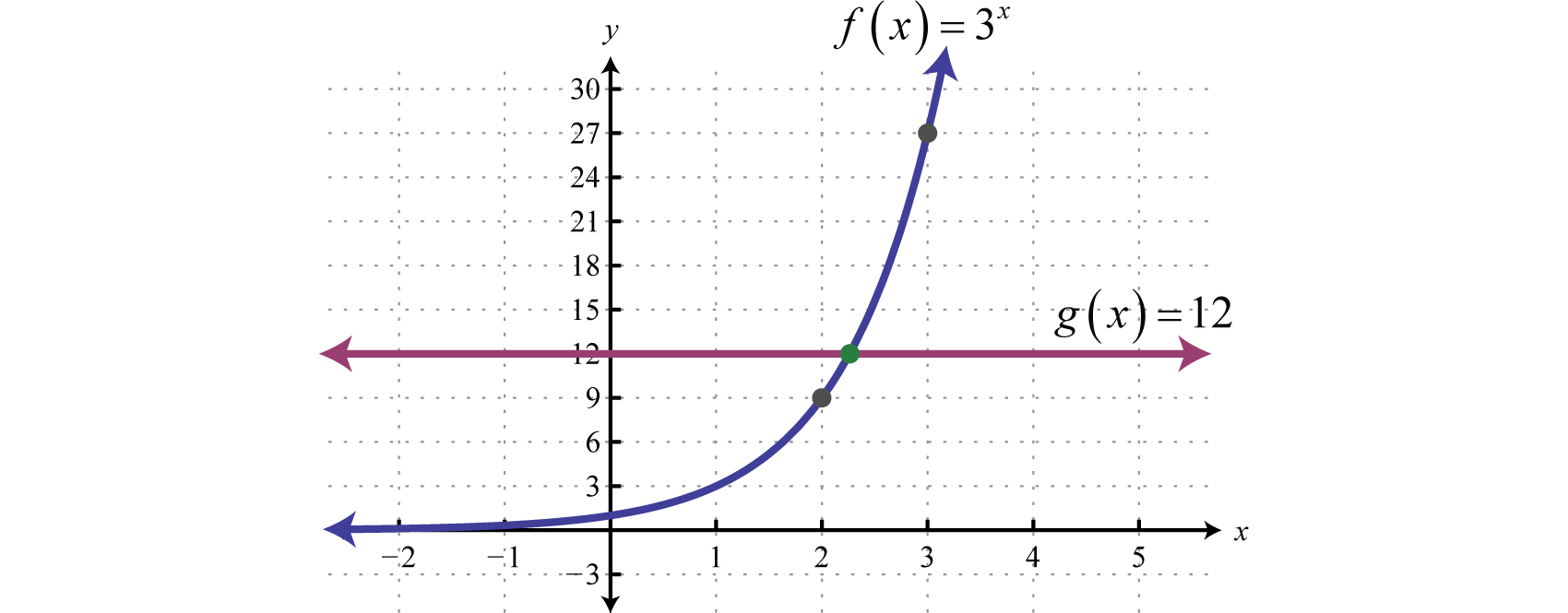To solve this we make use of fact that logarithms are one-to-one functions. Given $x,y>0$ the one-to-one property of logarithmsGiven $b>0$ and $b≠1$ where $x,y>0$ we have $logb x=logb y$ if and only if $x=y.$ follows:

$logb x=logb y if and only if x=y$

This property, as well as the properties of the logarithm, allows us to solve exponential equations. For example, to solve $3x=12$ apply the common logarithm to both sides and then use the properties of the logarithm to isolate the variable.

$3x=12log 3x=log 12 One-to-one property of logarithmsxlog 3=log 12 Power rule for logarithmsx=log 12log 3$

Approximating to four decimal places on a calculator.

$x=log (12)/log (3)≈2.2619$

An answer between 2 and 3 is what we expected. Certainly we can check by raising 3 to this power to verify that we obtain a good approximation of 12.

$3^2.2618≈12✓$

Note that we are not multiplying both sides by “log”; we are applying the one-to-one property of logarithmic functions — which is often expressed as “taking the log of both sides.” The general steps for solving exponential equations are outlined in the following example.

### Example 3

Solve: $52x−1+2=9.$

Solution:

• Step 1: Isolate the exponential expression.

$52x−1+2=952x−1=7$

• Step 2: Take the logarithm of both sides. In this case, we will take the common logarithm of both sides so that we can approximate our result on a calculator.

$log 52x−1=log 7$

• Step 3: Apply the power rule for logarithms and then solve.

$log 52x−1=log 7(2x−1)log 5=log 7 Distribute.2xlog 5−log 5=log 72xlog 5=log 5+log 7x=log 5+log 72log 5$

This is an irrational number which can be approximated using a calculator. Take care to group the numerator and the product in the denominator when entering this into your calculator. To do this, make use of the parenthesis buttons $($ and $)$ :

$x=(log 5+log (7))/(2*log (5))≈1.1045$

Answer: $log 5+log 72log 5≈1.1045$

### Example 4

Solve: $e5x+3=1.$

Solution:

The exponential function is already isolated and the base is e. Therefore, we choose to apply the natural logarithm to both sides.

$e5x+3=1ln e5x+3=ln 1$

Apply the power rule for logarithms and then simplify.

$ln e5x+3=ln 1(5x+3)ln e=ln 1 Recall ln e=1 and ln1=0.(5x+3)⋅1=05x+3=0x=−35$

Answer: $−35$

On most calculators there are only two logarithm buttons, the common logarithm $LOG$ and the natural logarithm $LN.$ If we want to approximate $log3 10$ we have to somehow change this base to 10 or e. The idea begins by rewriting the logarithmic function $y=loga x$, in exponential form.

$loga x=y ⇒ x=ay$

Here $x>0$ and so we can apply the one-to-one property of logarithms. Apply the logarithm base b to both sides of the function in exponential form.

$x=aylogb x=logb ay$

And then solve for y.

$logb x=ylogb alogb xlogb a=y$

Replace y into the original function and we have the very important change of base formula$loga x=logb xlogb a$; we can write any base-a logarithm in terms of base-b logarithms using this formula.:

$loga x=logb xlogb a$

We can use this to approximate $log3 10$ as follows.

$log3 10=log 10log 3≈2.0959 or log3 10=ln 10ln 3≈2.0959$

Notice that the result is independent of the choice of base. In words, we can approximate the logarithm of any given base on a calculator by dividing the logarithm of the argument by the logarithm of that given base.

### Example 5

Approximate $log7 120$ the nearest hundredth.

Solution:

Apply the change of base formula and use a calculator.

$log7 120=log 120log 7$

On a calculator,

$log (120)/log (7)≈2.46$

Try this! Solve: $23x+1−4=1.$ Give the exact and approximate answer rounded to four decimal places.

Answer: $log 5−log 23log 2≈0.4406$

## Solving Logarithmic Equations

A logarithmic equationAn equation that involves a logarithm with a variable argument. is an equation that involves a logarithm with a variable argument. Some logarithmic equations can be solved using the one-to-one property of logarithms. This is true when a single logarithm with the same base can be obtained on both sides of the equal sign.

### Example 6

Solve: $log2 (2x−5)−log2 (x−2)=0.$

Solution:

We can obtain two equal logarithms base 2 by adding $log2 (x−2)$ to both sides of the equation.

$log2 (2x−5)−log2 (x−2)=0log2 (2x−5)=log2 (x−2)$

Here the bases are the same and so we can apply the one-to-one property and set the arguments equal to each other.

$log2 (2x−5)=log2 (x−2)2x−5=x−2x=3$

Checking $x=3$ in the original equation:

$log2 (2(3)−5)=log2 ((3)−2)log2 1=log2 10=0 ✓$

When solving logarithmic equations the check is very important because extraneous solutions can be obtained. The properties of the logarithm only apply for values in the domain of the given logarithm. And when working with variable arguments, such as $log (x−2)$, the value of x is not known until the end of this process. The logarithmic expression $log (x−2)$ is only defined for values $x>2.$

### Example 7

Solve: $log (3x−4)=log (x−2).$

Solution:

Apply the one-to-one property of logarithms (set the arguments equal to each other) and then solve for x.

$log (3x−4)=log (x−2)3x−4=x−22x=2x=1$

When performing the check we encounter a logarithm of a negative number:

$log (x−2)=log (1−2)=log (−1) Undefined$

Try this on a calculator, what does it say? Here $x=1$ is not in the domain of $log (x−2).$ Therefore our only possible solution is extraneous and we conclude that there are no solutions to this equation.

Caution: Solving logarithmic equations sometimes leads to extraneous solutions — we must check our answers.

Try this! Solve: $ln (x2−15)−ln (2x)=0.$

In many cases we will not be able to obtain two equal logarithms. To solve such equations we make use of the definition of the logarithm. If $b>0$, where $b≠1$, then $logb x=y$ implies that $by=x.$ Consider the following common logarithmic equations (base 10),

$log x=0 ⇒ x=1 Because 100=1.log x=0.5 ⇒ x=?log x=1 ⇒ x=10 Because 101=10.$

We can see that the solution to $log x=0.5$ will be somewhere between 1 and 10. A graphical interpretation follows.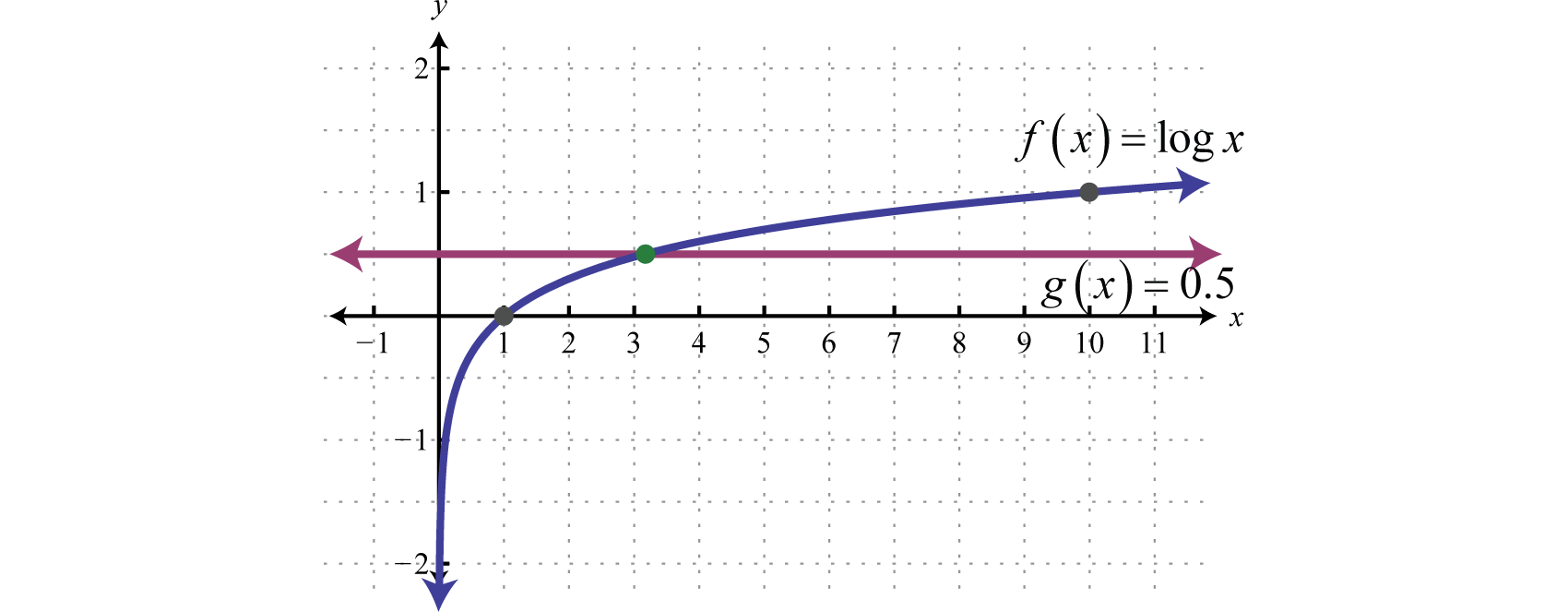To find x we can apply the definition as follows.

$log10 x=0.5 ⇒ 100.5=x$

This can be approximated using a calculator,

$x=100.5=10^0.5≈3.1623$

An answer between 1 and 10 is what we expected. Check this on a calculator.

$log 3.1623≈5✓$

### Example 8

Solve: $log3 (2x−5)=2.$

Solution:

Apply the definition of the logarithm.

$log3 (2x−5)=2 ⇒ 2x−5=32$

Solve the resulting equation.

$2x−5=92x=14x=7$

Check.

$log3 (2(7)−5)=?2 log3 (9)=2 ✓$

In order to apply the definition, we will need to rewrite logarithmic expressions as a single logarithm with coefficient 1.The general steps for solving logarithmic equations are outlined in the following example.

### Example 9

Solve: $log2 (x−2)+log2 (x−3)=1.$

Solution:

• Step 1: Write all logarithmic expressions as a single logarithm with coefficient 1. In this case, apply the product rule for logarithms.

$log2 (x−2)+log2 (x−3)=1log2 [(x−2)(x−3)]=1$

• Step 2: Use the definition and rewrite the logarithm in exponential form.

$log2 [(x−2)(x−3)]=1 ⇒ (x−2)(x−3)=21$

• Step 3: Solve the resulting equation. Here we can solve by factoring.

$(x−2)(x−3)=2x2−5x+6=2x2−5x+4=0(x−4)(x−1)=0x−4=0 or x−1=0x=4x=1$

• Step 4: Check. This step is required.

Check $x=4$

Check $x=1$

$log2 (x−2)+log2 (x−3)=1log2 (4−2)+log2 (4−3)=1log2 (2)+log2 (1)=11+0=1 ✓$

$log2 (x−2)+log2 (x−3)=1log2 (1−2)+log2 (1−3)=1log2 (−1)+log2 (−2)=1 ✗$

In this example, $x=1$ is not in the domain of the given logarithmic expression and is extraneous. The only solution is $x=4.$

### Example 10

Solve: $log (x+15)−1=log (x+6).$

Solution:

Begin by writing all logarithmic expressions on one side and constants on the other.

$log (x+15)−1=log (x+6)log (x+15)−log (x+6)=1$

Apply the quotient rule for logarithms as a means to obtain a single logarithm with coefficient 1.

$log (x+15)−log (x+6)=1log (x+15x+6)=1$

This is a common logarithm; therefore use 10 as the base when applying the definition.

$x+15x+6=101x+15=10(x+6)x+15=10x+60−9x=45x=−5$

Check.

$log (x+15)−1=log (x+6)log (−5+15)−1=log (−5+6)log 10−1=log 11−1=00=0 ✓$

Try this! Solve: $log2 (x)+log2 (x−1)=1.$

### Example 11

Find the inverse: $f(x)=log2 (3x−4).$

Solution:

Begin by replacing the function notation $f(x)$ with y.

$f(x)=log2 (3x−4)y=log2 (3x−4)$

Interchange x and y and then solve for y.

$x=log2 (3y−4) ⇒ 3y−4=2x3y=2x+4y=2x+43$

The resulting function is the inverse of f. Present the answer using function notation.

Answer: $f−1(x)=2x+43$

### Key Takeaways

• If each side of an exponential equation can be expressed using the same base, then equate the exponents and solve.
• To solve a general exponential equation, first isolate the exponential expression and then apply the appropriate logarithm to both sides. This allows us to use the properties of logarithms to solve for the variable.
• The change of base formula allows us to use a calculator to calculate logarithms. The logarithm of a number is equal to the common logarithm of the number divided by the common logarithm of the given base.
• If a single logarithm with the same base can be isolated on each side of an equation, then equate the arguments and solve.
• To solve a general logarithmic equation, first isolate the logarithm with coefficient 1 and then apply the definition. Solve the resulting equation.
• The steps for solving logarithmic equations sometimes produce extraneous solutions. Therefore, the check is required.

### Part A: Solving Exponential Equations

Solve using the one-to-one property of exponential functions.

1. $3x=81$

2. $2−x=16$

3. $5x−1=25$

4. $3x+4=27$

5. $25x−2=16$

6. $23x+7=8$

7. $812x+1=3$

8. $643x−2=2$

9. $92−3x−27=0$

10. $81−5x−32=0$

11. $16x2−2=0$

12. $4x2−1−64=0$

13. $9x(x+1)=81$

14. $4x(2x+5)=64$

15. $100x2−107x−3=0$

16. $e3(3x2−1)−e=0$

Solve. Give the exact answer and the approximate answer rounded to the nearest thousandth.

1. $3x=5$

2. $7x=2$

3. $4x=9$

4. $2x=10$

5. $5x−3=13$

6. $3x+5=17$

7. $72x+5=2$

8. $35x−9=11$

9. $54x+3+6=4$

10. $107x−1−2=1$

11. $e2x−3−5=0$

12. $e5x+1−10=0$

13. $63x+1−3=7$

14. $8−109x+2=9$

15. $15−e3x=2$

16. $7+e4x+1=10$

17. $7−9e−x=4$

18. $3−6e−x=0$

19. $5x2=2$

20. $32x2−x=1$

21. $100e27x=50$

22. $6e12x=2$

23. $31+e−x=1$
24. $21+3e−x=1$

Find the x- and y-intercepts of the given function.

1. $f(x)=3x+1−4$

2. $f(x)=23x−1−1$

3. $f(x)=10x+1+2$

4. $f(x)=104x−5$

5. $f(x)=ex−2+1$

6. $f(x)=ex+4−4$

Use a u-substitution to solve the following.

1. $32x−3x−6=0$ (Hint: Let $u=3x$)

2. $22x+2x−20=0$

3. $102x+10x−12=0$

4. $102x−10x−30=0$

5. $e2x−3ex+2=0$

6. $e2x−8ex+15=0$

Use the change of base formula to approximate the following to the nearest hundredth.

1. $log2 5$

2. $log3 7$

3. $log5 (23)$
4. $log7 (15)$
5. $log1/2 10$

6. $log2/3 30$

7. $log2 5$

8. $log2 63$

9. If left unchecked, a new strain of flu virus can spread from a single person to others very quickly. The number of people affected can be modeled using the formula $P(t)=e0.22t$, where t represents the number of days the virus is allowed to spread unchecked. Estimate the number of days it will take 1,000 people to become infected.

10. The population of a certain small town is growing according to the function $P(t)=12,500(1.02)t$, where t represents time in years since the last census. Use the function to determine number of years it will take the population to grow to 25,000 people.

### Part B: Solving Logarithmic Equations

Solve using the one-to-one property of logarithms.

1. $log5 (2x+4)=log5 (3x−6)$

2. $log4 (7x)=log4 (5x+14)$

3. $log2 (x−2)−log2 (6x−5)=0$

4. $ln (2x−1)=ln (3x)$

5. $log (x+5)−log (2x+7)=0$

6. $ln (x2+4x)=2ln (x+1)$

7. $log3 2+2log3 x=log3 (7x−3)$

8. $2log x−log 36=0$

9. $ln (x+3)+ln (x+1)=ln 8$

10. $log5 (x−2)+log5 (x−5)=log5 10$

Solve.

1. $log2 (3x−7)=5$

2. $log3 (2x+1)=2$

3. $log (2x+20)=1$

4. $log4 (3x+5)=12$

5. $log3 x2=2$

6. $log (x2+3x+10)=1$

7. $ln (x2−1)=0$

8. $log5 (x2+20)−2=0$

9. $log2 (x−5)+log2 (x−9)=5$

10. $log2 (x+5)+log2 (x+1)=5$

11. $log4 x+log4 (x−6)=2$

12. $log6 x+log6 (2x−1)=2$

13. $log3 (2x+5)−log3 (x−1)=2$

14. $log2 (x+1)−log2 (x−2)=4$

15. $ln x−ln (x−1)=1$

16. $ln (2x+1)−ln x=2$

17. $2log3 x=2+log3 (2x−9)$

18. $2log2 x=3+log2 (x−2)$

19. $log2 (x−2)=2−log2 x$

20. $log2 (x+3)+log2 (x+1)−1=0$

21. $log x−log (x+1)=1$

22. $log2 (x+2)+log2 (1−x)=1+log2 (x+1)$

Find the x- and y-intercepts of the given function.

1. $f(x)=log (x+3)−1$

2. $f(x)=log (x−2)+1$

3. $f(x)=log2 (3x)−4$

4. $f(x)=log3 (x+4)−3$

5. $f(x)=ln (2x+5)−6$

6. $f(x)=ln (x+1)+2$

Find the inverse of the following functions.

1. $f(x)=log2 (x+5)$

2. $f(x)=4+log3 x$

3. $f(x)=log (x+2)−3$

4. $f(x)=ln (x−4)+1$

5. $f(x)=ln (9x−2)+5$

6. $f(x)=log6 (2x+7)−1$

7. $g(x)=e3x$

8. $g(x)=10−2x$

9. $g(x)=2x+3$

10. $g(x)=32x+5$

11. $g(x)=10x+4−3$

12. $g(x)=e2x−1+1$

Solve.

1. $log (9x+5)=1+log (x−5)$

2. $2+log2 (x2+1)=log2 13$

3. $e5x−2−e3x=0$

4. $3x2−11=70$

5. $23x−5=0$

6. $log7 (x+1)+log7 (x−1)=1$

7. $ln (4x−1)−1=ln x$

8. $log (20x+1)=log x+2$

9. $31+e2x=2$
10. $2e−3x=4$

11. $2e3x=e4x+1$

12. $2log x+log x−1=0$

13. $3log x=log (x−2)+2log x$

14. $2ln 3+ln x2=ln (x2+1)$

15. In chemistry, pH is a measure of acidity and is given by the formula $pH=−log (H+)$, where $H+$ is the hydrogen ion concentration (measured in moles of hydrogen per liter of solution.) Determine the hydrogen ion concentration if the pH of a solution is 4.

16. The volume of sound, L in decibels (dB), is given by the formula $L=10log (I/10−12)$ where I represents the intensity of the sound in watts per square meter. Determine the intensity of an alarm that emits 120 dB of sound.

### Part C: Discussion Board

1. Research and discuss the history and use of the slide rule.

2. Research and discuss real-world applications involving logarithms.

1. 4

2. 3

3. $65$

4. $−38$

5. $16$

6. $±12$

7. −2, 1

8. $12$, 3

9. $log 5log 3≈1.465$

10. $log 3log 2≈1.585$

11. $3log 5+log 13log 5≈4.594$
12. $log 2−5log 72log 7≈−2.322$
13. Ø

14. $3+ln 52≈2.305$
15. $1−log 63log 6≈0.095$
16. $ln 133≈0.855$
17. $ln 3≈1.099$

18. $±log 2log 5≈±0.656$

19. $−ln 227≈−0.026$

20. $−ln 2≈−0.693$

21. x-intercept: $(2log 2−log 3log 3,0)$; y-intercept: $(0,−1)$

22. x-intercept: None; y-intercept: $(0,12)$

23. x-intercept: None; y-intercept: $(0,1+e2e2)$

24. 1

25. $log 3$

26. 0, $ln 2$

27. 2.32

28. −0.25

29. −3.32

30. 1.16

31. Approximately 31 days

1. 10

2. $35$

3. −2

4. $12$, 3

5. 1

6. 13

7. −5

8. ±3

9. $±2$

10. 13

11. 8

12. 2

13. $ee−1$
14. 9

15. $1+5$

16. Ø

17. x-intercept: $(7,0)$; y-intercept: $(0,log 3−1)$

18. x-intercept: $(163, 0)$; y-intercept: None

19. x-intercept: $(e6−52,0)$; y-intercept: $(0,ln 5−6)$

20. $f−1(x)=2x−5$

21. $f−1(x)=10x+3−2$

22. $f−1(x)=ex−5+29$
23. $g−1(x)=ln x3$
24. $g−1(x)=log2 x−3$

25. $g−1(x)=log (x+3)−4$

26. 55

27. 1

28. $log2 53$
29. $14−e$

30. $ln (1/2)2$
31. $ln 2−1$

32. Ø

33. $10−4$ moles per liter

## 7.6 Applications

### Learning Objectives

1. Use the compound and continuous interest formulas.
2. Calculate doubling time.
3. Use the exponential growth/decay model.
4. Calculate the rate of decay given half-life.

## Compound and Continuous Interest Formulas

Recall that compound interest occurs when interest accumulated for one period is added to the principal investment before calculating interest for the next period. The amount A accrued in this manner over time t is modeled by the compound interest formula:

$A(t)=P(1+rn)nt$

Here the initial principal P is accumulating compound interest at an annual rate r where the value n represents the number of times the interest is compounded in a year.

### Example 1

Susan invested \$500 in an account earning $412$% annual interest that is compounded monthly.

1. How much will be in the account after 3 years?
2. How long will it take for the amount to grow to \$750?

Solution:

In this example, the principal $P=500$, the interest rate $r=412%=0.045$, and because the interest is compounded monthly, $n=12.$ The investment can be modeled by the following function:

$A(t)=500(1+0.04512)12tA(t)=500(1.00375)12t$

1. Use this model to calculate the amount in the account after $t=3$ years.

$A(3)=500(1.00375)12(3)=500(1.00375)36≈572.12$

Rounded off to the nearest cent, after 3 years, the amount accumulated will be \$572.12.

2. To calculate the time it takes to accumulate \$750, set $A(t)=750$ and solve for t.

$A(t)=500(1.00375)12t750=500(1.00375)12t$

This results in an exponential equation that can be solved by first isolating the exponential expression.

$750=500(1.00375)12t750500=(1.00375)12t1.5=(1.00375)12t$

At this point take the common logarithm of both sides, apply the power rule for logarithms, and then solve for t.

$log (1.5)=log (1.00375)12tlog (1.5)=12tlog (1.00375)log (1.5)12log (1.00375)=12tlog (1.00375)12log (1.00375)log (1.5)12log (1.00375)=t$

Using a calculator we can approximate the time it takes.

$t=log (1.5)/(12*log (1.00375))≈9 years$

1. \$572.12
2. Approximately 9 years.

The period of time it takes a quantity to double is called the doubling timeThe period of time it takes a quantity to double.. We next outline a technique for calculating the time it takes to double an initial investment earning compound interest.

### Example 2

Mario invested \$1,000 in an account earning 6.3% annual interest that is compounded semi-annually. How long will it take the investment to double?

Solution:

Here the principal $P=1,000$, the interest rate $r=6.3%=0.063$, and because the interest is compounded semi-annually $n=2.$ This investment can be modeled as follows:

$A(t)=1,000(1+0.0632)2tA(t)=1,000(1.0315)2t$

Since we are looking for the time it takes to double \$1,000, substitute \$2,000 for the resulting amount $A(t)$ and then solve for t.

$2,000=1,000(1.0315)2t2,0001,000=(1.0315)2t2=(1.0315)2t$

At this point we take the common logarithm of both sides.

$2=(1.0315)2tlog 2=log (1.0315)2tlog 2=2tlog (1.0315)log 22log (1.0315)=t$

Using a calculator we can approximate the time it takes:

$t=log (2)/(2*log (1.0315))≈11.17 years$

Answer: Approximately 11.17 years to double at 6.3%.

If the investment in the previous example was one million dollars, how long would it take to double? To answer this we would use $P=1,000,000$ and $A(t)=2,000,000$:

$A(t)=1,000(1.0315)2t2,000,000=1,000,000(1.0315)2t$

Dividing both sides by 1,000,000 we obtain the same exponential function as before.

$2=(1.0315)2t$

Hence, the result will be the same, about 11.17 years. In fact, doubling time is independent of the initial investment P.

Interest is typically compounded semi-annually (n = 2), quarterly (n = 4), monthly (n = 12), or daily (n = 365). However if interest is compounded every instant we obtain a formula for continuously compounding interest:

$A(t)=Pert$

Here P represents the initial principal amount invested, r represents the annual interest rate, and t represents the time in years the investment is allowed to accrue continuously compounded interest.

### Example 3

Mary invested \$200 in an account earning $534$% annual interest that is compounded continuously. How long will it take the investment to grow to \$350?

Solution:

Here the principal $P=200$ and the interest rate $r=534%=5.75%=0.0575.$ Since the interest is compounded continuously, use the formula $A(t)=Pert.$ Hence, the investment can be modeled by the following,

$A(t)=200e0.0575t$

To calculate the time it takes to accumulate to \$350, set $A(t)=350$ and solve for t.

$A(t)=200e0.0575t350=200e0.0575t$

Begin by isolating the exponential expression.

$350200=e0.0575t74=e0.0575t1.75=e0.0575t$

Because this exponential has base e, we choose to take the natural logarithm of both sides and then solve for t.

$ln (1.75)=ln e0.0575t Apply the power rule for logarithms.ln (1.75)=0.0575tln e Recall that ln e=1.ln (1.75)=0.0575t⋅1ln (1.75)0.0575=t$

Using a calculator we can approximate the time it takes:

$t=ln (1.75)/0.0575≈9.73 years$

Answer: It will take approximately 9.73 years.

When solving applications involving compound interest, look for the keyword “continuous,” or the keywords that indicate the number of annual compoundings. It is these keywords that determine which formula to choose.

Try this! Mario invested \$1,000 in an account earning 6.3% annual interest that is compounded continuously. How long will it take the investment to double?

## Modeling Exponential Growth and Decay

In the sciences, when a quantity is said to grow or decay exponentially, it is specifically meant to be modeled using the exponential growth/decay formulaA formula that models exponential growth or decay: $P(t)=P0ekt.$:

$P(t)=P0ekt$

Here $P0$, read “P naught,” or “P zero,” represents the initial amount, k represents the growth rate, and t represents the time the initial amount grows or decays exponentially. If k is negative, then the function models exponential decay. Notice that the function looks very similar to that of continuously compounding interest formula. We can use this formula to model population growth when conditions are optimal.

### Example 4

It is estimated that the population of a certain small town is 93,000 people with an annual growth rate of 2.6%. If the population continues to increase exponentially at this rate:

1. Estimate the population in 7 years’ time.
2. Estimate the time it will take for the population to reach 120,000 people.

Solution:

We begin by constructing a mathematical model based on the given information. Here the initial population $P0=93,000$ people and the growth rate $r=2.6%=0.026.$ The following model gives population in terms of time measured in years:

$P(t)=93,000e0.026t$

1. Use this function to estimate the population in $t=7$ years.

$P(t)=93,000e0.026(7)=93,000e0.182≈111,564 people$

2. Use the model to determine the time it takes to reach $P(t)=120,000$ people.

$P(t)=93,000e0.026t120,000=93,000e0.026t120,00093,000=e0.026t4031=e0.026t$

Take the natural logarithm of both sides and then solve for t.

$ln (4031)=ln e0.026tln (4031)=0.026tln eln (4031)=0.026t⋅1ln (4031)0.026=t$

Using a calculator,

$t=ln (40/31)/0.026≈9.8 years$

1. 111,564 people
2. 9.8 years

Often the growth rate k is not given. In this case, we look for some other information so that we can determine it and then construct a mathematical model. The general steps are outlined in the following example.

### Example 5

Under optimal conditions Escherichia coli (E. coli) bacteria will grow exponentially with a doubling time of 20 minutes. If 1,000 E. coli cells are placed in a Petri dish and maintained under optimal conditions, how many E. coli cells will be present in 2 hours?

Figure 7.3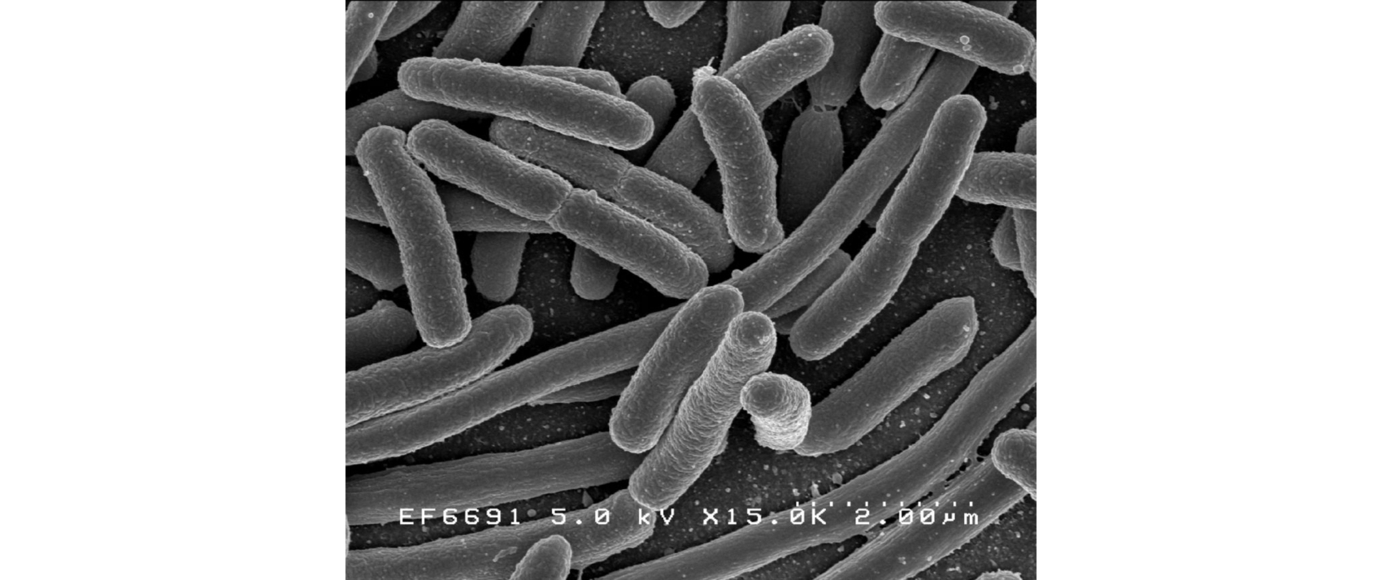Escherichia coli (E. coli) (Wikipedia)

Solution:

The goal is to use the given information to construct a mathematical model based on the formula $P(t)=P0ekt.$

• Step 1: Find the growth rate k. Use the fact that the initial amount, $P0=1,000$ cells, doubles in 20 minutes. That is, $P(t)=2,000$ cells when $t=20$ minutes.

$P(t)=P0ekt2,000=1,000ek20$

Solve for the only variable k.

$2,000=1,000ek202,0001,000=ek202=ek20ln (2)=ln ek20ln (2)=k20ln eln (2)=k20⋅1ln (2)20=k$

• Step 2: Write a mathematical model based on the given information. Here $k≈0.0347$, which is about 3.5% growth rate per minute. However, we will use the exact value for k in our model. This will allow us to avoid round-off error in the final result. Use $P0=1,000$ and $k=ln (2)/20$:

$P(t)=1,000e(ln (2)/20)t$

This equation models the number of E. coli cells in terms of time in minutes.

• Step 3: Use the function to answer the questions. In this case, we are asked to find the number of cells present in 2 hours. Because time is measured in minutes, use $t=120$ minutes to calculate the number of E. coli cells.

$P(120)=1,000e(ln (2)/20)(120)=1,000eln (2)⋅6=1,000eln 26=1,000⋅26=64,000 cells$

Answer: In two hours 64,000 cells will be present.

When the growth rate is negative the function models exponential decay. We can describe decreasing quantities using a half-lifeThe period of time it takes a quantity to decay to one-half of the initial amount., or the time it takes to decay to one-half of a given quantity.

### Example 6

Due to radioactive decay, caesium-137 has a half-life of 30 years. How long will it take a 50-milligram sample to decay to 10 milligrams?

Solution:

Use the half-life information to determine the rate of decay k. In $t=30$ years the initial amount $P0=50$ milligrams will decay to half $P(30)=25$ milligrams.

$P(t)=P0ekt25=50ek30$

Solve for the only variable, k.

$25=50ek302550=e30kln (12)=ln e30kln (12)=30kln eln 1−ln 230=k Recall that ln 1=0.−ln 230=k$

Note that $k=−ln 230≈−0.0231$ is negative. However, we will use the exact value to construct a model that gives the amount of cesium-137 with respect to time in years.

$P(t)=50e(−ln 2/30)t$

Use this model to find t when $P(t)=10$ milligrams.

$10=50e(−ln 2/30)t1050=e(−ln 2/30)tln (15)=ln e(−ln 2/30)tln 1− ln 5=(−ln 230)t ln e Recall that ln e=1.−30(ln 1−ln 5)ln 2=t−30(0−ln 5)ln 2=t30ln 5ln 2=t$

Answer: Using a calculator, it will take $t≈69.66$ years to decay to 10 milligrams.

Radiocarbon dating is a method used to estimate the age of artifacts based on the relative amount of carbon-14 present in it. When an organism dies, it stops absorbing this naturally occurring radioactive isotope, and the carbon-14 begins to decay at a known rate. Therefore, the amount of carbon-14 present in an artifact can be used to estimate the age of the artifact.

### Example 7

An ancient bone tool is found to contain 25% of the carbon-14 normally found in bone. Given that carbon-14 has a half-life of 5,730 years, estimate the age of the tool.

Solution:

Begin by using the half-life information to find k. Here the initial amount $P0$ of carbon-14 is not given, however, we know that in $t=5,730$ years, this amount decays to half, $12P0.$

$P(t)=P0ekt12P0=P0ek5,730$

Dividing both sides by $P0$ leaves us with an exponential equation in terms of k. This shows that half-life is independent of the initial amount.

$12=ek5,730$

Solve for k.

$ln (12)=ln ek5,730ln 1−ln 2=5,730k ln e0−ln 25,730=k−ln 25,730=k$

Therefore we have the model,

$P(t)=P0e(−ln 2/5,730)t$

Next we wish to the find time it takes the carbon-14 to decay to 25% of the initial amount, or $P(t)=0.25P0.$

$0.25P0=P0e(−ln 2/5,730)t$

Divide both sides by $P0$ and solve for t.

$0.25=e(−ln 2/5,730)tln (0.25)=ln e(−ln 2/5,730)tln (0.25)=(−ln 25,730)tln e−5,730 ln (0.25)ln 2=t11,460≈t$

Answer: The tool is approximately 11,460 years old.

Try this! The half-life of strontium-90 is about 28 years. How long will it take a 36 milligram sample of strontium-90 to decay to 30 milligrams?

### Key Takeaways

• When interest is compounded a given number of times per year use the formula $A(t)=P(1+rn)nt.$
• When interest is to be compounded continuously use the formula $A(t)=Pert.$
• Doubling time is the period of time it takes a given amount to double. Doubling time is independent of the principal.
• When amounts are said to be increasing or decaying exponentially, use the formula $P(t)=P0ekt.$
• Half-life is the period of time it takes a given amount to decrease to one-half. Half-life is independent of the initial amount.
• To model data using the exponential growth/decay formula, use the given information to determine the growth/decay rate k. Once k is determined, a formula can be written to model the problem. Use the formula to answer the questions.

### Part A: Compound and Continuous Interest

1. Jill invested \$1,450 in an account earning $458$% annual interest that is compounded monthly.

1. How much will be in the account after 6 years?
2. How long will it take the account to grow to \$2,200?
2. James invested \$825 in an account earning $525$% annual interest that is compounded monthly.

1. How much will be in the account after 4 years?
2. How long will it take the account to grow to \$1,500?
3. Raul invested \$8,500 in an online money market fund earning 4.8% annual interest that is compounded continuously.

1. How much will be in the account after 2 years?
2. How long will it take the account to grow to \$10,000?
4. Ian deposited \$500 in an account earning 3.9% annual interest that is compounded continuously.

1. How much will be in the account after 3 years?
2. How long will it take the account to grow to \$1,500?
5. Bill wants to grow his \$75,000 inheritance to \$100,000 before spending any of it. How long will this take if the bank is offering 5.2% annual interest compounded quarterly?

6. Mary needs \$25,000 for a down payment on a new home. If she invests her savings of \$21,350 in an account earning 4.6% annual interest that is compounded semi-annually, how long will it take to grow to the amount that she needs?

7. Joe invested his \$8,700 savings in an account earning $634$% annual interest that is compounded continuously. How long will it take to earn \$300 in interest?

8. Miriam invested \$12,800 in an account earning $514$% annual interest that is compounded monthly. How long will it take to earn \$1,200 in interest?

9. Given that the bank is offering 4.2% annual interest compounded monthly, what principal is needed to earn \$25,000 in interest for one year?

10. Given that the bank is offering 3.5% annual interest compounded continuously, what principal is needed to earn \$12,000 in interest for one year?

11. Jose invested his \$3,500 bonus in an account earning $512$% annual interest that is compounded quarterly. How long will it take to double his investment?

12. Maria invested her \$4,200 savings in an account earning $634$% annual interest that is compounded semi-annually. How long will it take to double her savings?

13. If money is invested in an account earning 3.85% annual interest that is compounded continuously, how long will it take the amount to double?

14. If money is invested in an account earning 6.82% annual interest that is compounded continuously, how long will it take the amount to double?

15. Find the annual interest rate at which an account earning continuously compounding interest has a doubling time of 9 years.

16. Find the annual interest rate at which an account earning interest that is compounded monthly has a doubling time of 10 years.

17. Alice invested her savings of \$7,000 in an account earning 4.5% annual interest that is compounded monthly. How long will it take the account to triple in value?

18. Mary invested her \$42,000 bonus in an account earning 7.2% annual interest that is compounded continuously. How long will it take the account to triple in value?

19. Calculate the doubling time of an investment made at 7% annual interest that is compounded:

1. monthly
2. continuously
20. Calculate the doubling time of an investment that is earning continuously compounding interest at an annual interest rate of:

1. 4%
2. 6%
21. Billy’s grandfather invested in a savings bond that earned 5.5% annual interest that was compounded annually. Currently, 30 years later, the savings bond is valued at \$10,000. Determine what the initial investment was.

22. In 1935 Frank opened an account earning 3.8% annual interest that was compounded quarterly. He rediscovered this account while cleaning out his garage in 2005. If the account is now worth \$11,294.30, how much was his initial deposit in 1935?

### Part B: Modeling Exponential Growth and Decay

1. The population of a small town of 24,000 people is expected grow exponentially at a rate of 1.6% per year. Construct an exponential growth model and use it to:

1. Estimate the population in 3 years’ time.
2. Estimate the time it will take for the population to reach 30,000 people.
2. During the exponential growth phase, certain bacteria can grow at a rate of 4.1% per hour. If 10,000 cells are initially present in a sample, construct an exponential growth model and use it to:

1. Estimate the population in 5 hours.
2. Estimate the time it will take for the population to reach 25,000 cells.
3. In 2000, the world population was estimated to be 6.115 billion people and in 2010 the estimate was 6.909 billion people. If the world population continues to grow exponentially, estimate the total world population in 2020.

4. In 2000, the population of the United States was estimated to be 282 million people and in 2010 the estimate was 309 million people. If the population of the United States grows exponentially, estimate the population in 2020.

5. An automobile was purchased new for \$42,500 and 2 years later it was valued at \$33,400. Estimate the value of the automobile in 5 years if it continues to decrease exponentially.

6. A new PC was purchased for \$1,200 and in 1.5 years it was worth \$520. Assume the value is decreasing exponentially and estimate the value of the PC four years after it is purchased.

7. The population of the downtown area of a certain city decreased from 12,500 people to 10,200 people in two years. If the population continues to decrease exponentially at this rate, what would we expect the population to be in two more years?

8. A new MP3 player was purchased for \$320 and in 1 year it was selling used online for \$210. If the value continues to decrease exponentially at this rate, determine the value of the MP3 player 3 years after it was purchased.

9. The half-life of radium-226 is about 1,600 years. How long will a 5-milligram sample of radium-226 take to decay to 1 milligram?

10. The half-life of plutonium-239 is about 24,000 years. How long will a 5-milligram sample of plutonium-239 take to decay to 1 milligram?

11. The half-life of radioactive iodine-131 is about 8 days. How long will it take a 28-gram initial sample of iodine-131 to decay to 12 grams?

12. The half-life of caesium-137 is about 30 years. How long will it take a 15-milligram sample of caesium-137 to decay to 5 milligrams?

13. The Rhind Mathematical Papyrus is considered to be the best example of Egyptian mathematics found to date. This ancient papyrus was found to contain 64% of the carbon-14 normally found in papyrus. Given that carbon-14 has a half-life of 5,730 years, estimate the age of the papyrus.

14. A wooden bowl artifact carved from oak was found to contain 55% of the carbon-14 normally found in oak. Given that carbon-14 has a half-life of 5,730 years, estimate the age of the bowl.

15. The half-life of radioactive iodine-131 is about 8 days. How long will it take a sample of iodine-131 to decay to 10% of the original amount?

16. The half-life of caesium-137 is about 30 years. How long will it take a sample of caesium-137 to decay to 25% of the original amount?

17. The half-life of caesium-137 is about 30 years. What percent of an initial sample will remain in 100 years?

18. The half-life of radioactive iodine-131 is about 8 days. What percent of an initial sample will remain in 30 days?

19. If a bone is 100 years old, what percent of its original amount of carbon-14 do we expect to find in it?

20. The half-life of plutonium-239 is about 24,000 years. What percent of an initial sample will remain in 1,000 years?

21. Find the amount of time it will take for 10% of an initial sample of plutonium-239 to decay. (Hint: If 10% decays, then 90% will remain.)

22. Find the amount of time it will take for 10% of an initial sample of carbon-14 to decay.

Solve for the given variable:

1. Solve for t: $A=Pert$

2. Solve for t: $A=P(1+r)t$

3. Solve for I: $M=log (II0)$

4. Solve for $H+$: $pH=−log (H+)$

5. Solve for t: $P=11+e−t$

6. Solve for I: $L=10log (I/10−12)$

7. The number of cells in a certain bacteria sample is approximated by the logistic growth model $N(t)=1.2×1051+9e−0.32t$, where t represents time in hours. Determine the time it takes the sample to grow to 24,000 cells.

8. The market share of a product, as a percentage, is approximated by the formula $P(t)=1003+e−0.44t$ where t represents the number of months after an aggressive advertising campaign is launched.

1. What was the initial market share?
2. How long would we expect to see a 3.5% increase in market share?
9. In chemistry, pH is a measure of acidity and is given by the formula $pH=−log (H+)$, where $H+$ is the hydrogen ion concentration (measured in moles of hydrogen per liter of solution.) What is the hydrogen ion concentration of seawater with a pH of 8?

10. Determine the hydrogen ion concentration of milk with a pH of 6.6.

11. The volume of sound, L in decibels (dB), is given by the formula $L=10log (I/10−12)$ where I represents the intensity of the sound in watts per square meter. Determine the sound intensity of a hair dryer that emits 70 dB of sound.

12. The volume of a chainsaw measures 110 dB. Determine the intensity of this sound.

### Part C: Discussion Board

1. Which factor affects the doubling time the most, the annual compounding n or the interest rate r? Explain.

3. Is exponential growth sustainable over an indefinite amount of time? Explain.

4. Research and discuss the half-life of radioactive materials.

1. \$1,912.73
2. 9 years
1. \$9,356.45
2. 3.4 years
1. 5.6 years

2. $12$ year

3. \$583,867

4. 12.7 years

5. 18 years

6. 7.7%

7. 24.5 years

1. 9.93 years
2. 9.90 years
8. \$2,006.44

3. 8,323 people

4. 3,715 years

5. 9.8 days

7. 26.6 days

8. 9.9%

9. 98.8%

10. 3,648 years

11. $t=ln (A)−ln (P)r$
12. $I=I0⋅10M$

13. $t=ln (P1−P)$
14. Approximately 2.5 hours

15. $10−8$ moles per liter

16. $10−5$ watts per square meter

Given f and g find $(f○g)(x)$ and $(g○f)(x).$
1. $f(x)=6x−5$, $g(x)=2x+1$
2. $f(x$